libxlsxwriter
chart.h File Reference

## Description

The Chart object represents an Excel chart. It provides functions for adding data series to the chart and for configuring the chart.

A Chart object isn't created directly. Instead a chart is created by calling the `workbook_add_chart()` function from a Workbook object. For example:

#include "xlsxwriter.h"
int main() {
lxw_workbook *workbook = new_workbook("chart.xlsx");
// User function to add data to worksheet, not shown here.
write_worksheet_data(worksheet);
// Create a chart object.
// In the simplest case we just add some value data series.
// The NULL categories will default to 1 to 5 like in Excel.
// Insert the chart into the worksheet
worksheet_insert_chart(worksheet, CELL("B7"), chart);
return workbook_close(workbook);
}

The chart in the worksheet will look like this: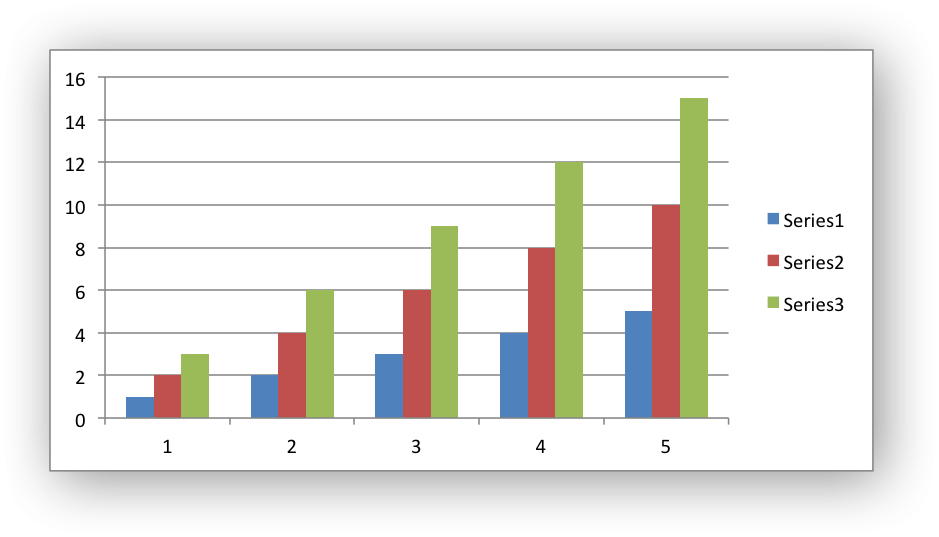The basic procedure for adding a chart to a worksheet is:

1. Create the chart with `workbook_add_chart()`.
2. Add one or more data series to the chart which refers to data in the workbook using `chart_add_series()`.
3. Configure the chart with the other available functions shown below.
4. Insert the chart into a worksheet using `worksheet_insert_chart()`.

## Functions

lxw_chart_serieschart_add_series (lxw_chart *chart, const char *categories, const char *values)
Add a data series to a chart. More...

void chart_series_set_categories (lxw_chart_series *series, const char *sheetname, lxw_row_t first_row, lxw_col_t first_col, lxw_row_t last_row, lxw_col_t last_col)
Set a series "categories" range using row and column values. More...

void chart_series_set_values (lxw_chart_series *series, const char *sheetname, lxw_row_t first_row, lxw_col_t first_col, lxw_row_t last_row, lxw_col_t last_col)
Set a series "values" range using row and column values. More...

void chart_series_set_name (lxw_chart_series *series, const char *name)
Set the name of a chart series range. More...

void chart_series_set_name_range (lxw_chart_series *series, const char *sheetname, lxw_row_t row, lxw_col_t col)
Set a series name formula using row and column values. More...

void chart_series_set_line (lxw_chart_series *series, lxw_chart_line *line)
Set the line properties for a chart series. More...

void chart_series_set_fill (lxw_chart_series *series, lxw_chart_fill *fill)
Set the fill properties for a chart series. More...

void chart_series_set_invert_if_negative (lxw_chart_series *series)
Invert the fill color for negative series values. More...

void chart_series_set_pattern (lxw_chart_series *series, lxw_chart_pattern *pattern)
Set the pattern properties for a chart series. More...

void chart_series_set_marker_type (lxw_chart_series *series, uint8_t type)
Set the data marker type for a series. More...

void chart_series_set_marker_size (lxw_chart_series *series, uint8_t size)
Set the size of a data marker for a series. More...

void chart_series_set_marker_line (lxw_chart_series *series, lxw_chart_line *line)
Set the line properties for a chart series marker. More...

void chart_series_set_marker_fill (lxw_chart_series *series, lxw_chart_fill *fill)
Set the fill properties for a chart series marker. More...

void chart_series_set_marker_pattern (lxw_chart_series *series, lxw_chart_pattern *pattern)
Set the pattern properties for a chart series marker. More...

lxw_error chart_series_set_points (lxw_chart_series *series, lxw_chart_point *points[])
Set the formatting for points in the series. More...

void chart_series_set_smooth (lxw_chart_series *series, uint8_t smooth)
Smooth a line or scatter chart series. More...

void chart_series_set_labels (lxw_chart_series *series)
Add data labels to a chart series. More...

void chart_series_set_labels_options (lxw_chart_series *series, uint8_t show_name, uint8_t show_category, uint8_t show_value)
Set the display options for the labels of a data series. More...

lxw_error chart_series_set_labels_custom (lxw_chart_series *series, lxw_chart_data_label *data_labels[])
Set the properties for data labels in a series. More...

void chart_series_set_labels_separator (lxw_chart_series *series, uint8_t separator)
Set the separator for the data label captions. More...

void chart_series_set_labels_position (lxw_chart_series *series, uint8_t position)
Set the data label position for a series. More...

Set leader lines for Pie and Doughnut charts. More...

void chart_series_set_labels_legend (lxw_chart_series *series)
Set the legend key for a data label in a chart series. More...

void chart_series_set_labels_percentage (lxw_chart_series *series)
Set the percentage for a Pie/Doughnut data point. More...

void chart_series_set_labels_num_format (lxw_chart_series *series, const char *num_format)
Set the number format for chart data labels in a series. More...

void chart_series_set_labels_font (lxw_chart_series *series, lxw_chart_font *font)
Set the font properties for chart data labels in a series. More...

void chart_series_set_labels_line (lxw_chart_series *series, lxw_chart_line *line)
Set the line properties for the data labels in a chart series. More...

void chart_series_set_labels_fill (lxw_chart_series *series, lxw_chart_fill *fill)
Set the fill properties for the data labels in a chart series. More...

void chart_series_set_labels_pattern (lxw_chart_series *series, lxw_chart_pattern *pattern)
Set the pattern properties for the data labels in a chart series. More...

void chart_series_set_trendline (lxw_chart_series *series, uint8_t type, uint8_t value)
Turn on a trendline for a chart data series. More...

void chart_series_set_trendline_forecast (lxw_chart_series *series, double forward, double backward)
Set the trendline forecast for a chart data series. More...

void chart_series_set_trendline_equation (lxw_chart_series *series)
Display the equation of a trendline for a chart data series. More...

void chart_series_set_trendline_r_squared (lxw_chart_series *series)
Display the R squared value of a trendline for a chart data series. More...

void chart_series_set_trendline_intercept (lxw_chart_series *series, double intercept)
Set the trendline Y-axis intercept for a chart data series. More...

void chart_series_set_trendline_name (lxw_chart_series *series, const char *name)
Set the trendline name for a chart data series. More...

void chart_series_set_trendline_line (lxw_chart_series *series, lxw_chart_line *line)
Set the trendline line properties for a chart data series. More...

lxw_series_error_bars * chart_series_get_error_bars (lxw_chart_series *series, lxw_chart_error_bar_axis axis_type)
Get a pointer to X or Y error bars from a chart series. More...

void chart_series_set_error_bars (lxw_series_error_bars *error_bars, uint8_t type, double value)

void chart_series_set_error_bars_direction (lxw_series_error_bars *error_bars, uint8_t direction)
Set the direction (up, down or both) of the error bars for a chart series. More...

void chart_series_set_error_bars_endcap (lxw_series_error_bars *error_bars, uint8_t endcap)
Set the end cap type for the error bars of a chart series. More...

void chart_series_set_error_bars_line (lxw_series_error_bars *error_bars, lxw_chart_line *line)
Set the line properties for a chart series error bars. More...

lxw_chart_axischart_axis_get (lxw_chart *chart, lxw_chart_axis_type axis_type)
Get an axis pointer from a chart. More...

void chart_axis_set_name (lxw_chart_axis *axis, const char *name)
Set the name caption of the an axis. More...

void chart_axis_set_name_range (lxw_chart_axis *axis, const char *sheetname, lxw_row_t row, lxw_col_t col)
Set a chart axis name formula using row and column values. More...

void chart_axis_set_name_font (lxw_chart_axis *axis, lxw_chart_font *font)
Set the font properties for a chart axis name. More...

void chart_axis_set_num_font (lxw_chart_axis *axis, lxw_chart_font *font)
Set the font properties for the numbers of a chart axis. More...

void chart_axis_set_num_format (lxw_chart_axis *axis, const char *num_format)
Set the number format for a chart axis. More...

void chart_axis_set_line (lxw_chart_axis *axis, lxw_chart_line *line)
Set the line properties for a chart axis. More...

void chart_axis_set_fill (lxw_chart_axis *axis, lxw_chart_fill *fill)
Set the fill properties for a chart axis. More...

void chart_axis_set_pattern (lxw_chart_axis *axis, lxw_chart_pattern *pattern)
Set the pattern properties for a chart axis. More...

void chart_axis_set_reverse (lxw_chart_axis *axis)
Reverse the order of the axis categories or values. More...

void chart_axis_set_crossing (lxw_chart_axis *axis, double value)
Set the position that the axis will cross the opposite axis. More...

void chart_axis_set_crossing_max (lxw_chart_axis *axis)
Set the opposite axis crossing position as the axis maximum. More...

void chart_axis_set_crossing_min (lxw_chart_axis *axis)
Set the opposite axis crossing position as the axis minimum. More...

void chart_axis_off (lxw_chart_axis *axis)
Turn off/hide an axis. More...

void chart_axis_set_position (lxw_chart_axis *axis, uint8_t position)
Position a category axis on or between the axis tick marks. More...

void chart_axis_set_label_position (lxw_chart_axis *axis, uint8_t position)
Position the axis labels. More...

void chart_axis_set_label_align (lxw_chart_axis *axis, uint8_t align)
Set the alignment of the axis labels. More...

void chart_axis_set_min (lxw_chart_axis *axis, double min)
Set the minimum value for a chart axis. More...

void chart_axis_set_max (lxw_chart_axis *axis, double max)
Set the maximum value for a chart axis. More...

void chart_axis_set_log_base (lxw_chart_axis *axis, uint16_t log_base)
Set the log base of the axis range. More...

void chart_axis_set_major_tick_mark (lxw_chart_axis *axis, uint8_t type)
Set the major axis tick mark type. More...

void chart_axis_set_minor_tick_mark (lxw_chart_axis *axis, uint8_t type)
Set the minor axis tick mark type. More...

void chart_axis_set_interval_unit (lxw_chart_axis *axis, uint16_t unit)
Set the interval between category values. More...

void chart_axis_set_interval_tick (lxw_chart_axis *axis, uint16_t unit)
Set the interval between category tick marks. More...

void chart_axis_set_major_unit (lxw_chart_axis *axis, double unit)
Set the increment of the major units in the axis. More...

void chart_axis_set_minor_unit (lxw_chart_axis *axis, double unit)
Set the increment of the minor units in the axis. More...

void chart_axis_set_display_units (lxw_chart_axis *axis, uint8_t units)
Set the display units for a value axis. More...

void chart_axis_set_display_units_visible (lxw_chart_axis *axis, uint8_t visible)
Turn on/off the display units for a value axis. More...

void chart_axis_major_gridlines_set_visible (lxw_chart_axis *axis, uint8_t visible)
Turn on/off the major gridlines for an axis. More...

void chart_axis_minor_gridlines_set_visible (lxw_chart_axis *axis, uint8_t visible)
Turn on/off the minor gridlines for an axis. More...

void chart_axis_major_gridlines_set_line (lxw_chart_axis *axis, lxw_chart_line *line)
Set the line properties for the chart axis major gridlines. More...

void chart_axis_minor_gridlines_set_line (lxw_chart_axis *axis, lxw_chart_line *line)
Set the line properties for the chart axis minor gridlines. More...

void chart_title_set_name (lxw_chart *chart, const char *name)
Set the title of the chart. More...

void chart_title_set_name_range (lxw_chart *chart, const char *sheetname, lxw_row_t row, lxw_col_t col)
Set a chart title formula using row and column values. More...

void chart_title_set_name_font (lxw_chart *chart, lxw_chart_font *font)
Set the font properties for a chart title. More...

void chart_title_off (lxw_chart *chart)
Turn off an automatic chart title. More...

void chart_legend_set_position (lxw_chart *chart, uint8_t position)
Set the position of the chart legend. More...

void chart_legend_set_font (lxw_chart *chart, lxw_chart_font *font)
Set the font properties for a chart legend. More...

lxw_error chart_legend_delete_series (lxw_chart *chart, int16_t delete_series[])
Remove one or more series from the the legend. More...

void chart_chartarea_set_line (lxw_chart *chart, lxw_chart_line *line)
Set the line properties for a chartarea. More...

void chart_chartarea_set_fill (lxw_chart *chart, lxw_chart_fill *fill)
Set the fill properties for a chartarea. More...

void chart_chartarea_set_pattern (lxw_chart *chart, lxw_chart_pattern *pattern)
Set the pattern properties for a chartarea. More...

void chart_plotarea_set_line (lxw_chart *chart, lxw_chart_line *line)
Set the line properties for a plotarea. More...

void chart_plotarea_set_fill (lxw_chart *chart, lxw_chart_fill *fill)
Set the fill properties for a plotarea. More...

void chart_plotarea_set_pattern (lxw_chart *chart, lxw_chart_pattern *pattern)
Set the pattern properties for a plotarea. More...

void chart_set_style (lxw_chart *chart, uint8_t style_id)
Set the chart style type. More...

void chart_set_table (lxw_chart *chart)
Turn on a data table below the horizontal axis. More...

void chart_set_table_grid (lxw_chart *chart, uint8_t horizontal, uint8_t vertical, uint8_t outline, uint8_t legend_keys)
Turn on/off grid options for a chart data table. More...

void chart_set_up_down_bars (lxw_chart *chart)
Turn on up-down bars for the chart. More...

void chart_set_up_down_bars_format (lxw_chart *chart, lxw_chart_line *up_bar_line, lxw_chart_fill *up_bar_fill, lxw_chart_line *down_bar_line, lxw_chart_fill *down_bar_fill)
Turn on up-down bars for the chart, with formatting. More...

void chart_set_drop_lines (lxw_chart *chart, lxw_chart_line *line)
Turn on and format Drop Lines for a chart. More...

void chart_set_high_low_lines (lxw_chart *chart, lxw_chart_line *line)
Turn on and format high-low Lines for a chart. More...

void chart_set_series_overlap (lxw_chart *chart, int8_t overlap)
Set the overlap between series in a Bar/Column chart. More...

void chart_set_series_gap (lxw_chart *chart, uint16_t gap)
Set the gap between series in a Bar/Column chart. More...

void chart_show_blanks_as (lxw_chart *chart, uint8_t option)
Set the option for displaying blank data in a chart. More...

void chart_show_hidden_data (lxw_chart *chart)
Display data on charts from hidden rows or columns. More...

void chart_set_rotation (lxw_chart *chart, uint16_t rotation)
Set the Pie/Doughnut chart rotation. More...

void chart_set_hole_size (lxw_chart *chart, uint8_t size)
Set the Doughnut chart hole size. More...

## Function Documentation

 lxw_chart_series* chart_add_series ( lxw_chart * chart, const char * categories, const char * values )
Parameters
 chart Pointer to a lxw_chart instance to be configured. categories The range of categories in the data series. values The range of values in the data series.
Returns
A lxw_chart_series object pointer.

In Excel a chart series is a collection of information that defines which data is plotted such as the categories and values. It is also used to define the formatting for the data.

For an libxlsxwriter chart object the `chart_add_series()` function is used to set the categories and values of the series:

The series parameters are:

• `categories`: This sets the chart category labels. The category is more or less the same as the X axis. In most Excel chart types the `categories` property is optional and the chart will just assume a sequential series from `1..n`:
// The NULL category will default to 1 to 5 like in Excel.
• `values`: This is the most important property of a series and is the only mandatory option for every chart object. This parameter links the chart with the worksheet data that it displays.

The `categories` and `values` should be a string formula like `"=Sheet1!\$A\$2:\$A\$7"` in the same way it is represented in Excel. This is convenient when recreating a chart from an example in Excel but it is trickier to generate programmatically. For these cases you can set the `categories` and `values` to `NULL` and use the `chart_series_set_categories()` and `chart_series_set_values()` functions:

lxw_chart_series *series = chart_add_series(chart, NULL, NULL);
// Configure the series using a syntax that is easier to define programmatically.
chart_series_set_categories(series, "Sheet1", 1, 0, 6, 0); // "=Sheet1!\$A\$2:\$A\$7"
chart_series_set_values( series, "Sheet1", 1, 2, 6, 2); // "=Sheet1!\$C\$2:\$C\$7"

As shown in the previous example the return value from `chart_add_series()` is a lxw_chart_series pointer. This can be used in other functions that configure a series.

More than one series can be added to a chart. The series numbering and order in the Excel chart will be the same as the order in which they are added in libxlsxwriter:

It is also possible to specify non-contiguous ranges:

chart,
"=(Sheet1!\$A\$1:\$A\$9,Sheet1!\$A\$14:\$A\$25)",
"=(Sheet1!\$B\$1:\$B\$9,Sheet1!\$B\$14:\$B\$25)"
);
Examples
chart.c, chart_area.c, chart_bar.c, chart_clustered.c, chart_column.c, chart_data_labels.c, chart_data_table.c, chart_data_tools.c, chart_doughnut.c, chart_fonts.c, chart_line.c, chart_pattern.c, chart_pie.c, chart_radar.c, chart_scatter.c, chart_styles.c, and chartsheet.c.

## ◆ chart_series_set_categories()

 void chart_series_set_categories ( lxw_chart_series * series, const char * sheetname, lxw_row_t first_row, lxw_col_t first_col, lxw_row_t last_row, lxw_col_t last_col )
Parameters
 series A series object created via `chart_add_series()`. sheetname The name of the worksheet that contains the data range. first_row The first row of the range. (All zero indexed.) first_col The first column of the range. last_row The last row of the range. last_col The last col of the range.

The `categories` and `values` of a chart data series are generally set using the `chart_add_series()` function and Excel range formulas like `"=Sheet1!\$A\$2:\$A\$7"`.

The `chart_series_set_categories()` function is an alternative method that is easier to generate programmatically. It requires that you set the `categories` and `values` parameters in `chart_add_series()`to `NULL` and then set them using row and column values in `chart_series_set_categories()` and `chart_series_set_values()`:

lxw_chart_series *series = chart_add_series(chart, NULL, NULL);
// Configure the series ranges programmatically.
chart_series_set_categories(series, "Sheet1", 1, 0, 6, 0); // "=Sheet1!\$A\$2:\$A\$7"
chart_series_set_values( series, "Sheet1", 1, 2, 6, 2); // "=Sheet1!\$C\$2:\$C\$7"
Examples
chart_area.c, chart_bar.c, chart_column.c, chart_data_table.c, chart_line.c, chart_radar.c, chart_scatter.c, and chartsheet.c.

## ◆ chart_series_set_values()

 void chart_series_set_values ( lxw_chart_series * series, const char * sheetname, lxw_row_t first_row, lxw_col_t first_col, lxw_row_t last_row, lxw_col_t last_col )
Parameters
 series A series object created via `chart_add_series()`. sheetname The name of the worksheet that contains the data range. first_row The first row of the range. (All zero indexed.) first_col The first column of the range. last_row The last row of the range. last_col The last col of the range.

The `categories` and `values` of a chart data series are generally set using the `chart_add_series()` function and Excel range formulas like `"=Sheet1!\$A\$2:\$A\$7"`.

The `chart_series_set_values()` function is an alternative method that is easier to generate programmatically. See the documentation for `chart_series_set_categories()` above.

Examples
chart_area.c, chart_bar.c, chart_column.c, chart_data_table.c, chart_line.c, chart_radar.c, chart_scatter.c, and chartsheet.c.

## ◆ chart_series_set_name()

 void chart_series_set_name ( lxw_chart_series * series, const char * name )
Parameters
 series A series object created via `chart_add_series()`. name The series name.

The `chart_series_set_name` function is used to set the name for a chart data series. The series name in Excel is displayed in the chart legend and in the formula bar. The name property is optional and if it isn't supplied it will default to `Series 1..n`.

The function applies to a lxw_chart_series object created using `chart_add_series()`:

lxw_chart_series *series = chart_add_series(chart, NULL, "=Sheet1!\$B\$2:\$B\$7");
chart_series_set_name(series, "Quarterly budget data");

The name parameter can also be a formula such as `=Sheet1!\$A\$1` to point to a cell in the workbook that contains the name:

lxw_chart_series *series = chart_add_series(chart, NULL, "=Sheet1!\$B\$2:\$B\$7");
chart_series_set_name(series, "=Sheet1!\$B\$1");

See also the `chart_series_set_name_range()` function to see how to set the name formula programmatically.

Examples
chart_area.c, chart_bar.c, chart_column.c, chart_data_table.c, chart_doughnut.c, chart_line.c, chart_pattern.c, chart_pie.c, chart_radar.c, chart_scatter.c, and chartsheet.c.

## ◆ chart_series_set_name_range()

 void chart_series_set_name_range ( lxw_chart_series * series, const char * sheetname, lxw_row_t row, lxw_col_t col )
Parameters
 series A series object created via `chart_add_series()`. sheetname The name of the worksheet that contains the cell range. row The zero indexed row number of the range. col The zero indexed column number of the range.

The `chart_series_set_name_range()` function can be used to set a series name range and is an alternative to using `chart_series_set_name()` and a string formula:

lxw_chart_series *series = chart_add_series(chart, NULL, "=Sheet1!\$B\$2:\$B\$7");
chart_series_set_name_range(series, "Sheet1", 0, 2); // "=Sheet1!\$C\$1"
Examples
chart_area.c, chart_bar.c, chart_column.c, chart_data_table.c, chart_line.c, chart_radar.c, chart_scatter.c, and chartsheet.c.

## ◆ chart_series_set_line()

 void chart_series_set_line ( lxw_chart_series * series, lxw_chart_line * line )
Parameters
 series A series object created via `chart_add_series()`. line A lxw_chart_line struct.

Set the line/border properties of a chart series:

chart_series_set_line(series1, &line);
chart_series_set_line(series2, &line);
chart_series_set_line(series3, &line);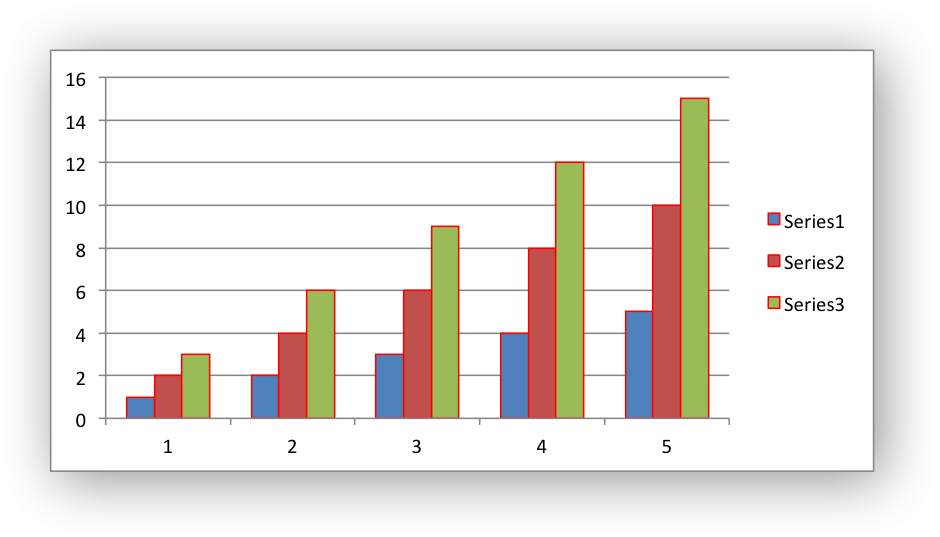Examples
chart_pattern.c.

## ◆ chart_series_set_fill()

 void chart_series_set_fill ( lxw_chart_series * series, lxw_chart_fill * fill )
Parameters
 series A series object created via `chart_add_series()`. fill A lxw_chart_fill struct.

Set the fill properties of a chart series: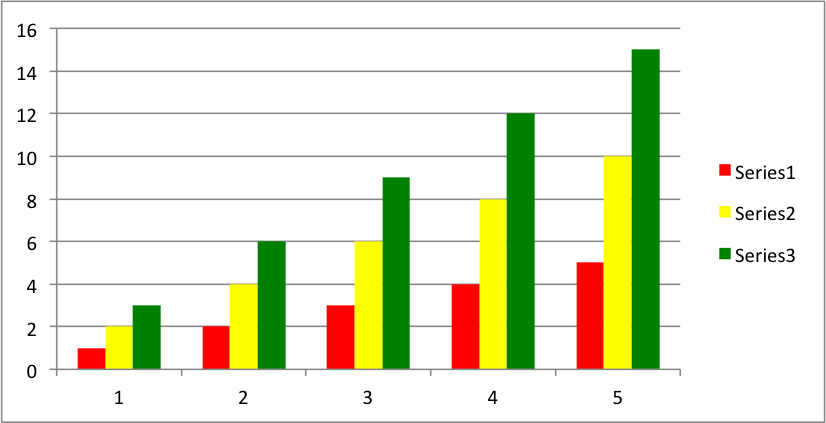## ◆ chart_series_set_invert_if_negative()

 void chart_series_set_invert_if_negative ( lxw_chart_series * series )
Parameters
 series A series object created via `chart_add_series()`.

Invert the fill color for negative values. Usually only applicable to column and bar charts.

## ◆ chart_series_set_pattern()

 void chart_series_set_pattern ( lxw_chart_series * series, lxw_chart_pattern * pattern )
Parameters
 series A series object created via `chart_add_series()`. pattern A lxw_chart_pattern struct.

Set the pattern properties of a chart series:

.fg_color = 0x804000,
.bg_color = 0XC68C53};
.fg_color = 0XB30000,
.bg_color = 0XFF6666};
chart_series_set_pattern(series1, &pattern1);
chart_series_set_pattern(series2, &pattern2);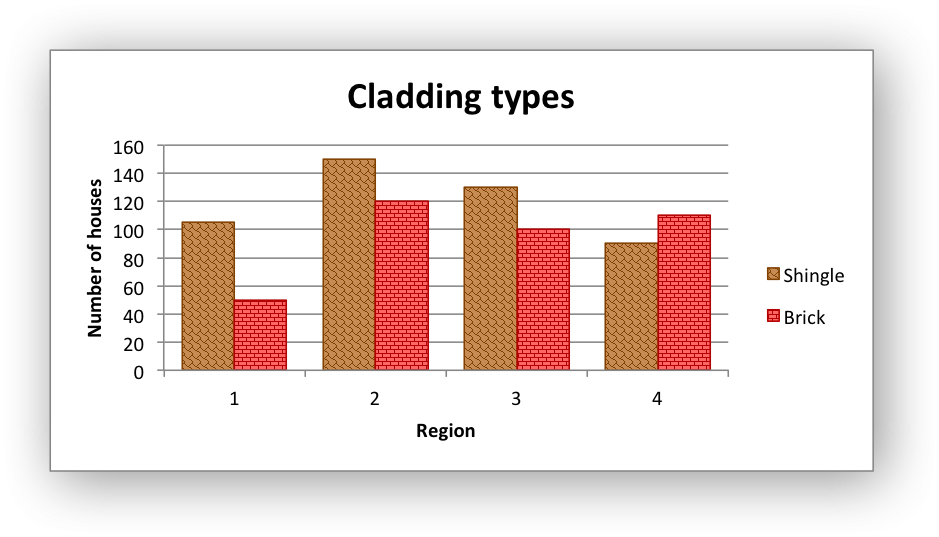Examples
chart_pattern.c.

## ◆ chart_series_set_marker_type()

 void chart_series_set_marker_type ( lxw_chart_series * series, uint8_t type )
Parameters
 series A series object created via `chart_add_series()`. type The marker type, see lxw_chart_marker_type.

In Excel a chart marker is used to distinguish data points in a plotted series. In general only Line and Scatter and Radar chart types use markers. The libxlsxwriter chart types that can have markers are:

The chart types with `MARKERS` in the name have markers with default colors and shapes turned on by default but it is possible using the various `chart_series_set_marker_xxx()` functions below to change these defaults. It is also possible to turn on an off markers.

The `chart_series_set_marker_type()` function is used to specify the type of the series marker: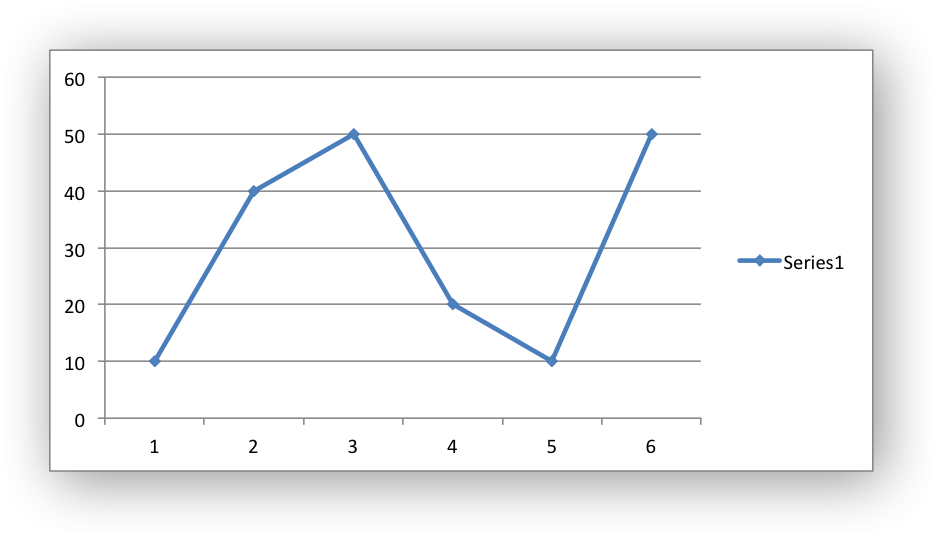The available marker types defined by lxw_chart_marker_type are:

The `#LXW_CHART_MARKER_NONE` type can be used to turn off default markers: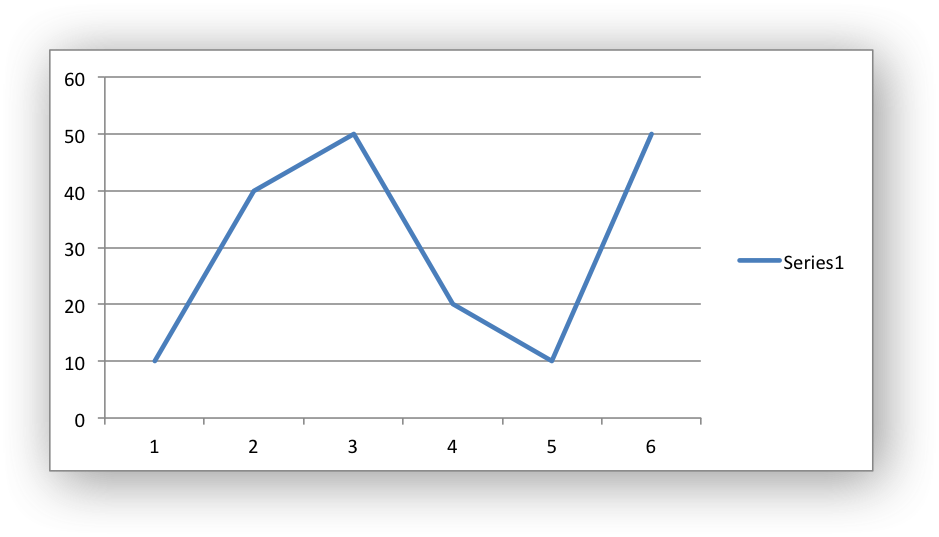The `#LXW_CHART_MARKER_AUTOMATIC` type is a special case which turns on a marker using the default marker style for the particular series. If automatic is on then other marker properties such as size, line or fill cannot be set.

Examples
chart_data_tools.c.

## ◆ chart_series_set_marker_size()

 void chart_series_set_marker_size ( lxw_chart_series * series, uint8_t size )
Parameters
 series A series object created via `chart_add_series()`. size The size of the marker.

The `chart_series_set_marker_size()` function is used to specify the size of the series marker: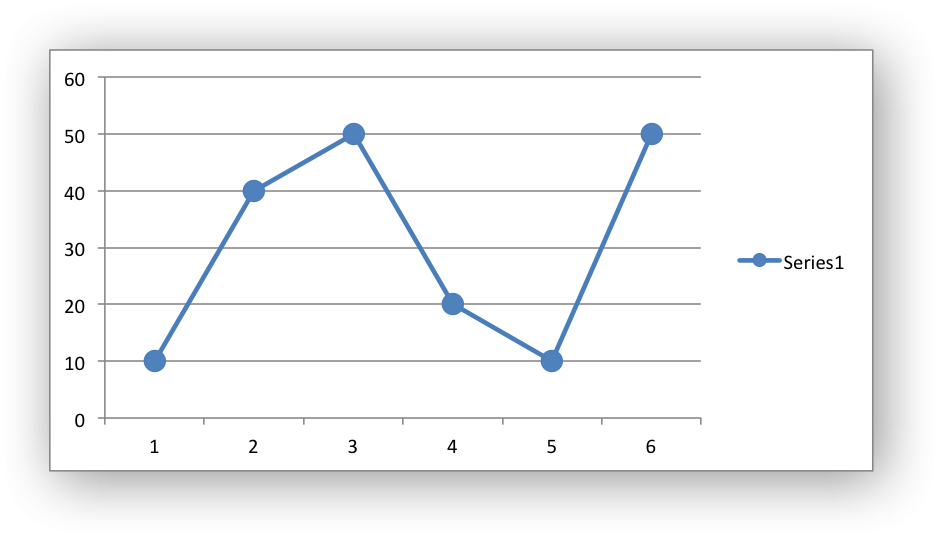## ◆ chart_series_set_marker_line()

 void chart_series_set_marker_line ( lxw_chart_series * series, lxw_chart_line * line )
Parameters
 series A series object created via `chart_add_series()`. line A lxw_chart_line struct.

Set the line/border properties of a chart marker: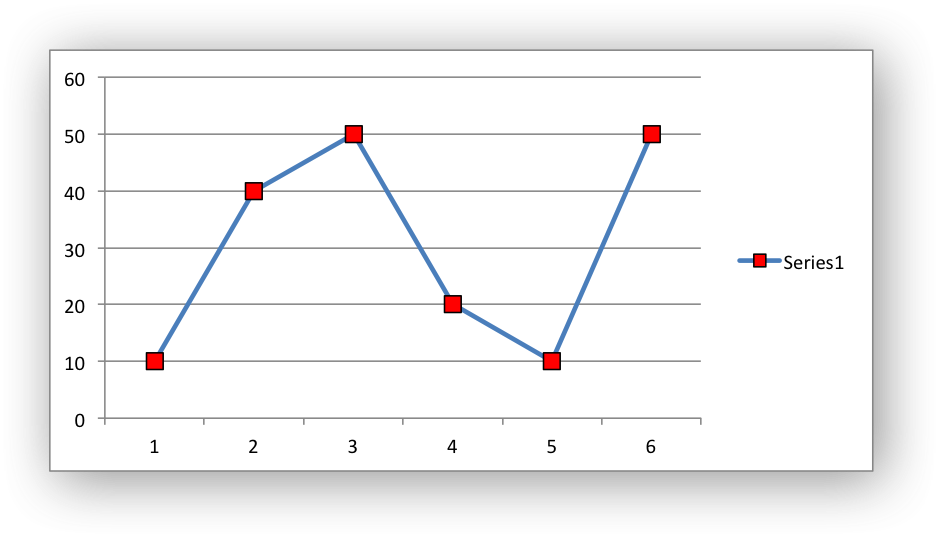## ◆ chart_series_set_marker_fill()

 void chart_series_set_marker_fill ( lxw_chart_series * series, lxw_chart_fill * fill )
Parameters
 series A series object created via `chart_add_series()`. fill A lxw_chart_fill struct.

Set the fill properties of a chart marker:

See the example and image above and also see Chart formatting: Fill.

## ◆ chart_series_set_marker_pattern()

 void chart_series_set_marker_pattern ( lxw_chart_series * series, lxw_chart_pattern * pattern )
Parameters
 series A series object created via `chart_add_series()`. pattern A lxw_chart_pattern struct.

Set the pattern properties of a chart marker:

## ◆ chart_series_set_points()

 lxw_error chart_series_set_points ( lxw_chart_series * series, lxw_chart_point * points[] )
Parameters
 series A series object created via `chart_add_series()`. points An NULL terminated array of lxw_chart_point pointers.
Returns
A lxw_error.

In general formatting is applied to an entire series in a chart. However, it is occasionally required to format individual points in a series. In particular this is required for Pie/Doughnut charts where each segment is represented by a point.

/* Add the data series to the chart. */
"=Sheet1!\$B\$1:\$B\$2");
/* Create some fills for the chart points/segments. */
/* Add the fills to the point objects. */
lxw_chart_point red_point = {.fill = &red_fill };
lxw_chart_point green_point = {.fill = &green_fill};
/* Create an array of pointer to chart points. Note, the array should be
* NULL terminated. */
lxw_chart_point *points[] = {&green_point,
&red_point,
NULL};
/* Add the points to the series. */
chart_series_set_points(series, points);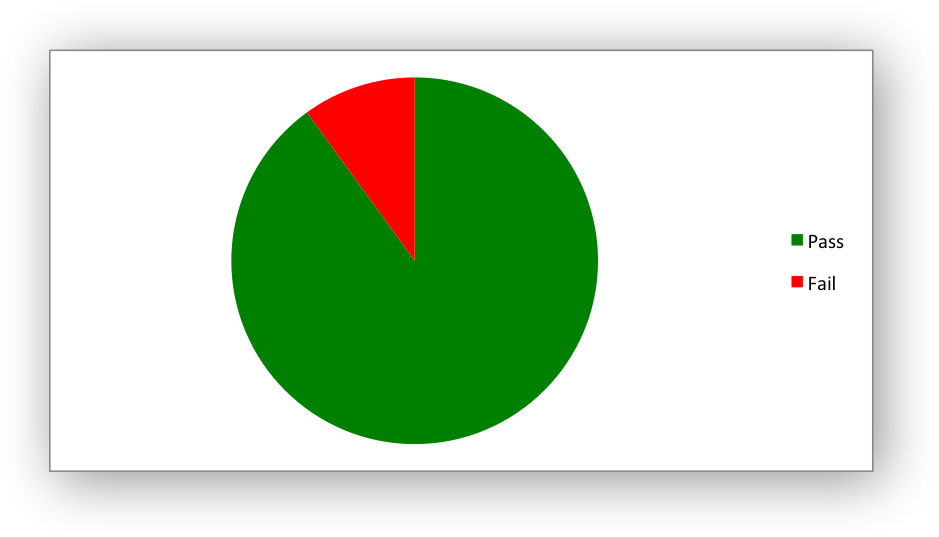Note
The array of lxw_chart_point pointers should be NULL terminated as shown in the example.

For more details see Working with Chart Points

Examples
chart_doughnut.c, and chart_pie.c.

## ◆ chart_series_set_smooth()

 void chart_series_set_smooth ( lxw_chart_series * series, uint8_t smooth )
Parameters
 series A series object created via `chart_add_series()`. smooth Turn off/on the line smoothing. (0/1)

The `chart_series_set_smooth()` function is used to set the smooth property of a line series. It is only applicable to the line and scatter chart types: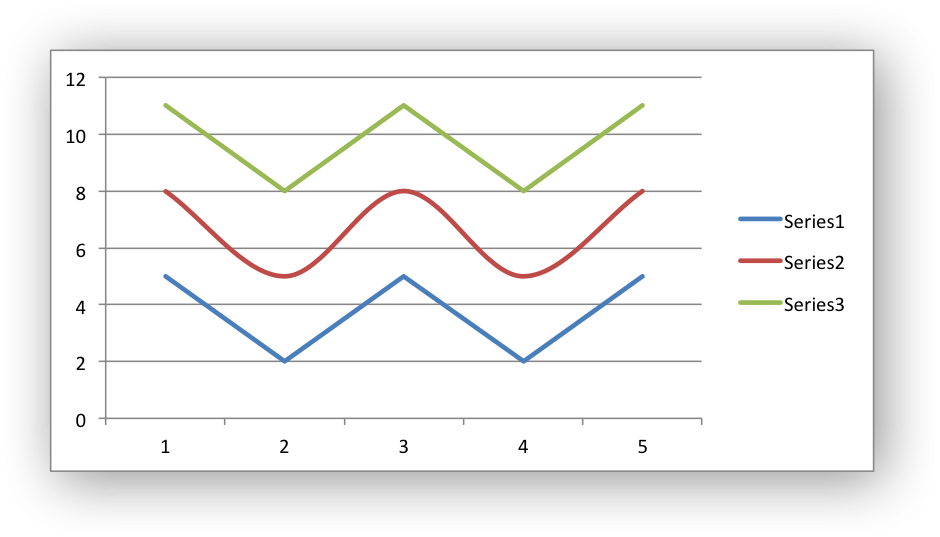## ◆ chart_series_set_labels()

 void chart_series_set_labels ( lxw_chart_series * series )
Parameters
 series A series object created via `chart_add_series()`.

The `chart_series_set_labels()` function is used to turn on data labels for a chart series. Data labels indicate the values of the plotted data points.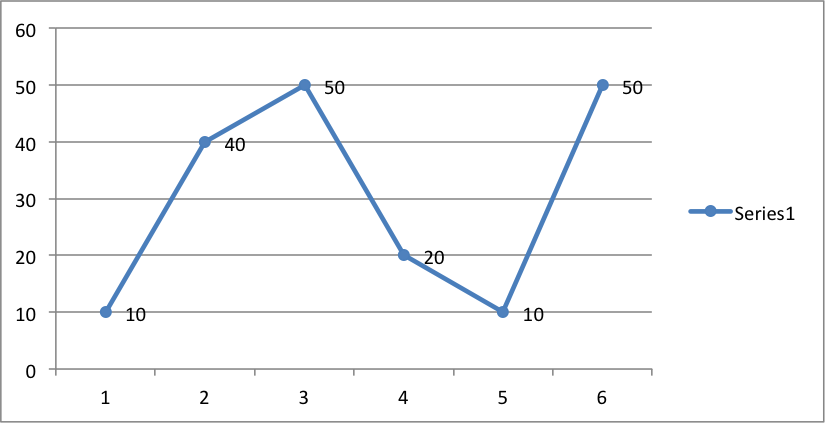By default data labels are displayed in Excel with only the values shown: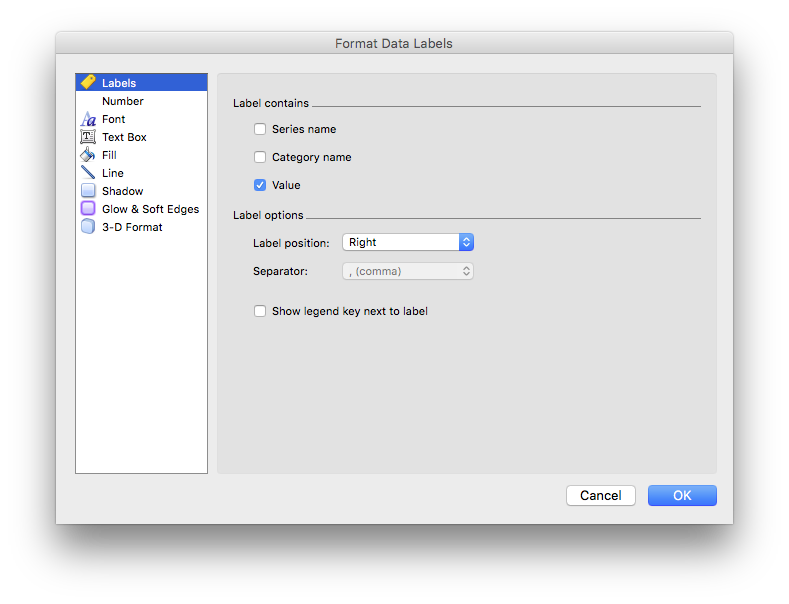However, it is possible to configure other display options, as shown in the functions below.

Examples
chart_data_labels.c, and chart_data_tools.c.

## ◆ chart_series_set_labels_options()

 void chart_series_set_labels_options ( lxw_chart_series * series, uint8_t show_name, uint8_t show_category, uint8_t show_value )
Parameters
 series A series object created via `chart_add_series()`. show_name Turn on/off the series name in the label caption. show_category Turn on/off the category name in the label caption. show_value Turn on/off the value in the label caption.

The `chart_series_set_labels_options()` function is used to set the parameters that are displayed in the series data label: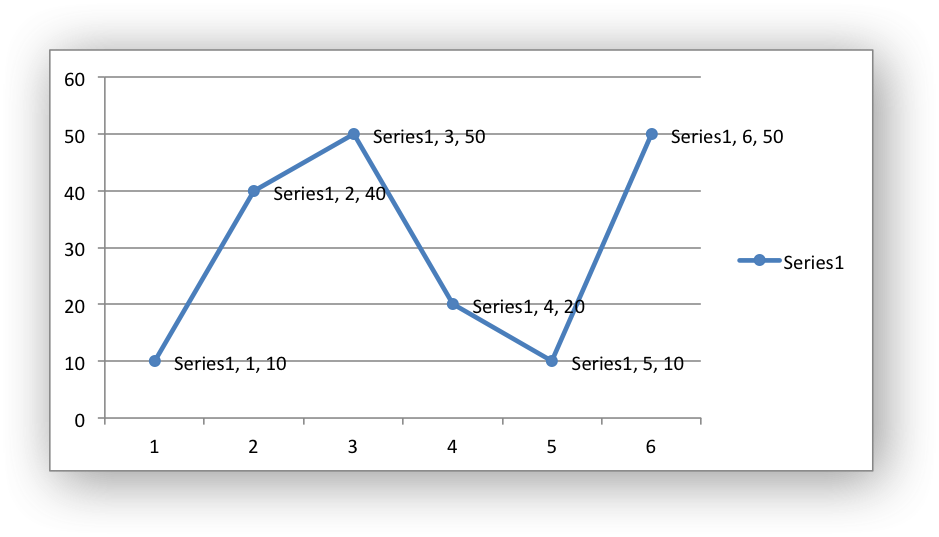Examples
chart_data_labels.c.

## ◆ chart_series_set_labels_custom()

 lxw_error chart_series_set_labels_custom ( lxw_chart_series * series, lxw_chart_data_label * data_labels[] )
Parameters
 series A series object created via `chart_add_series()`. data_labels An NULL terminated array of lxw_chart_data_label pointers.
Returns
A lxw_error.

The `chart_series_set_labels_custom()` function is used to set the properties for data labels in a series. It can also be used to delete individual data labels in a series.

In general properties are set for all the data labels in a chart series. However, it is also possible to set properties for individual data labels in a series using `chart_series_set_labels_custom()`.

The `chart_series_set_labels_custom()` function takes a pointer to an array of lxw_chart_data_label pointers. The list should be `NULL` terminated:

// Add the series data labels.
// Create some custom labels.
lxw_chart_data_label data_label1 = {.value = "Jan"};
lxw_chart_data_label data_label2 = {.value = "Feb"};
lxw_chart_data_label data_label3 = {.value = "Mar"};
lxw_chart_data_label data_label4 = {.value = "Apr"};
lxw_chart_data_label data_label5 = {.value = "May"};
lxw_chart_data_label data_label6 = {.value = "Jun"};
// Create an array of label pointers. NULL indicates the end of the array.
lxw_chart_data_label *data_labels[] = {
&data_label1,
&data_label2,
&data_label3,
&data_label4,
&data_label5,
&data_label6,
NULL
};
// Set the custom labels.
chart_series_set_labels_custom(series, data_labels);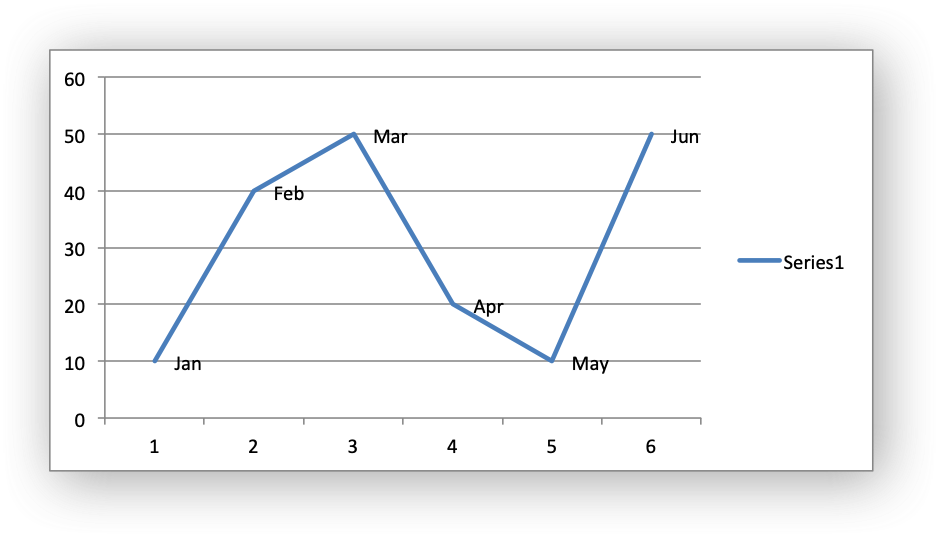Note
The array of lxw_chart_point pointers should be NULL terminated as shown in the example. Any lxw_chart_data_label items set to a default initialization or omitted from the list will be assigned the default data label value.

For more details see Custom Chart Data Labels.

Examples
chart_data_labels.c.

## ◆ chart_series_set_labels_separator()

 void chart_series_set_labels_separator ( lxw_chart_series * series, uint8_t separator )
Parameters
 series A series object created via `chart_add_series()`. separator The separator for the data label options: lxw_chart_label_separator.

The `chart_series_set_labels_separator()` function is used to change the separator between multiple data label items. The default options is a comma separator as shown in the previous example.

The available options are:

For example: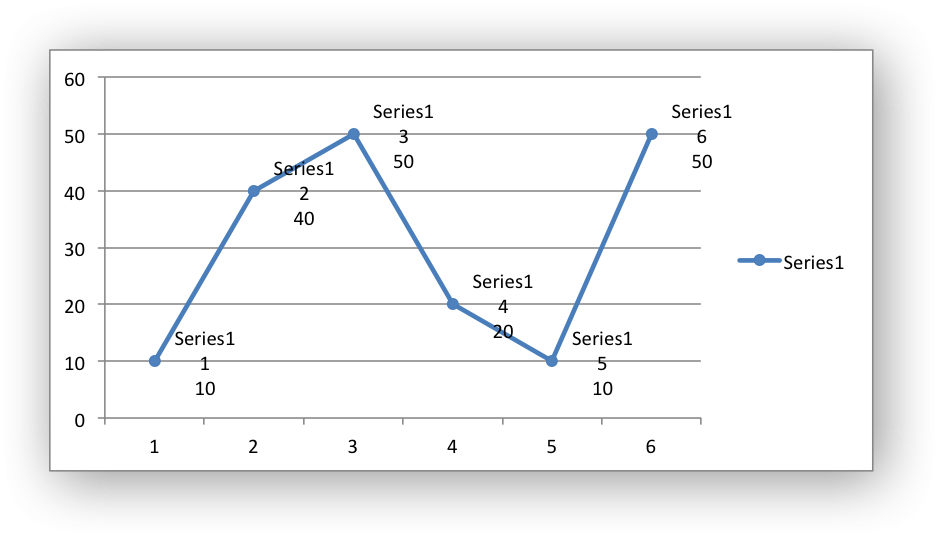## ◆ chart_series_set_labels_position()

 void chart_series_set_labels_position ( lxw_chart_series * series, uint8_t position )
Parameters
 series A series object created via `chart_add_series()`. position The data label position: lxw_chart_label_position.

The `chart_series_set_labels_position()` function sets the position of the labels in the data series: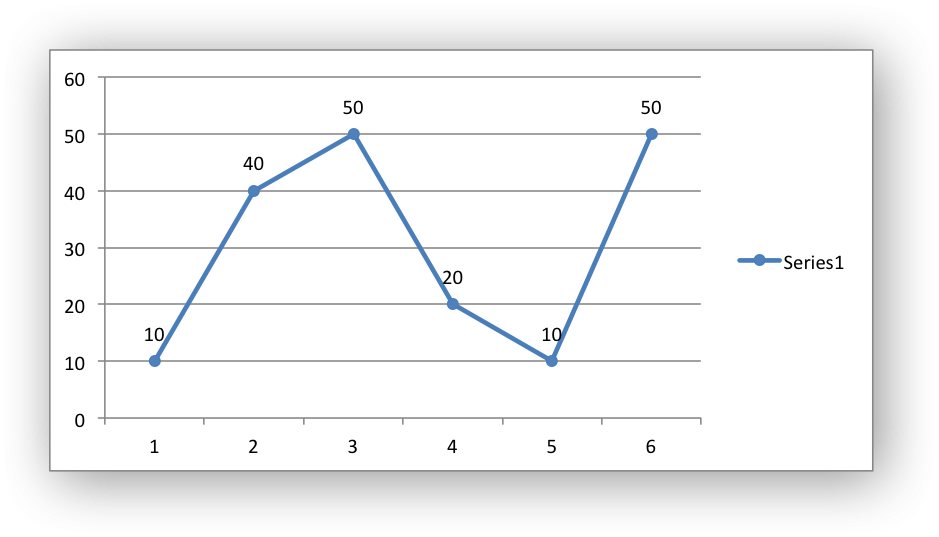In Excel the allowable data label positions vary for different chart types. The allowable, and default, positions are:

Position Line, Scatter Bar, Column Pie, Doughnut Area, Radar
LXW_CHART_LABEL_POSITION_CENTER Yes Yes Yes Yes (default)
LXW_CHART_LABEL_POSITION_RIGHT Yes (default)
LXW_CHART_LABEL_POSITION_LEFT Yes
LXW_CHART_LABEL_POSITION_ABOVE Yes
LXW_CHART_LABEL_POSITION_BELOW Yes
LXW_CHART_LABEL_POSITION_INSIDE_BASE Yes
LXW_CHART_LABEL_POSITION_INSIDE_END Yes Yes
LXW_CHART_LABEL_POSITION_OUTSIDE_END Yes (default) Yes
LXW_CHART_LABEL_POSITION_BEST_FIT Yes (default)

 void chart_series_set_labels_leader_line ( lxw_chart_series * series )
Parameters
 series A series object created via `chart_add_series()`.

The `chart_series_set_labels_leader_line()` function is used to turn on leader lines for the data label of a series. It is mainly used for pie or doughnut charts:

Note
Even when leader lines are turned on they aren't automatically visible in Excel or XlsxWriter. Due to an Excel limitation (or design) leader lines only appear if the data label is moved manually or if the data labels are very close and need to be adjusted automatically.

## ◆ chart_series_set_labels_legend()

 void chart_series_set_labels_legend ( lxw_chart_series * series )
Parameters
 series A series object created via `chart_add_series()`.

The `chart_series_set_labels_legend()` function is used to set the legend key for a data series: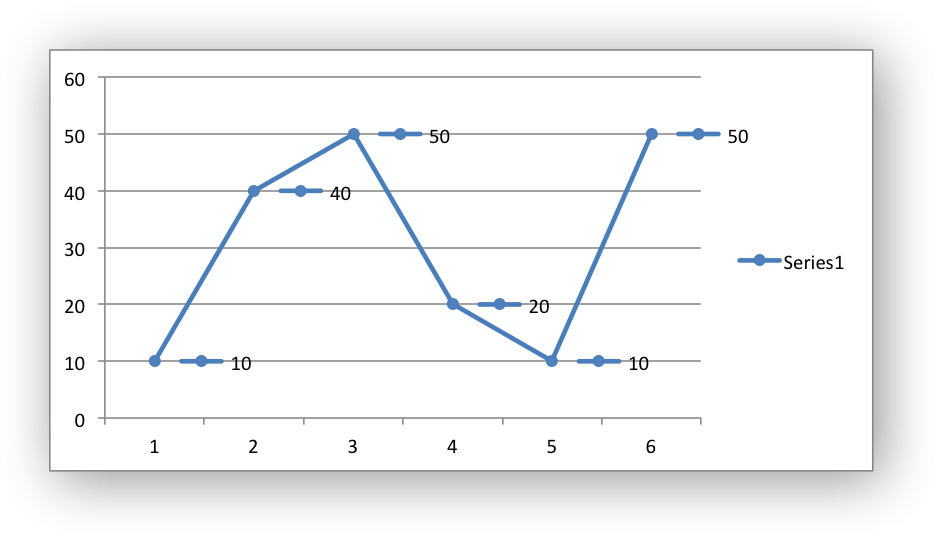## ◆ chart_series_set_labels_percentage()

 void chart_series_set_labels_percentage ( lxw_chart_series * series )
Parameters
 series A series object created via `chart_add_series()`.

The `chart_series_set_labels_percentage()` function is used to turn on the display of data labels as a percentage for a series. It is mainly used for pie charts: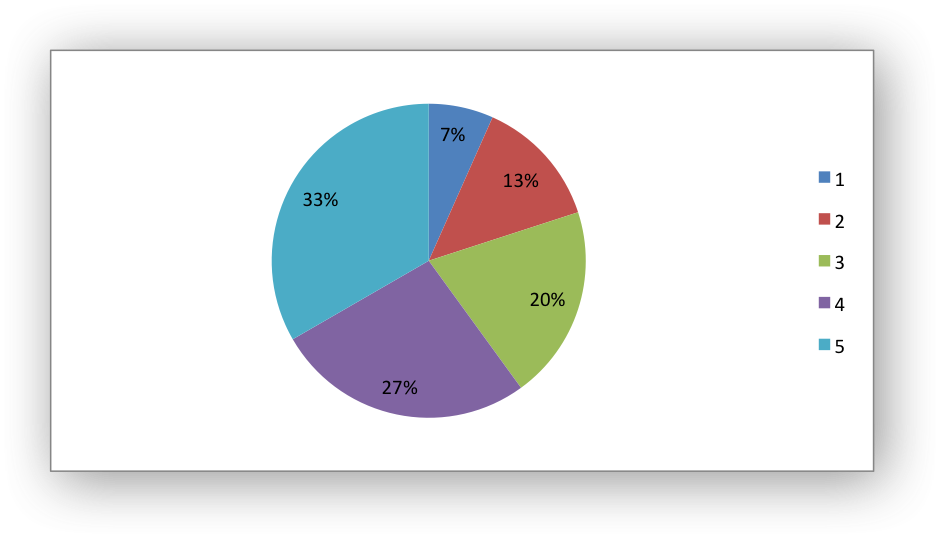## ◆ chart_series_set_labels_num_format()

 void chart_series_set_labels_num_format ( lxw_chart_series * series, const char * num_format )
Parameters
 series A series object created via `chart_add_series()`. num_format The number format string.

The `chart_series_set_labels_num_format()` function is used to set the number format for data labels: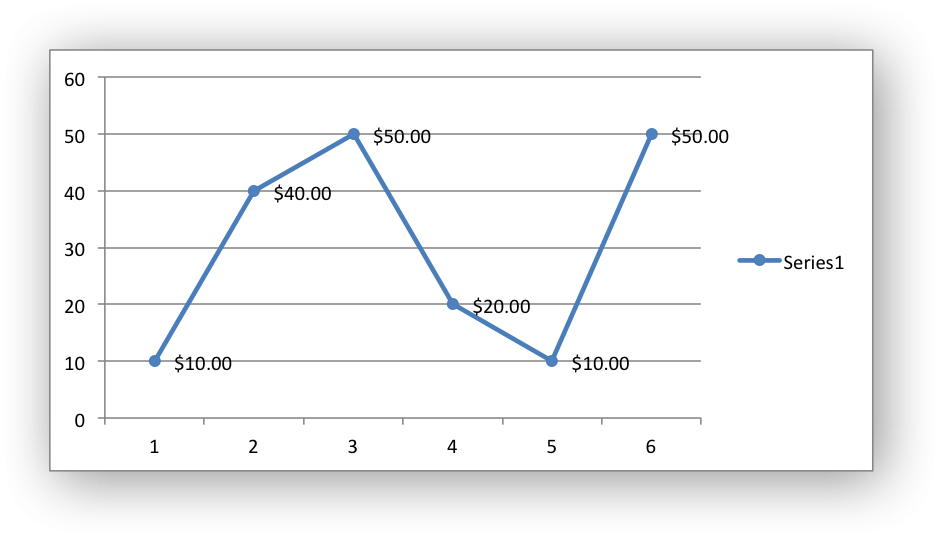The number format is similar to the Worksheet Cell Format num_format, see `format_set_num_format()`.

## ◆ chart_series_set_labels_font()

 void chart_series_set_labels_font ( lxw_chart_series * series, lxw_chart_font * font )
Parameters
 series A series object created via `chart_add_series()`. font A pointer to a chart lxw_chart_font font struct.

The `chart_series_set_labels_font()` function is used to set the font for data labels:

lxw_chart_font font = {.name = "Consolas", .color = LXW_COLOR_RED};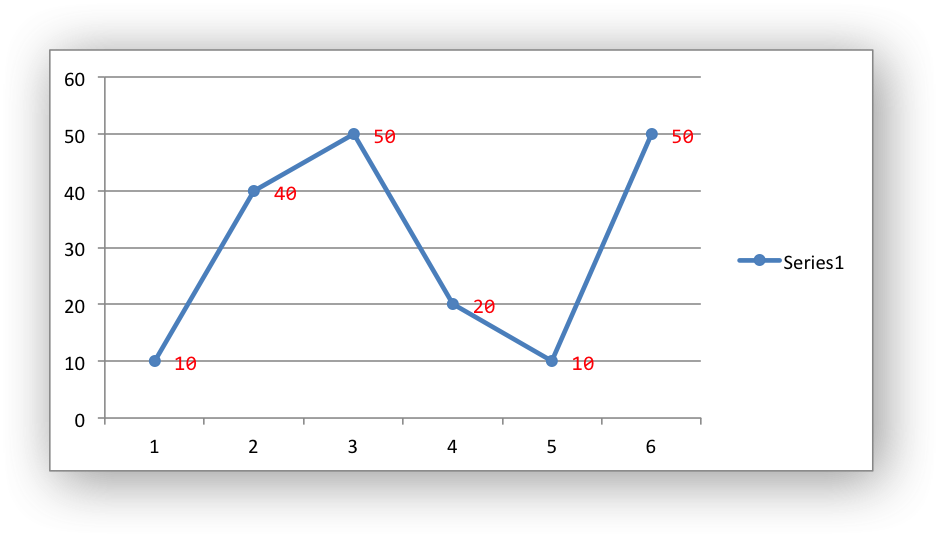For more information see Chart formatting: Fonts and Working with Chart Data Labels.

Examples
chart_data_labels.c.

## ◆ chart_series_set_labels_line()

 void chart_series_set_labels_line ( lxw_chart_series * series, lxw_chart_line * line )
Parameters
 series A series object created via `chart_add_series()`. line A lxw_chart_line struct.

Set the line/border properties of the data labels in a chart series: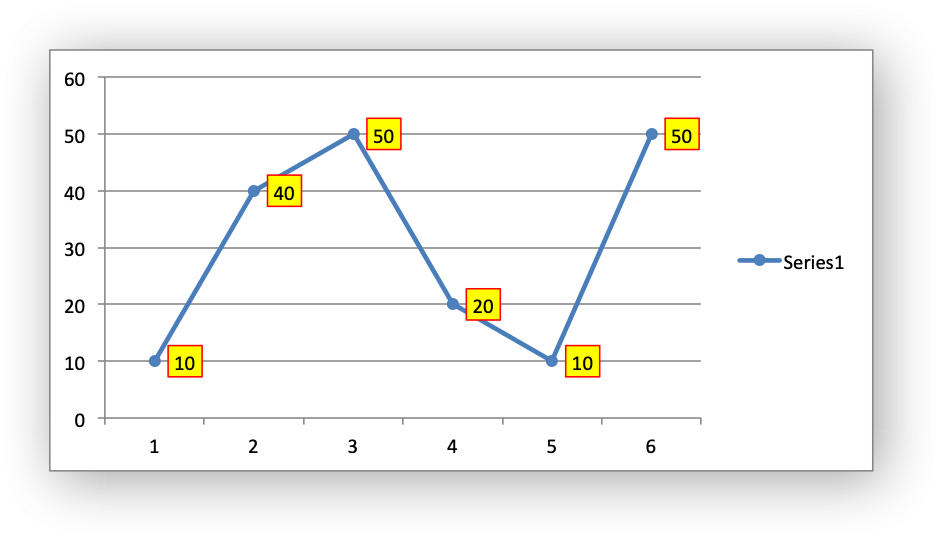For more information see Chart formatting: Line and Working with Chart Data Labels.

Examples
chart_data_labels.c.

## ◆ chart_series_set_labels_fill()

 void chart_series_set_labels_fill ( lxw_chart_series * series, lxw_chart_fill * fill )
Parameters
 series A series object created via `chart_add_series()`. fill A lxw_chart_fill struct.

Set the fill properties of the data labels in a chart series:

See the example and image above and also see Chart formatting: Fill and Working with Chart Data Labels.

Examples
chart_data_labels.c.

## ◆ chart_series_set_labels_pattern()

 void chart_series_set_labels_pattern ( lxw_chart_series * series, lxw_chart_pattern * pattern )
Parameters
 series A series object created via `chart_add_series()`. pattern A lxw_chart_pattern struct.

Set the pattern properties of the data labels in a chart series:

## ◆ chart_series_set_trendline()

 void chart_series_set_trendline ( lxw_chart_series * series, uint8_t type, uint8_t value )
Parameters
 series A series object created via `chart_add_series()`. type The type of trendline: lxw_chart_trendline_type. value The order/period value for polynomial and moving average trendlines.

A trendline can be added to a chart series to indicate trends in the data such as a moving average or a polynomial fit. The trendlines types are shown in the following Excel dialog: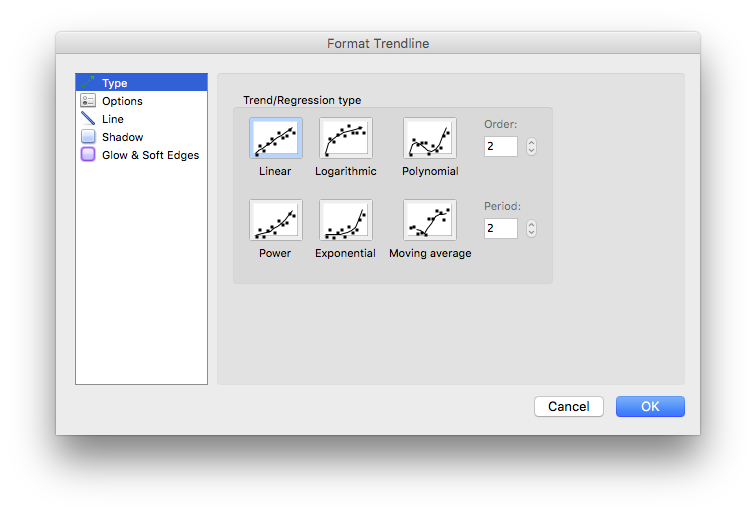The `chart_series_set_trendline()` function turns on these trendlines for a data series: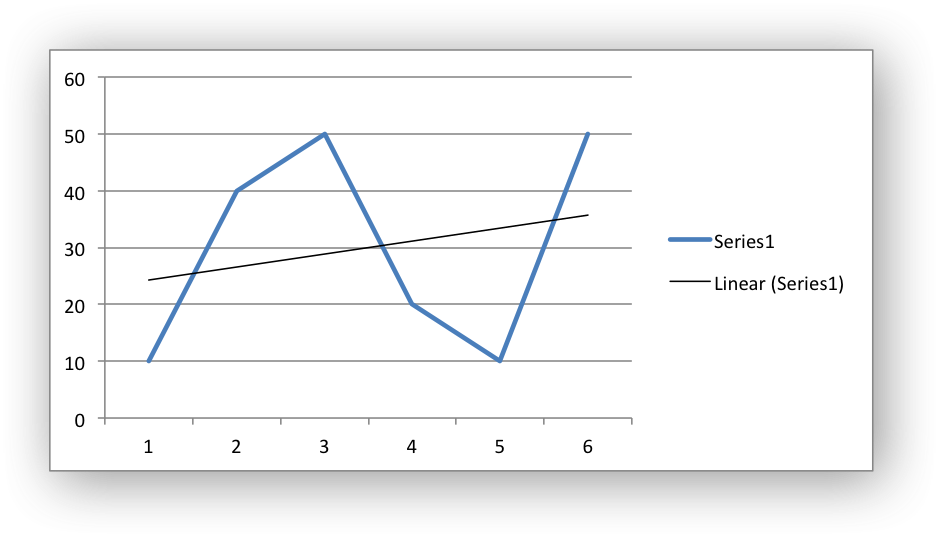The `value` parameter corresponds to order for a polynomial trendline and period for a Moving Average trendline. It both cases it must be >= 2. The `value` parameter is ignored for all other trendlines: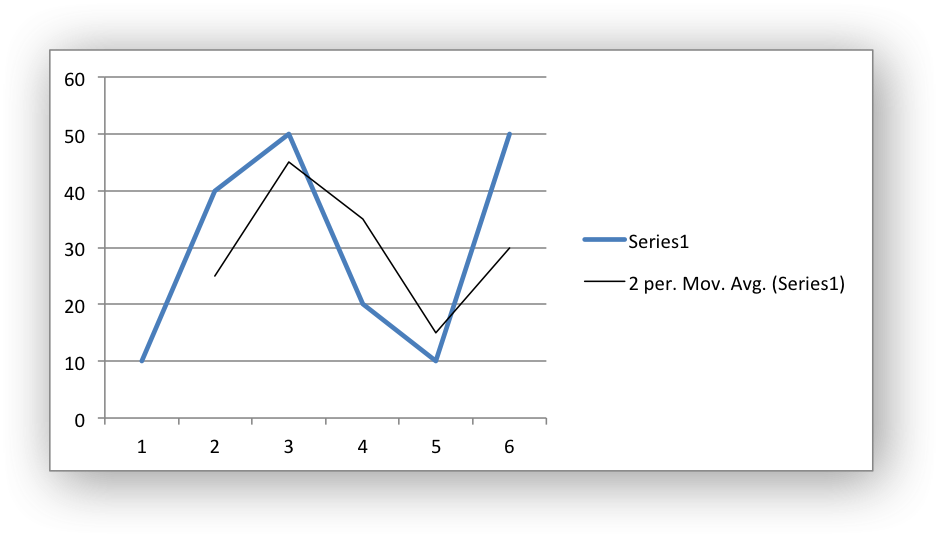The allowable values for the the trendline `type` are:

Other trendline options, such as those shown in the following Excel dialog, can be set using the functions below.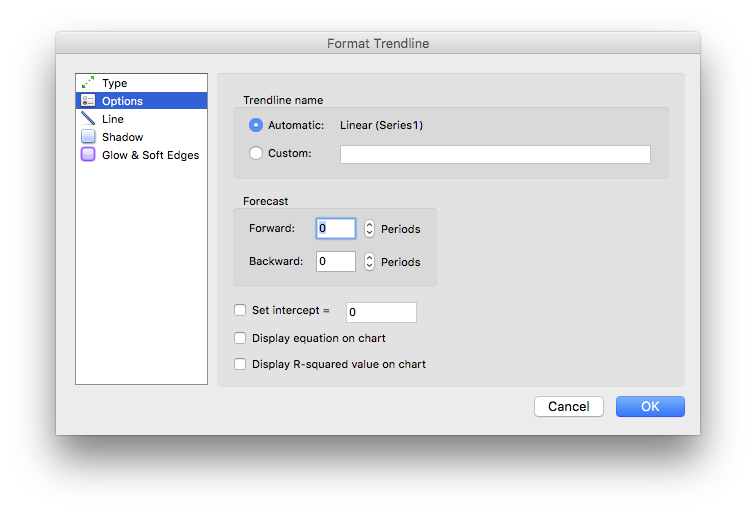Examples
chart_data_tools.c.

## ◆ chart_series_set_trendline_forecast()

 void chart_series_set_trendline_forecast ( lxw_chart_series * series, double forward, double backward )
Parameters
 series A series object created via `chart_add_series()`. forward The forward period. backward The backwards period.

The `chart_series_set_trendline_forecast()` function sets the forward and backward forecast periods for the trendline: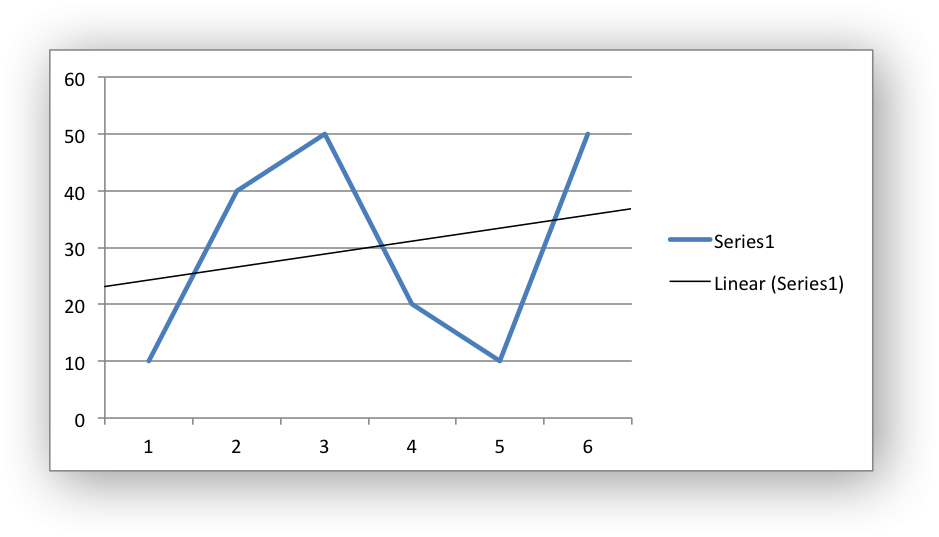Note
This feature isn't available for Moving Average in Excel.

## ◆ chart_series_set_trendline_equation()

 void chart_series_set_trendline_equation ( lxw_chart_series * series )
Parameters
 series A series object created via `chart_add_series()`.

The `chart_series_set_trendline_equation()` function displays the equation of the trendline on the chart: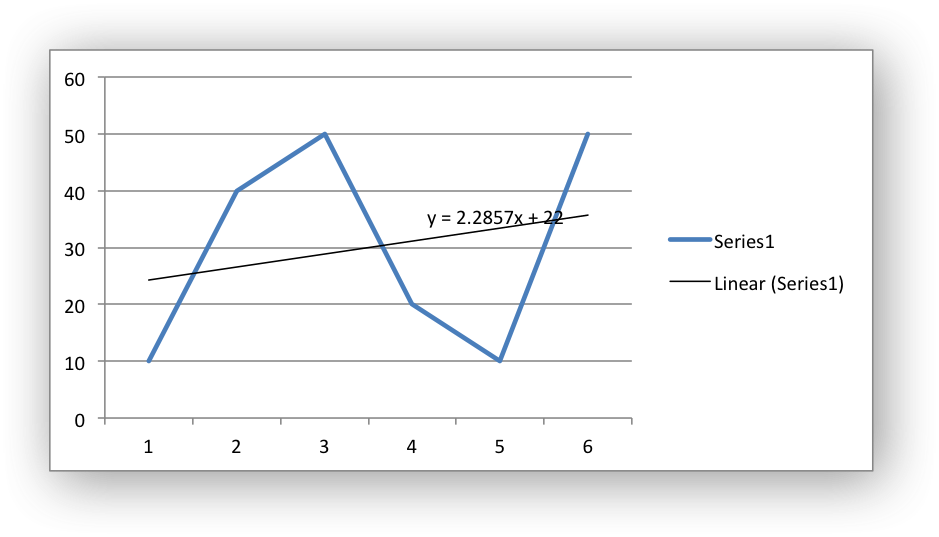Note
This feature isn't available for Moving Average in Excel.

## ◆ chart_series_set_trendline_r_squared()

 void chart_series_set_trendline_r_squared ( lxw_chart_series * series )
Parameters
 series A series object created via `chart_add_series()`.

The `chart_series_set_trendline_r_squared()` function displays the R-squared value for the trendline on the chart: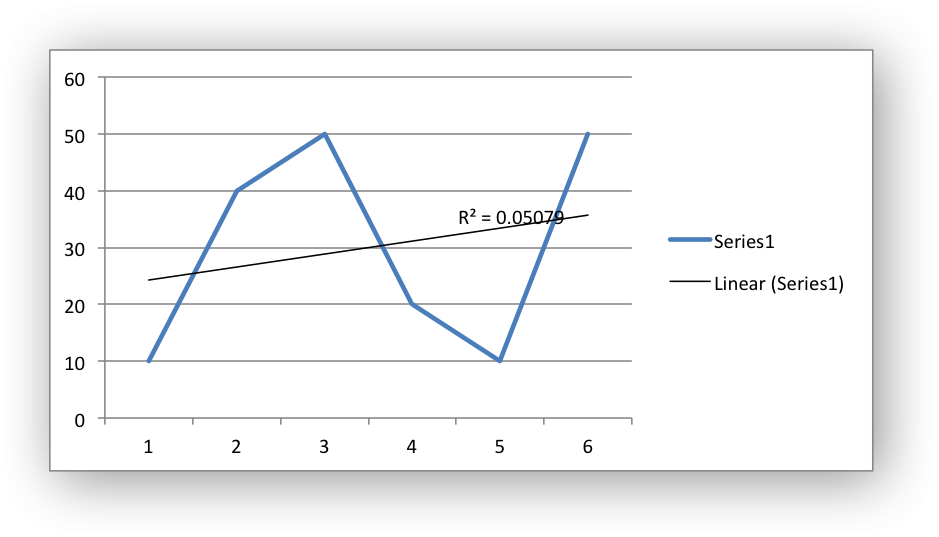Note
This feature isn't available for Moving Average in Excel.

## ◆ chart_series_set_trendline_intercept()

 void chart_series_set_trendline_intercept ( lxw_chart_series * series, double intercept )
Parameters
 series A series object created via `chart_add_series()`. intercept Y-axis intercept value.

The `chart_series_set_trendline_intercept()` function sets the Y-axis intercept for the trendline: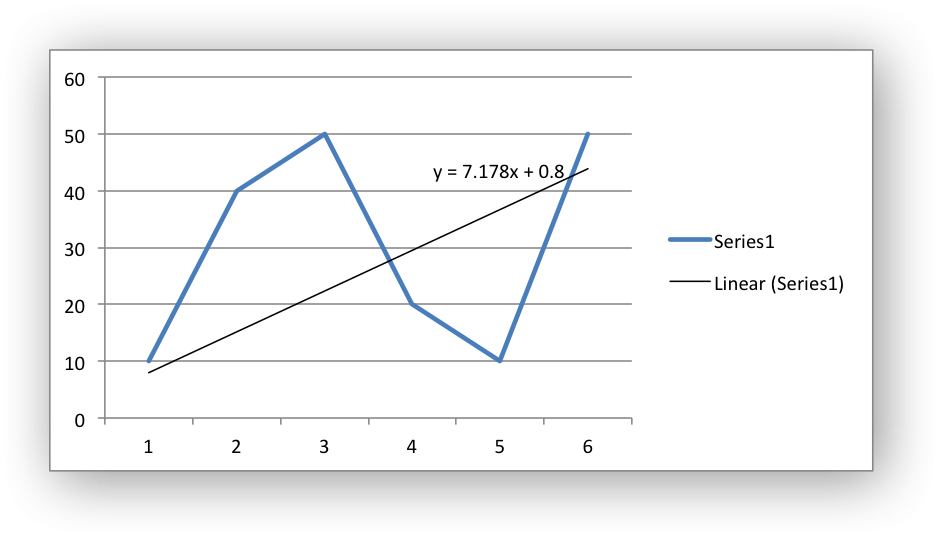As can be seen from the equation on the chart the intercept point (when X=0) is the same as the value set in the equation.

Note
The intercept feature is only available in Excel for Exponential, Linear and Polynomial trendline types.

## ◆ chart_series_set_trendline_name()

 void chart_series_set_trendline_name ( lxw_chart_series * series, const char * name )
Parameters
 series A series object created via `chart_add_series()`. name The name of the trendline to display in the legend.

The `chart_series_set_trendline_name()` function sets the name of the trendline that is displayed in the chart legend. In the examples above the trendlines are displayed with default names like "Linear (Series 1)" and "2 per Mov. Avg. (Series 1)". If these names are too verbose or not descriptive enough you can set your own trendline name:

chart_series_set_trendline_name(series, "My trendline");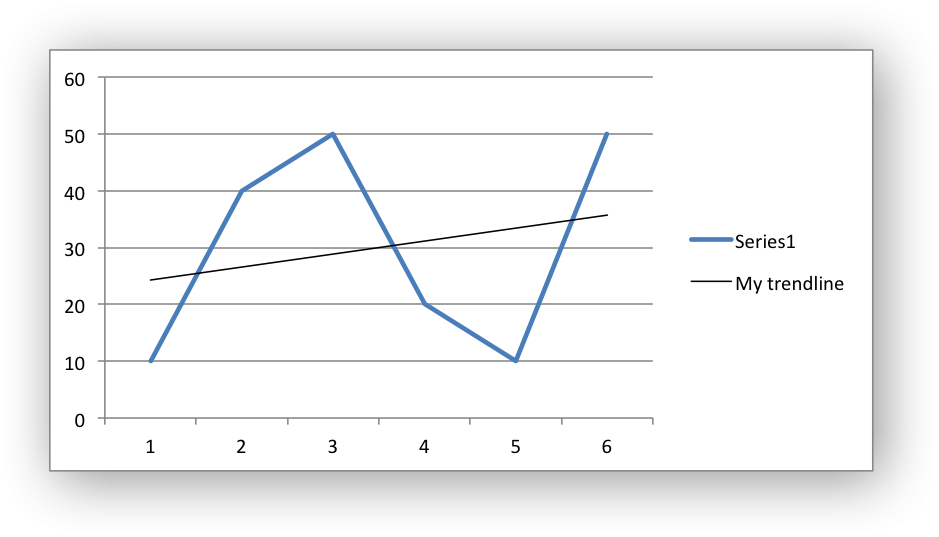It is often preferable to turn off the trendline caption in the legend. This is down in Excel by deleting the trendline name from the legend. In libxlsxwriter this is done using the `chart_legend_delete_series()` function to delete the zero based series numbers:

// Delete the series name for the second series (=1 in zero base).
// The -1 value indicates the end of the array of values.
int16_t names[] = {1, -1};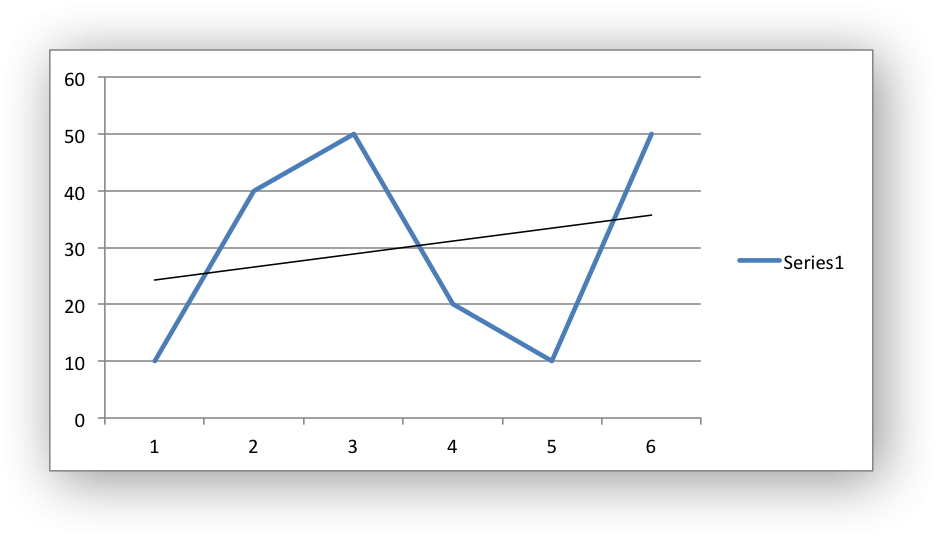## ◆ chart_series_set_trendline_line()

 void chart_series_set_trendline_line ( lxw_chart_series * series, lxw_chart_line * line )
Parameters
 series A series object created via `chart_add_series()`. line A lxw_chart_line struct.

The `chart_series_set_trendline_line()` function is used to set the line properties of a trendline: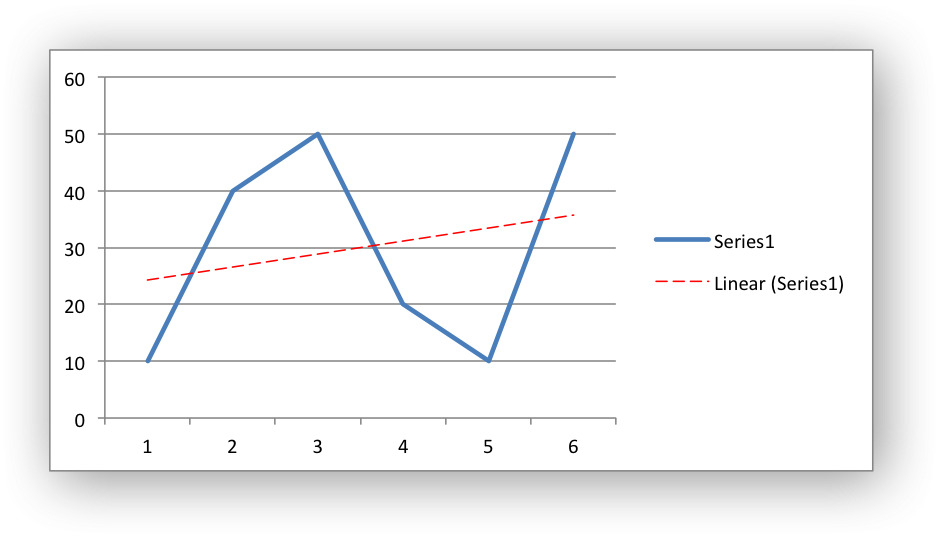For more information see Working with Chart Trendlines and Chart formatting: Line.

Examples
chart_data_tools.c.

## ◆ chart_series_get_error_bars()

 lxw_series_error_bars* chart_series_get_error_bars ( lxw_chart_series * series, lxw_chart_error_bar_axis axis_type )
Parameters
 series A series object created via `chart_add_series()`. axis_type The axis type (X or Y): lxw_chart_error_bar_axis.

The `chart_series_get_error_bars()` function returns a pointer to the error bars of a series based on the type of lxw_chart_error_bar_axis:

lxw_series_error_bars *x_error_bars;
lxw_series_error_bars *y_error_bars;
// Use the error bar pointers.

Note, the series error bars can also be accessed directly:

// Equivalent to the above example, without function calls.
chart_series_set_error_bars(series->x_error_bars,
chart_series_set_error_bars(series->y_error_bars,
Returns

## ◆ chart_series_set_error_bars()

 void chart_series_set_error_bars ( lxw_series_error_bars * error_bars, uint8_t type, double value )

Set the X or Y error bars for a chart series.

Parameters
 error_bars A pointer to the series X or Y error bars. type The type of error bar: lxw_chart_error_bar_type. value The error value.

Error bars can be added to a chart series to indicate error bounds in the data. The error bars can be vertical `y_error_bars` (the most common type) or horizontal `x_error_bars` (for Bar and Scatter charts only).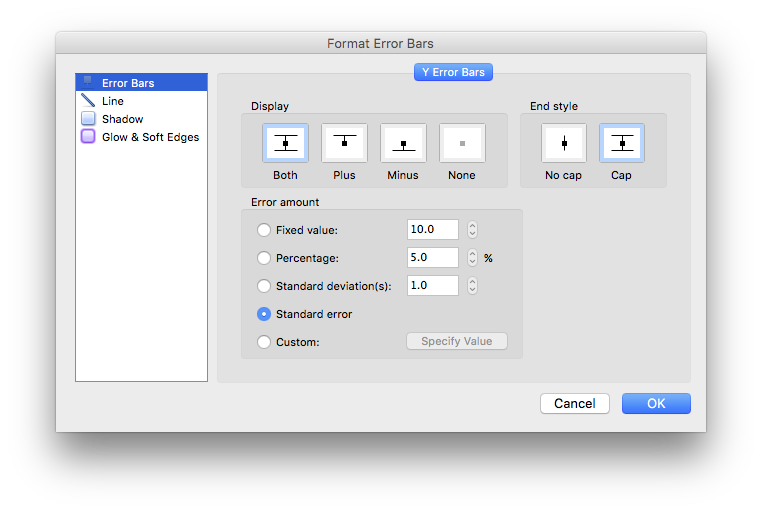The `chart_series_set_error_bars()` function sets the error bar type and value associated with the type:

"=Sheet1!\$A\$1:\$A\$5",
"=Sheet1!\$B\$1:\$B\$5");
chart_series_set_error_bars(series->y_error_bars,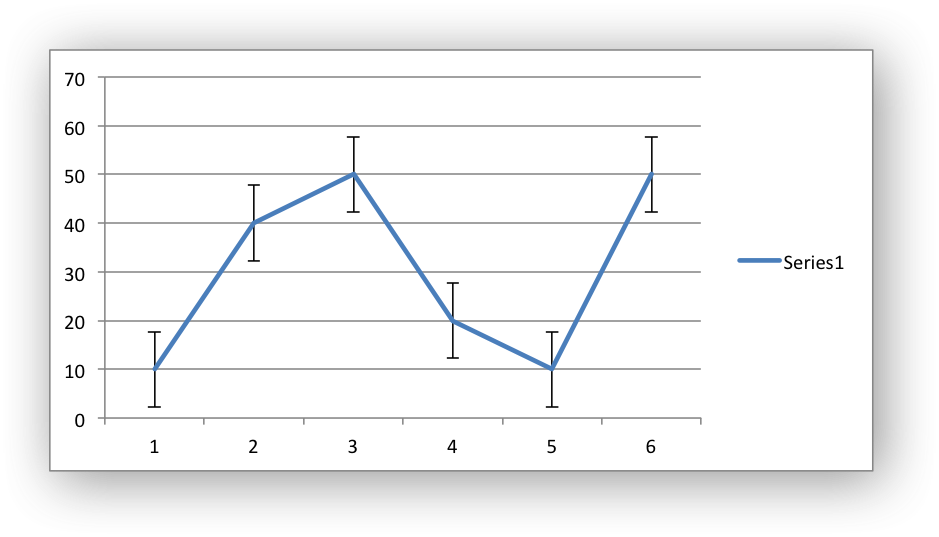The error bar types that be used are:

Note
Custom error bars are not currently supported.

All error bar types, apart from Standard error, should have a valid value to set the error range:

chart_series_set_error_bars(series1->y_error_bars,
chart_series_set_error_bars(series2->y_error_bars,
chart_series_set_error_bars(series3->y_error_bars,

For the Standard error type the value is ignored.

Examples
chart_data_tools.c.

## ◆ chart_series_set_error_bars_direction()

 void chart_series_set_error_bars_direction ( lxw_series_error_bars * error_bars, uint8_t direction )
Parameters
 error_bars A pointer to the series X or Y error bars. direction The bar direction: lxw_chart_error_bar_direction.

The `chart_series_set_error_bars_direction()` function sets the direction of the error bars:

chart_series_set_error_bars(series->y_error_bars,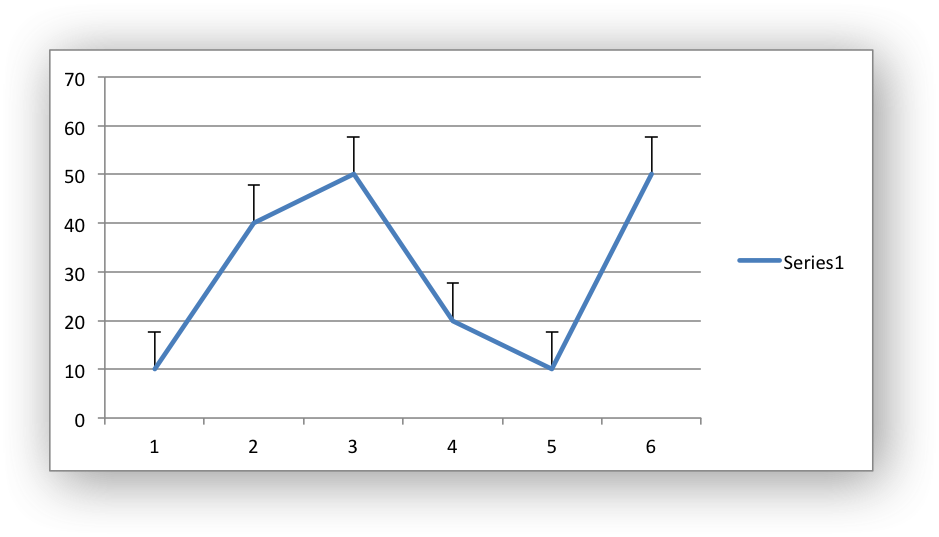The valid directions are:

## ◆ chart_series_set_error_bars_endcap()

 void chart_series_set_error_bars_endcap ( lxw_series_error_bars * error_bars, uint8_t endcap )
Parameters
 error_bars A pointer to the series X or Y error bars. endcap The error bar end cap type: lxw_chart_error_bar_cap .

The `chart_series_set_error_bars_endcap()` function sets the end cap type for the error bars:

chart_series_set_error_bars(series->y_error_bars,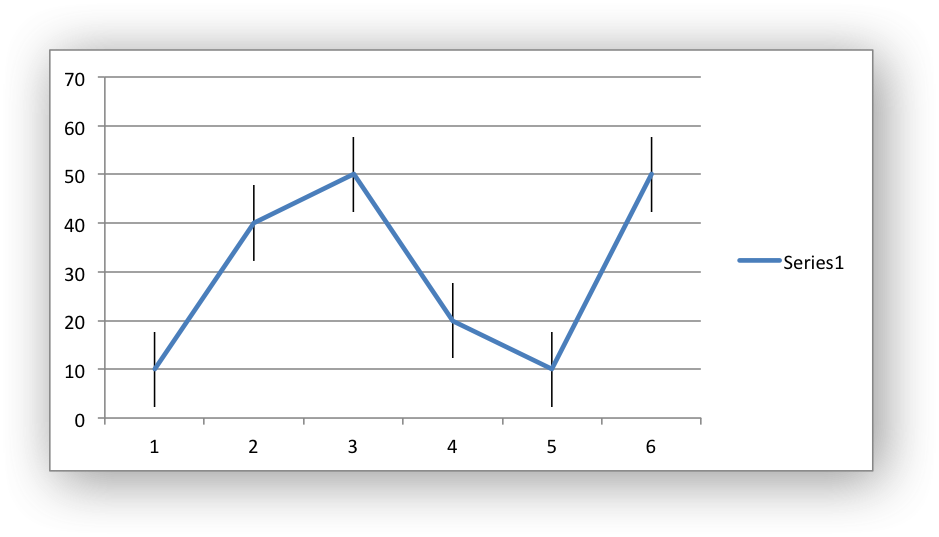The valid values are:

## ◆ chart_series_set_error_bars_line()

 void chart_series_set_error_bars_line ( lxw_series_error_bars * error_bars, lxw_chart_line * line )
Parameters
 error_bars A pointer to the series X or Y error bars. line A lxw_chart_line struct.

The `chart_series_set_error_bars_line()` function sets the line properties for the error bars:

chart_series_set_error_bars(series->y_error_bars,
chart_series_set_error_bars_line(series->y_error_bars, &line);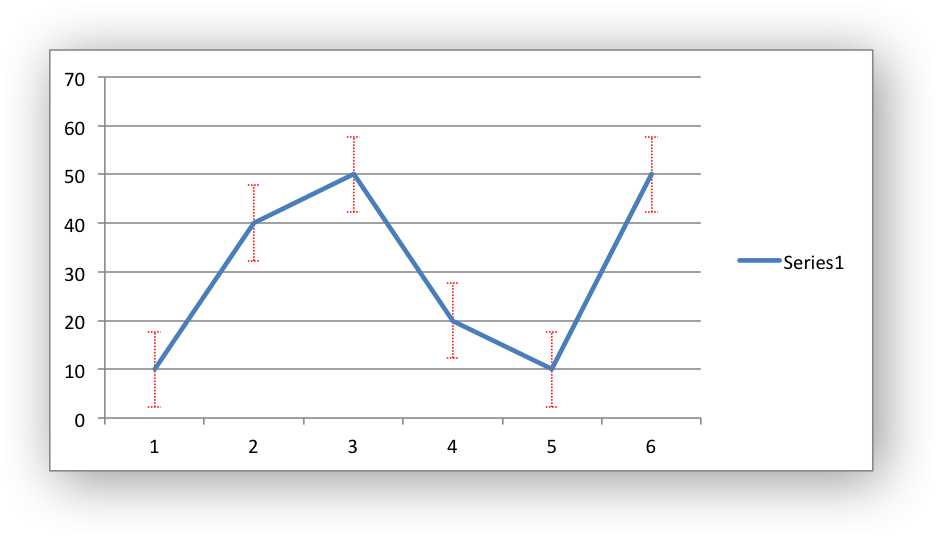For more information see Chart formatting: Line and Working with Chart Error Bars.

## ◆ chart_axis_get()

 lxw_chart_axis* chart_axis_get ( lxw_chart * chart, lxw_chart_axis_type axis_type )
Parameters
 chart Pointer to a lxw_chart instance to be configured. axis_type The axis type (X or Y): lxw_chart_axis_type.

The `chart_axis_get()` function returns a pointer to a chart axis based on the lxw_chart_axis_type:

// Use the axis pointer in other functions.

Note, the axis pointer can also be accessed directly:

// Equivalent to the above example, without function calls.
Returns

## ◆ chart_axis_set_name()

 void chart_axis_set_name ( lxw_chart_axis * axis, const char * name )
Parameters
 axis A pointer to a chart lxw_chart_axis object. name The name caption of the axis.

The `chart_axis_set_name()` function sets the name (also known as title or caption) for an axis. It can be used for the X or Y axes. The name is displayed below an X axis and to the side of a Y axis.

chart_axis_set_name(chart->x_axis, "Earnings per Quarter");
chart_axis_set_name(chart->y_axis, "US Dollars (Millions)");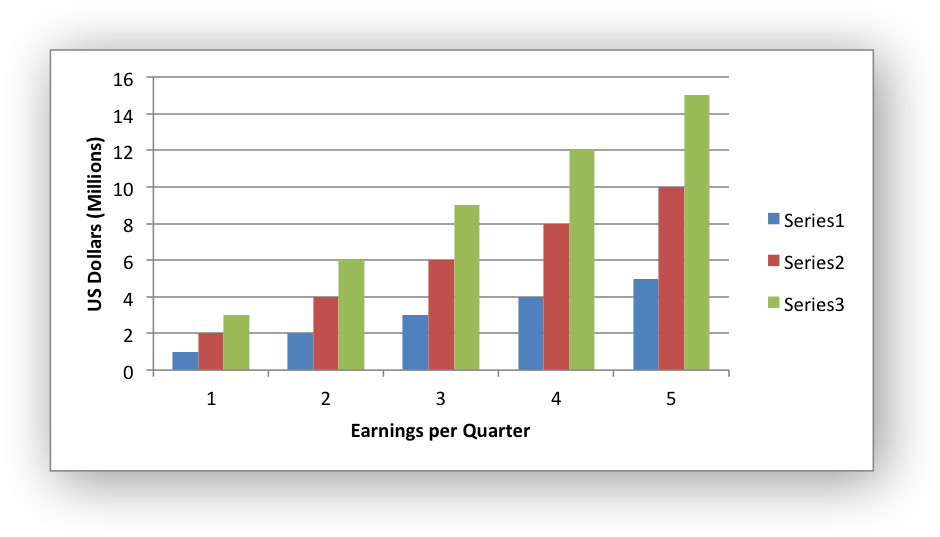The name parameter can also be a formula such as `=Sheet1!\$A\$1` to point to a cell in the workbook that contains the name:

chart_axis_set_name(chart->x_axis, "=Sheet1!\$B\$1");

See also the `chart_axis_set_name_range()` function to see how to set the name formula programmatically.

Axis types: This function is applicable to to all axes types. See Chart Value and Category Axes.

Examples
chart_area.c, chart_bar.c, chart_column.c, chart_data_table.c, chart_fonts.c, chart_line.c, chart_pattern.c, chart_radar.c, chart_scatter.c, and chartsheet.c.

## ◆ chart_axis_set_name_range()

 void chart_axis_set_name_range ( lxw_chart_axis * axis, const char * sheetname, lxw_row_t row, lxw_col_t col )
Parameters
 axis A pointer to a chart lxw_chart_axis object. sheetname The name of the worksheet that contains the cell range. row The zero indexed row number of the range. col The zero indexed column number of the range.

The `chart_axis_set_name_range()` function can be used to set an axis name range and is an alternative to using `chart_axis_set_name()` and a string formula:

chart_axis_set_name_range(chart->x_axis, "Sheet1", 1, 0);
chart_axis_set_name_range(chart->y_axis, "Sheet1", 2, 0);

Axis types: This function is applicable to to all axes types. See Chart Value and Category Axes.

## ◆ chart_axis_set_name_font()

 void chart_axis_set_name_font ( lxw_chart_axis * axis, lxw_chart_font * font )
Parameters
 axis A pointer to a chart lxw_chart_axis object. font A pointer to a chart lxw_chart_font font struct.

The `chart_axis_set_name_font()` function is used to set the font of an axis name:

chart_axis_set_name(chart->x_axis, "Yearly data");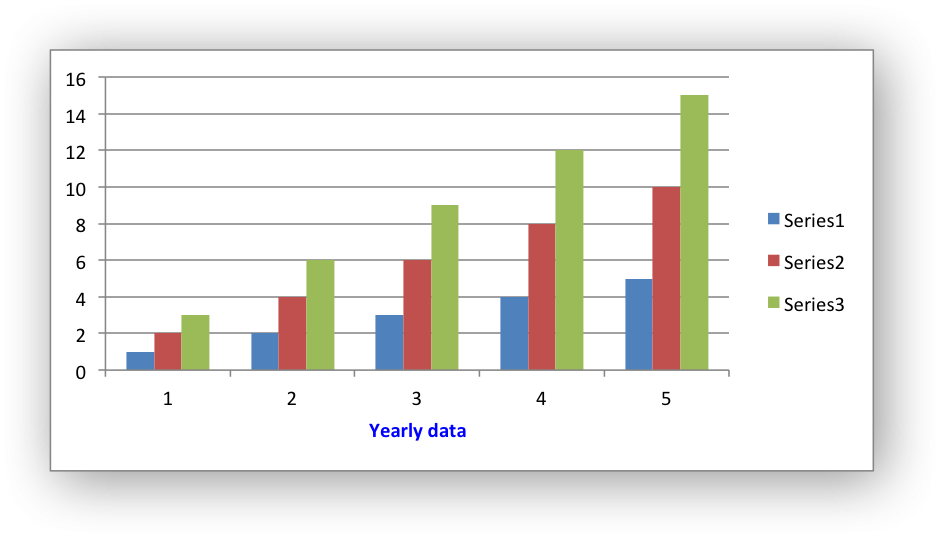Axis types: This function is applicable to to all axes types. See Chart Value and Category Axes.

Examples
chart_fonts.c.

## ◆ chart_axis_set_num_font()

 void chart_axis_set_num_font ( lxw_chart_axis * axis, lxw_chart_font * font )
Parameters
 axis A pointer to a chart lxw_chart_axis object. font A pointer to a chart lxw_chart_font font struct.

The `chart_axis_set_num_font()` function is used to set the font of the numbers on an axis: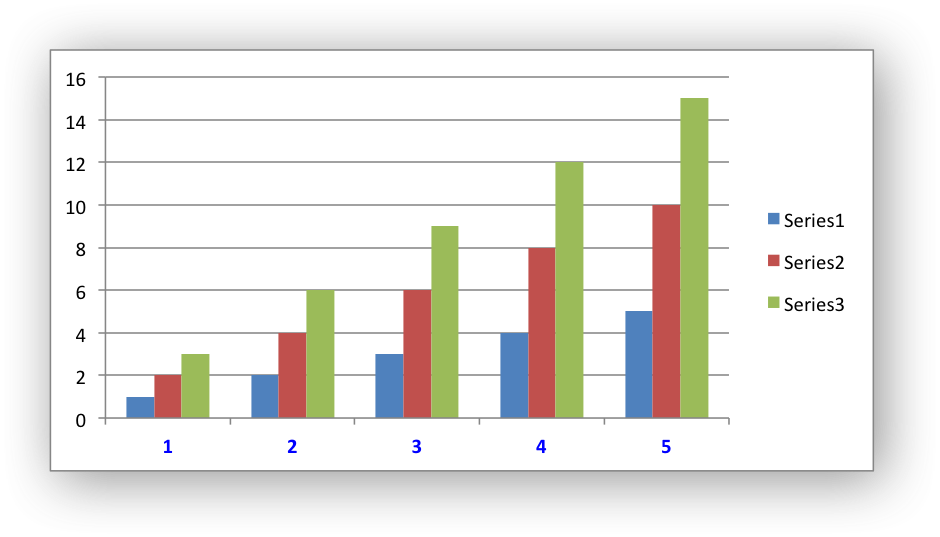Axis types: This function is applicable to to all axes types. See Chart Value and Category Axes.

Examples
chart_fonts.c.

## ◆ chart_axis_set_num_format()

 void chart_axis_set_num_format ( lxw_chart_axis * axis, const char * num_format )
Parameters
 axis A pointer to a chart lxw_chart_axis object. num_format The number format string.

The `chart_axis_set_num_format()` function is used to set the format of the numbers on an axis:

chart_axis_set_num_format(chart->y_axis, "\$#,##0.00");

The number format is similar to the Worksheet Cell Format num_format, see `format_set_num_format()`.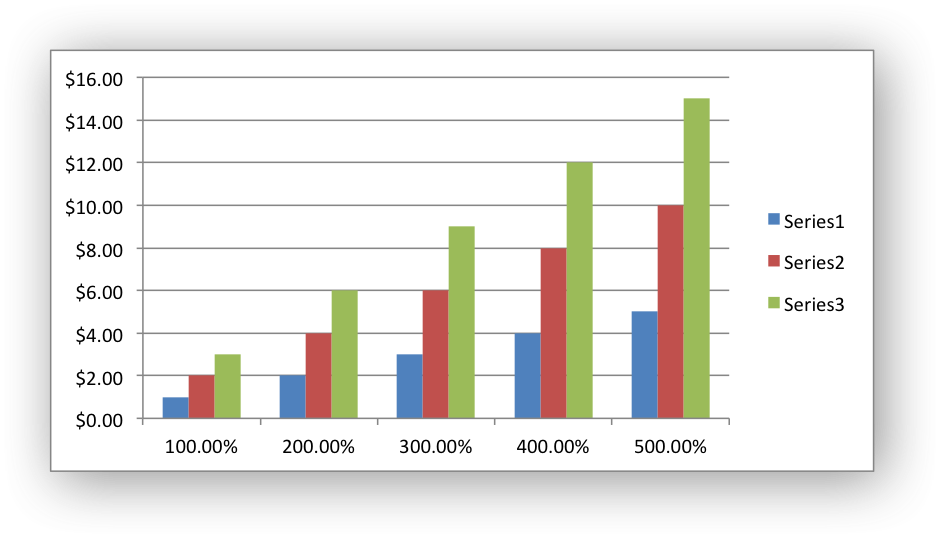Axis types: This function is applicable to to all axes types. See Chart Value and Category Axes.

## ◆ chart_axis_set_line()

 void chart_axis_set_line ( lxw_chart_axis * axis, lxw_chart_line * line )
Parameters
 axis A pointer to a chart lxw_chart_axis object. line A lxw_chart_line struct.

Set the line properties of a chart axis:

// Hide the Y axis.
chart_axis_set_line(chart->y_axis, &line);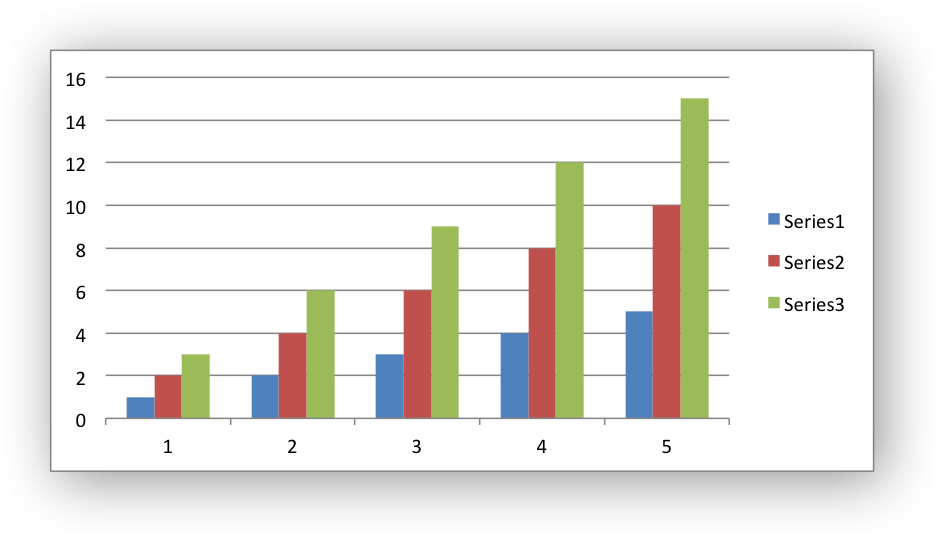Axis types: This function is applicable to to all axes types. See Chart Value and Category Axes.

## ◆ chart_axis_set_fill()

 void chart_axis_set_fill ( lxw_chart_axis * axis, lxw_chart_fill * fill )
Parameters
 axis A pointer to a chart lxw_chart_axis object. fill A lxw_chart_fill struct.

Set the fill properties of a chart axis: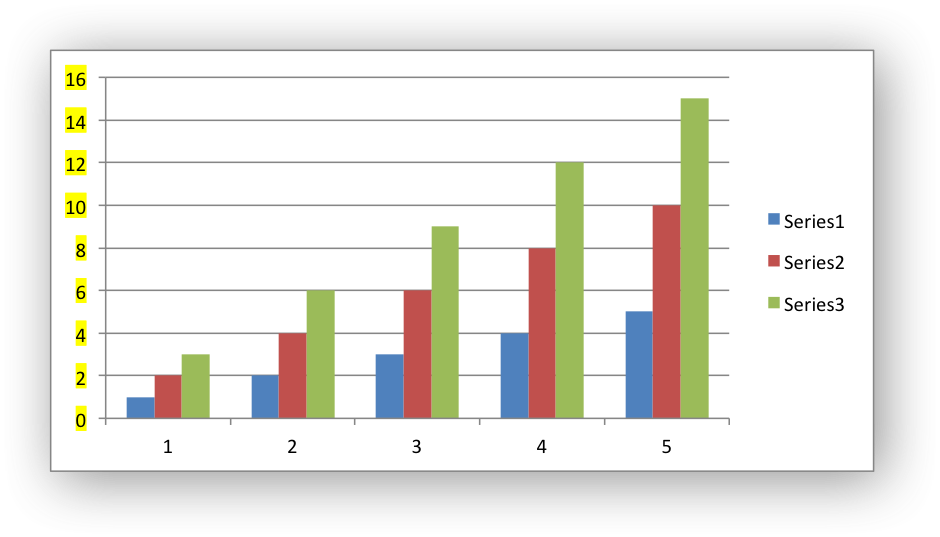Axis types: This function is applicable to to all axes types. See Chart Value and Category Axes.

## ◆ chart_axis_set_pattern()

 void chart_axis_set_pattern ( lxw_chart_axis * axis, lxw_chart_pattern * pattern )
Parameters
 axis A pointer to a chart lxw_chart_axis object. pattern A lxw_chart_pattern struct.

Set the pattern properties of a chart axis:

chart_axis_set_pattern(chart->y_axis, &pattern);

Axis types: This function is applicable to to all axes types. See Chart Value and Category Axes.

## ◆ chart_axis_set_reverse()

 void chart_axis_set_reverse ( lxw_chart_axis * axis )
Parameters
 axis A pointer to a chart lxw_chart_axis object.

Reverse the order of the axis categories or values: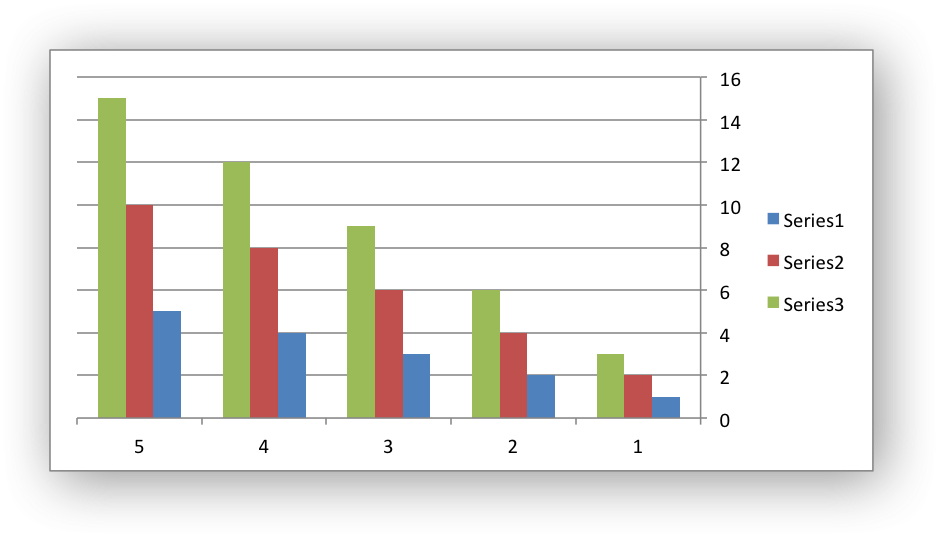Axis types: This function is applicable to to all axes types. See Chart Value and Category Axes.

## ◆ chart_axis_set_crossing()

 void chart_axis_set_crossing ( lxw_chart_axis * axis, double value )
Parameters
 axis A pointer to a chart lxw_chart_axis object. value The category or value that the axis crosses at.

Set the position that the axis will cross the opposite axis: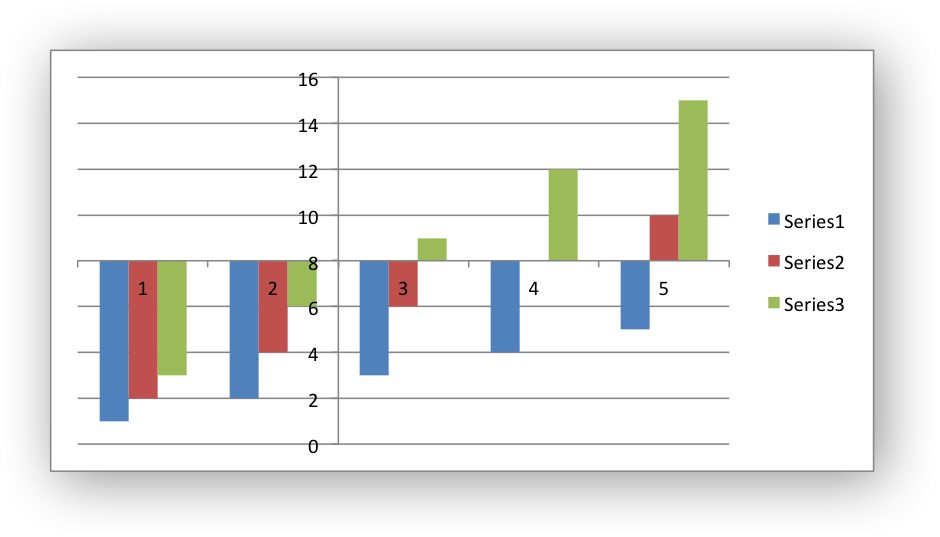If crossing is omitted (the default) the crossing will be set automatically by Excel based on the chart data.

Axis types: This function is applicable to to all axes types. See Chart Value and Category Axes.

## ◆ chart_axis_set_crossing_max()

 void chart_axis_set_crossing_max ( lxw_chart_axis * axis )
Parameters
 axis A pointer to a chart lxw_chart_axis object.

Set the position that the opposite axis will cross as the axis maximum. The default axis crossing position is generally the axis minimum so this function can be used to reverse the location of the axes without reversing the number sequence: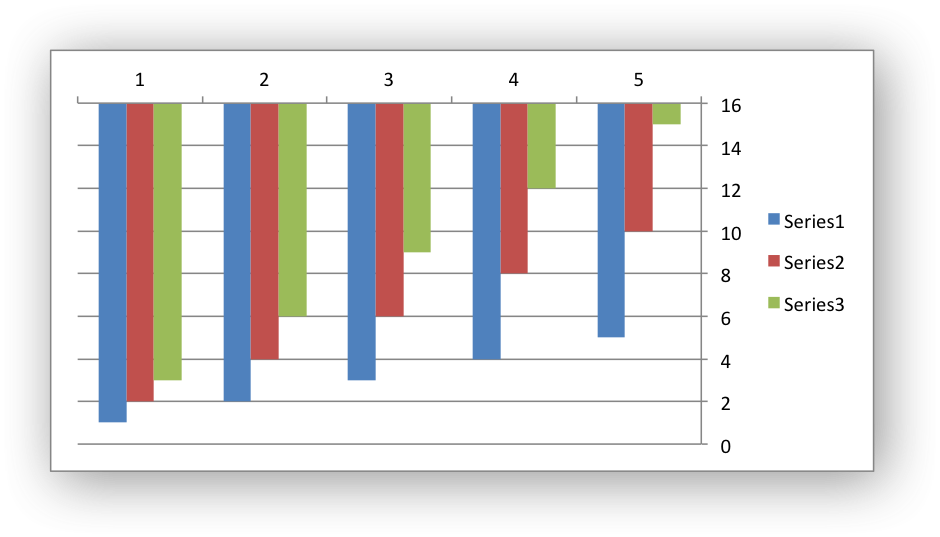Axis types: This function is applicable to to all axes types. See Chart Value and Category Axes.

## ◆ chart_axis_set_crossing_min()

 void chart_axis_set_crossing_min ( lxw_chart_axis * axis )
Parameters
 axis A pointer to a chart lxw_chart_axis object.

Set the position that the opposite axis will cross as the axis minimum. The default axis crossing position is generally the axis minimum so this function can be used to reverse the location of the axes without reversing the number sequence:

Axis types: This function is applicable to to all axes types. See Chart Value and Category Axes.

## ◆ chart_axis_off()

 void chart_axis_off ( lxw_chart_axis * axis )
Parameters
 axis A pointer to a chart lxw_chart_axis object.

Turn off, hide, a chart axis: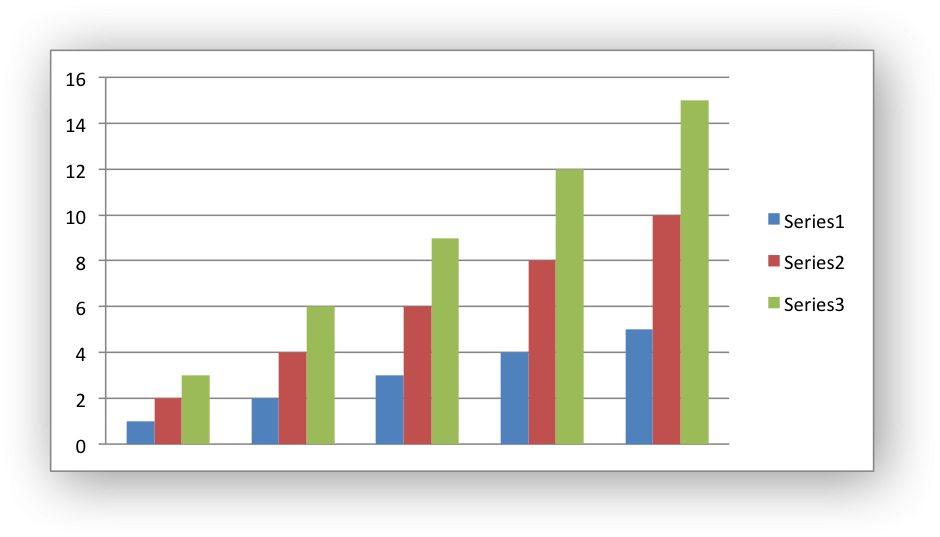Axis types: This function is applicable to to all axes types. See Chart Value and Category Axes.

## ◆ chart_axis_set_position()

 void chart_axis_set_position ( lxw_chart_axis * axis, uint8_t position )
Parameters
 axis A pointer to a chart lxw_chart_axis object. position A lxw_chart_axis_tick_position value.

Position a category axis horizontally on, or between, the axis tick marks.

There are two allowable values: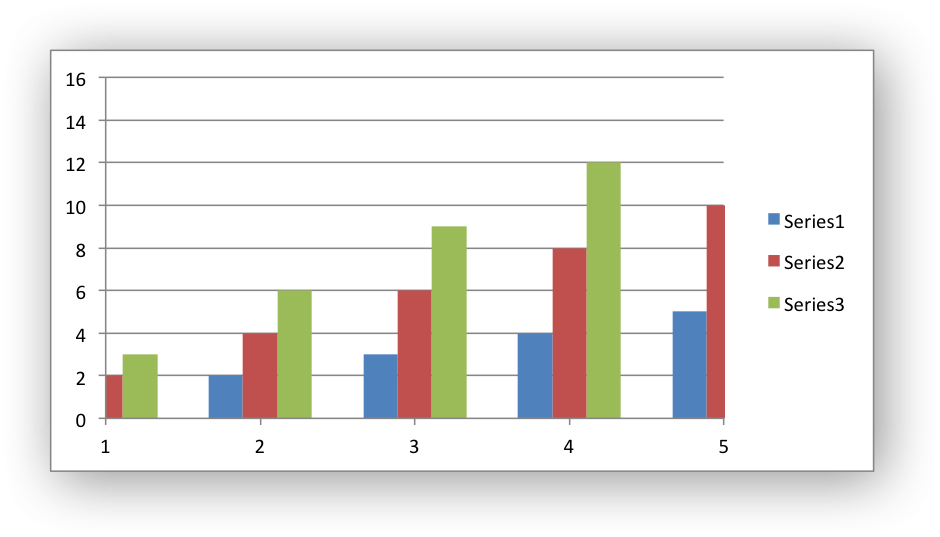Axis types: This function is applicable to category axes only. See Chart Value and Category Axes.

## ◆ chart_axis_set_label_position()

 void chart_axis_set_label_position ( lxw_chart_axis * axis, uint8_t position )
Parameters
 axis A pointer to a chart lxw_chart_axis object. position A lxw_chart_axis_label_position value.

Position the axis labels for the chart. The labels are the numbers, or strings or dates, on the axis that indicate the categories or values of the axis.

For example: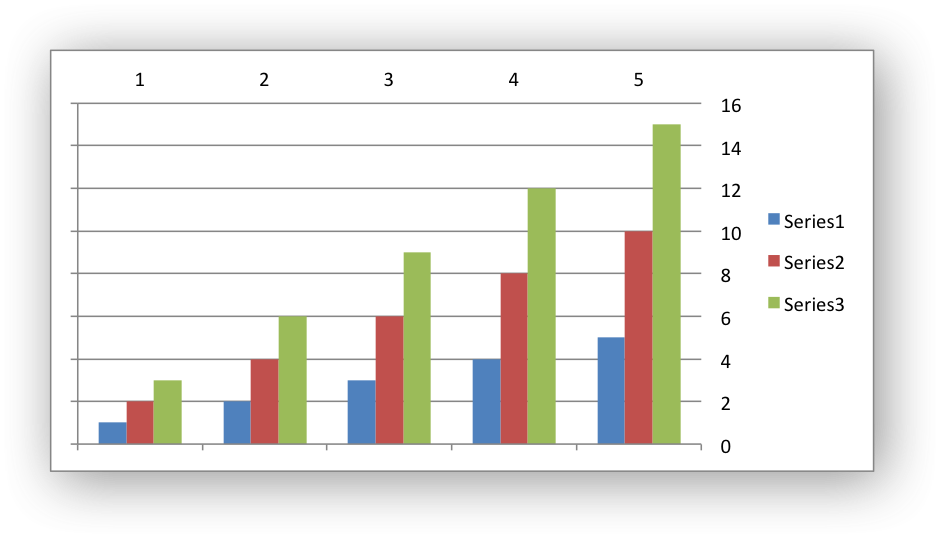The allowable values: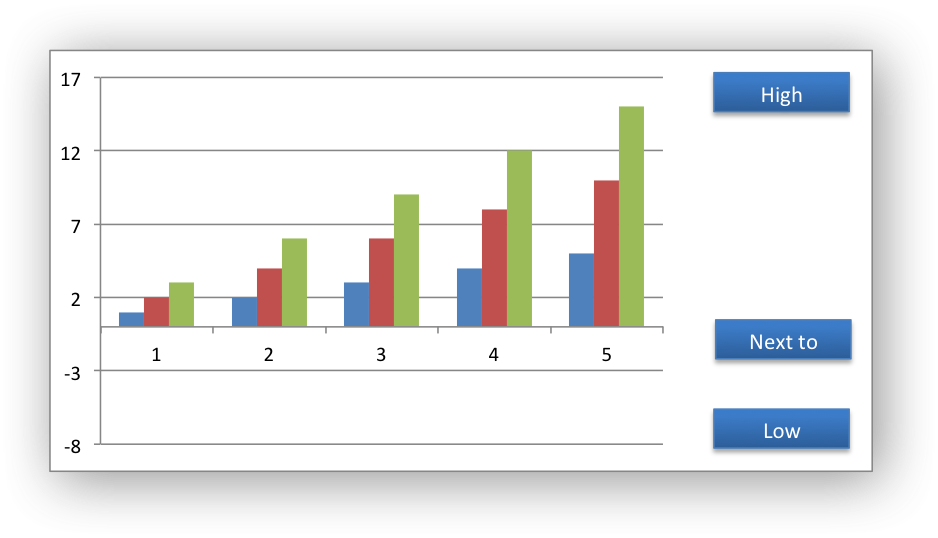The LXW_CHART_AXIS_LABEL_POSITION_NONE turns off the axis labels. This is slightly different from `chart_axis_off()` which also turns off the labels but also turns off tick marks.

Axis types: This function is applicable to to all axes types. See Chart Value and Category Axes.

## ◆ chart_axis_set_label_align()

 void chart_axis_set_label_align ( lxw_chart_axis * axis, uint8_t align )
Parameters
 axis A pointer to a chart lxw_chart_axis object. align A lxw_chart_axis_label_alignment value.

Position the category axis labels for the chart. The labels are the numbers, or strings or dates, on the axis that indicate the categories of the axis.

The allowable values:

Axis types: This function is applicable to category axes only. See Chart Value and Category Axes.

## ◆ chart_axis_set_min()

 void chart_axis_set_min ( lxw_chart_axis * axis, double min )
Parameters
 axis A pointer to a chart lxw_chart_axis object. min Minimum value for chart axis. Value axes only.

Set the minimum value for the axis range.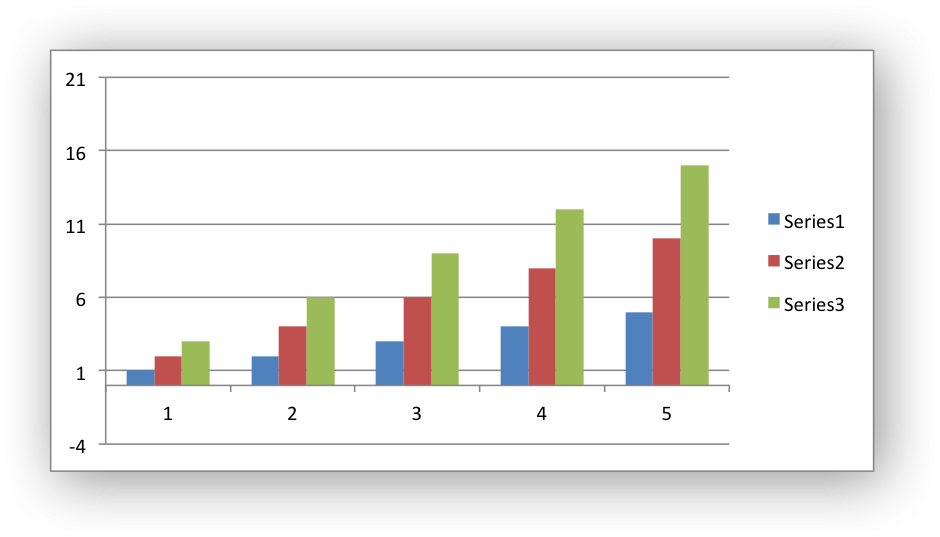Axis types: This function is applicable to value and date axes only. See Chart Value and Category Axes.

## ◆ chart_axis_set_max()

 void chart_axis_set_max ( lxw_chart_axis * axis, double max )
Parameters
 axis A pointer to a chart lxw_chart_axis object. max Maximum value for chart axis. Value axes only.

Set the maximum value for the axis range.

See the above image.

Axis types: This function is applicable to value and date axes only. See Chart Value and Category Axes.

## ◆ chart_axis_set_log_base()

 void chart_axis_set_log_base ( lxw_chart_axis * axis, uint16_t log_base )
Parameters
 axis A pointer to a chart lxw_chart_axis object. log_base The log base for value axis. Value axes only.

Set the log base for the axis: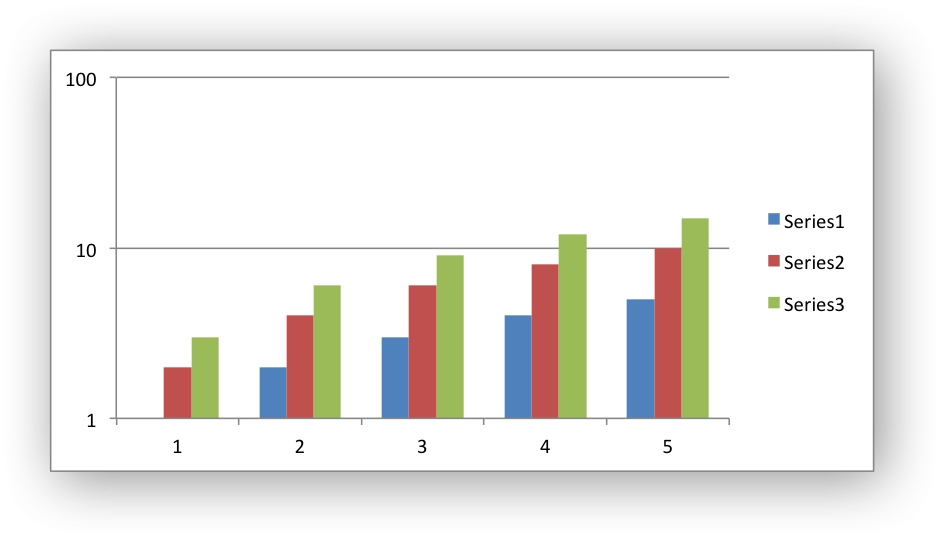The allowable range of values for the log base in Excel is between 2 and 1000.

Axis types: This function is applicable to value axes only. See Chart Value and Category Axes.

## ◆ chart_axis_set_major_tick_mark()

 void chart_axis_set_major_tick_mark ( lxw_chart_axis * axis, uint8_t type )
Parameters
 axis A pointer to a chart lxw_chart_axis object. type The tick mark type, defined by lxw_chart_tick_mark.

Set the type of the major axis tick mark:

// Hide the default gridlines so the tick marks are visible.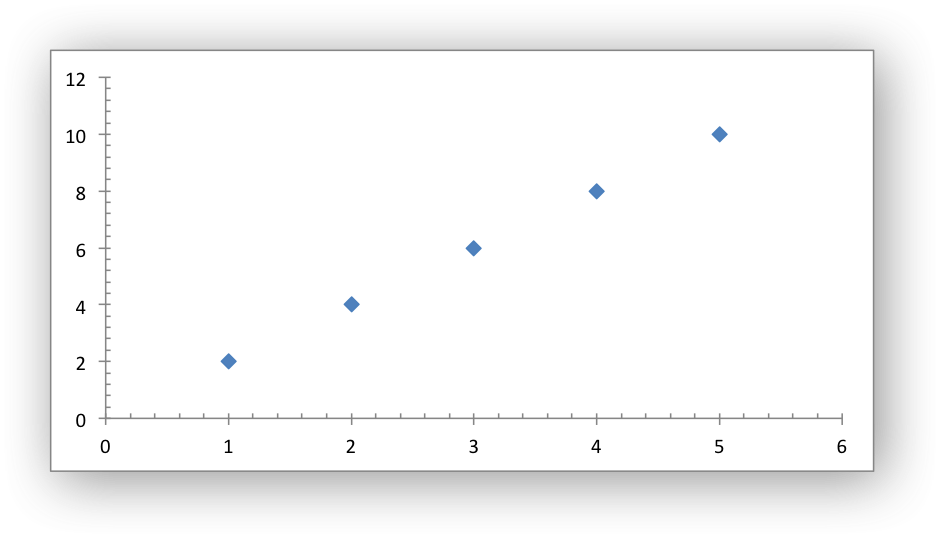The tick mark types are:

Axis types: This function is applicable to to all axes types. See Chart Value and Category Axes.

## ◆ chart_axis_set_minor_tick_mark()

 void chart_axis_set_minor_tick_mark ( lxw_chart_axis * axis, uint8_t type )
Parameters
 axis A pointer to a chart lxw_chart_axis object. type The tick mark type, defined by lxw_chart_tick_mark.

Set the type of the minor axis tick mark:

See the image and example above.

Axis types: This function is applicable to to all axes types. See Chart Value and Category Axes.

## ◆ chart_axis_set_interval_unit()

 void chart_axis_set_interval_unit ( lxw_chart_axis * axis, uint16_t unit )
Parameters
 axis A pointer to a chart lxw_chart_axis object. unit The interval between the categories.

Set the interval between the category values. The default interval is 1 which gives the intervals shown in the charts above:

```1, 2, 3, 4, 5, etc.
```

Setting it to 2 gives:

```1, 3, 5, 7, etc.
```

For example: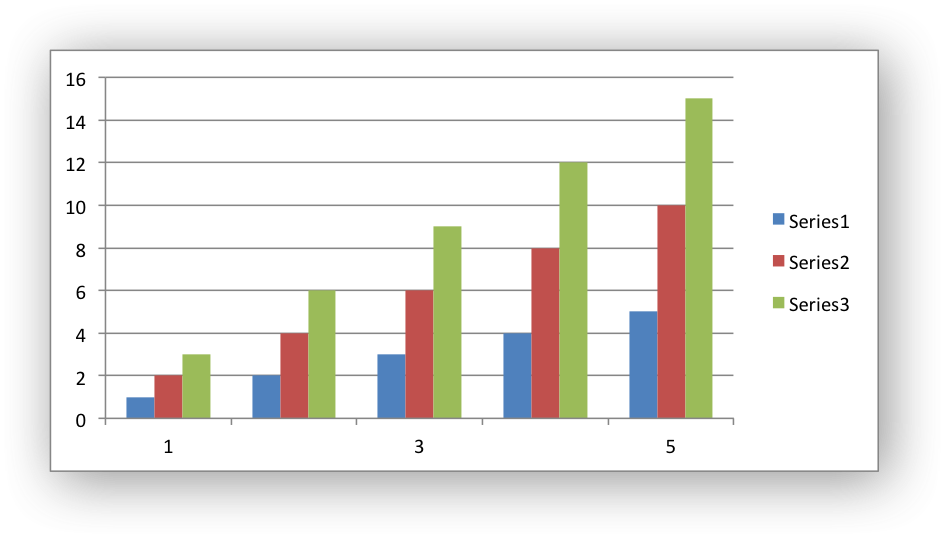Axis types: This function is applicable to category and date axes only. See Chart Value and Category Axes.

## ◆ chart_axis_set_interval_tick()

 void chart_axis_set_interval_tick ( lxw_chart_axis * axis, uint16_t unit )
Parameters
 axis A pointer to a chart lxw_chart_axis object. unit The interval between the category ticks.

Set the interval between the category tick marks. The default interval is 1 between each category but it can be set to other integer values: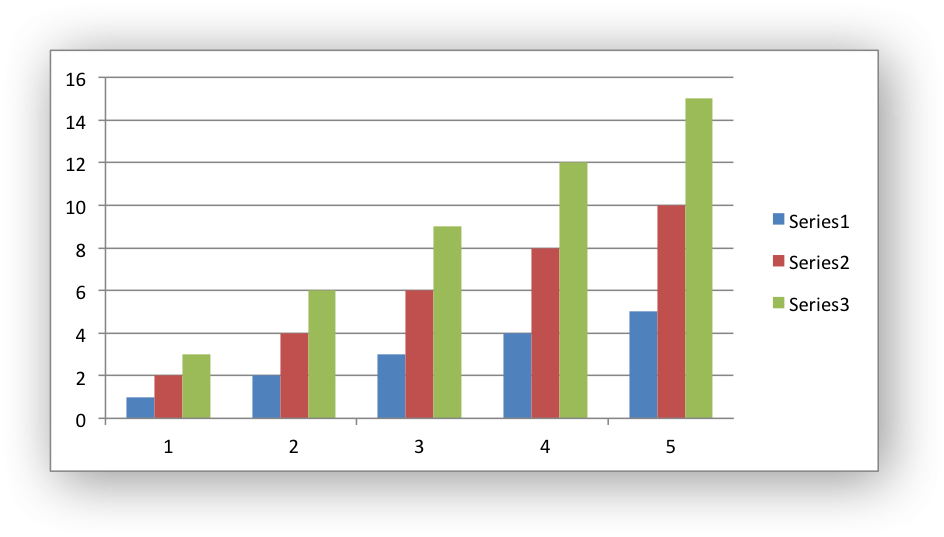Axis types: This function is applicable to category and date axes only. See Chart Value and Category Axes.

## ◆ chart_axis_set_major_unit()

 void chart_axis_set_major_unit ( lxw_chart_axis * axis, double unit )
Parameters
 axis A pointer to a chart lxw_chart_axis object. unit The increment of the major units.

Set the increment of the major units in the axis range.

// Turn on the minor gridline (it is off by default).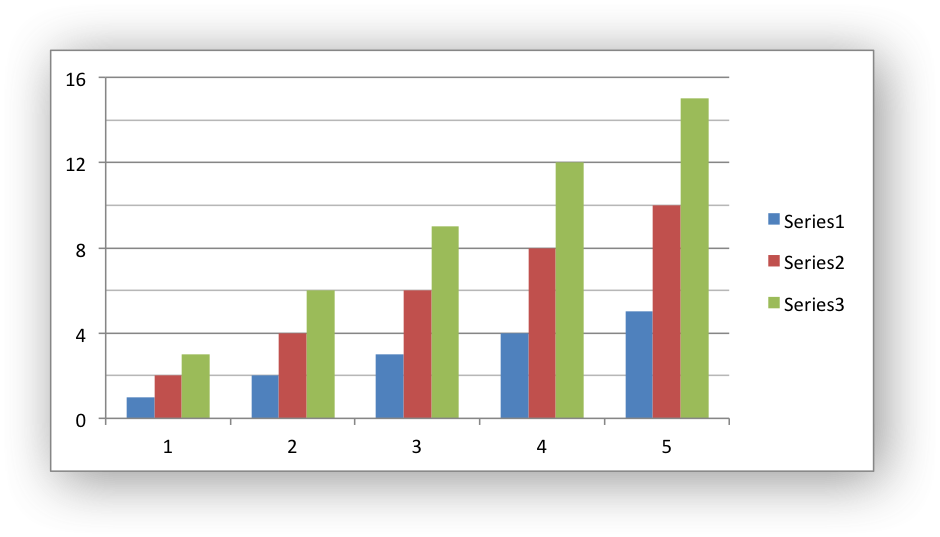Axis types: This function is applicable to value and date axes only. See Chart Value and Category Axes.

## ◆ chart_axis_set_minor_unit()

 void chart_axis_set_minor_unit ( lxw_chart_axis * axis, double unit )
Parameters
 axis A pointer to a chart lxw_chart_axis object. unit The increment of the minor units.

Set the increment of the minor units in the axis range.

See the image above

Axis types: This function is applicable to value and date axes only. See Chart Value and Category Axes.

## ◆ chart_axis_set_display_units()

 void chart_axis_set_display_units ( lxw_chart_axis * axis, uint8_t units )
Parameters
 axis A pointer to a chart lxw_chart_axis object. units The display units: lxw_chart_axis_display_unit.

Set the display units for the axis. This can be useful if the axis numbers are very large but you don't want to represent them in scientific notation: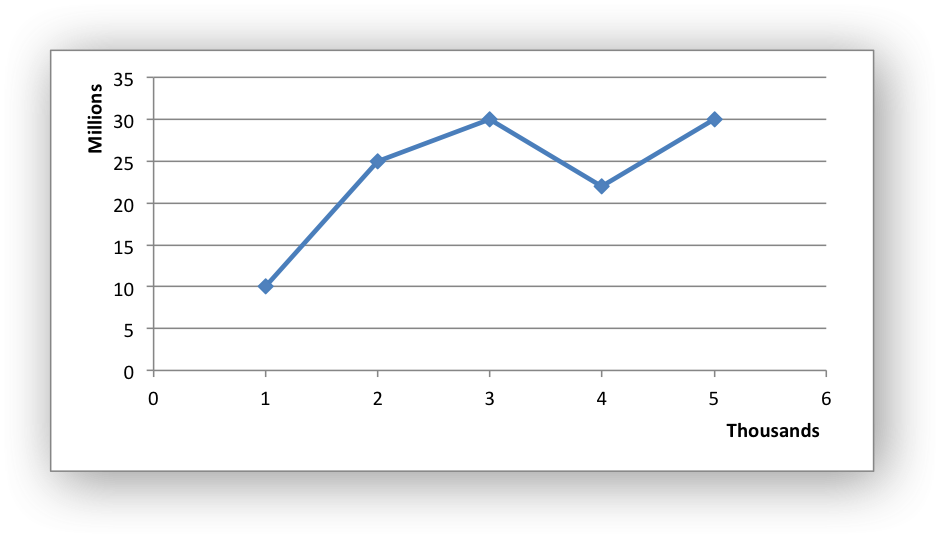Axis types: This function is applicable to value axes only. See Chart Value and Category Axes.

## ◆ chart_axis_set_display_units_visible()

 void chart_axis_set_display_units_visible ( lxw_chart_axis * axis, uint8_t visible )
Parameters
 axis A pointer to a chart lxw_chart_axis object. visible Turn off/on the display units. (0/1)

Turn on or off the display units for the axis. This option is set on automatically by `chart_axis_set_display_units()`.

Axis types: This function is applicable to value axes only. See Chart Value and Category Axes.

## ◆ chart_axis_major_gridlines_set_visible()

 void chart_axis_major_gridlines_set_visible ( lxw_chart_axis * axis, uint8_t visible )
Parameters
 axis A pointer to a chart lxw_chart_axis object. visible Turn off/on the major gridline. (0/1)

Turn on or off the major gridlines for an X or Y axis. In most Excel charts the Y axis major gridlines are on by default and the X axis major gridlines are off by default.

Example:

// Reverse the normal visible/hidden gridlines for a column chart.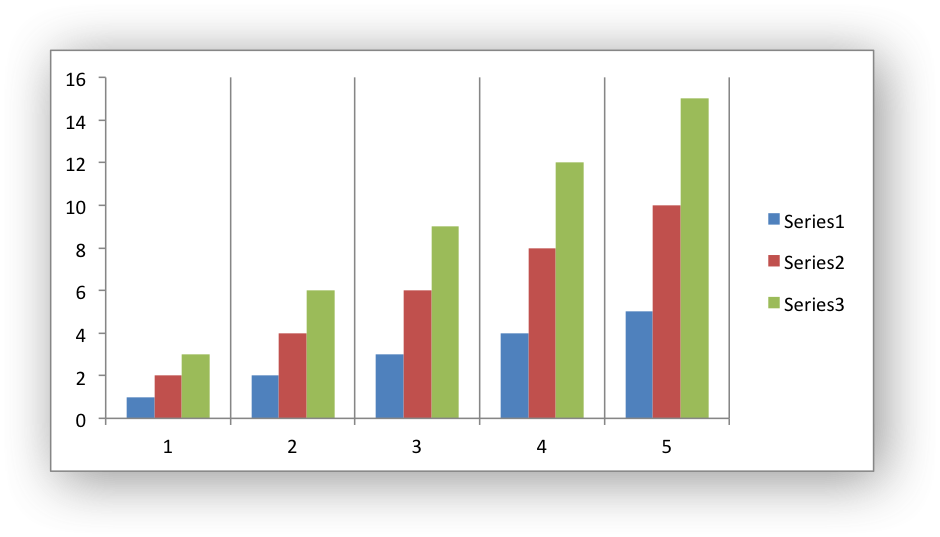Axis types: This function is applicable to to all axes types. See Chart Value and Category Axes.

## ◆ chart_axis_minor_gridlines_set_visible()

 void chart_axis_minor_gridlines_set_visible ( lxw_chart_axis * axis, uint8_t visible )
Parameters
 axis A pointer to a chart lxw_chart_axis object. visible Turn off/on the minor gridline. (0/1)

Turn on or off the minor gridlines for an X or Y axis. In most Excel charts the X and Y axis minor gridlines are off by default.

Example, turn on all major and minor gridlines: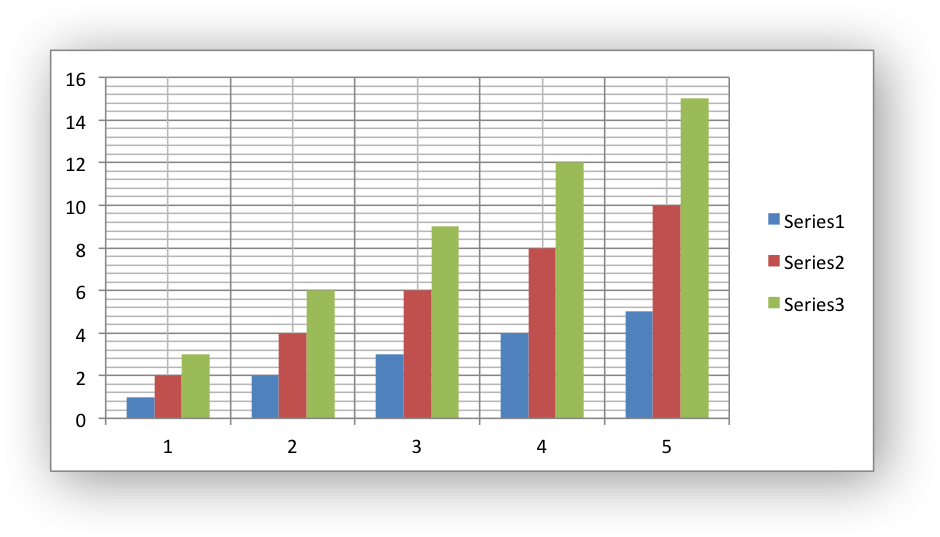Axis types: This function is applicable to to all axes types. See Chart Value and Category Axes.

## ◆ chart_axis_major_gridlines_set_line()

 void chart_axis_major_gridlines_set_line ( lxw_chart_axis * axis, lxw_chart_line * line )
Parameters
 axis A pointer to a chart lxw_chart_axis object. line A lxw_chart_line struct.

Format the line properties of the major gridlines of a chart: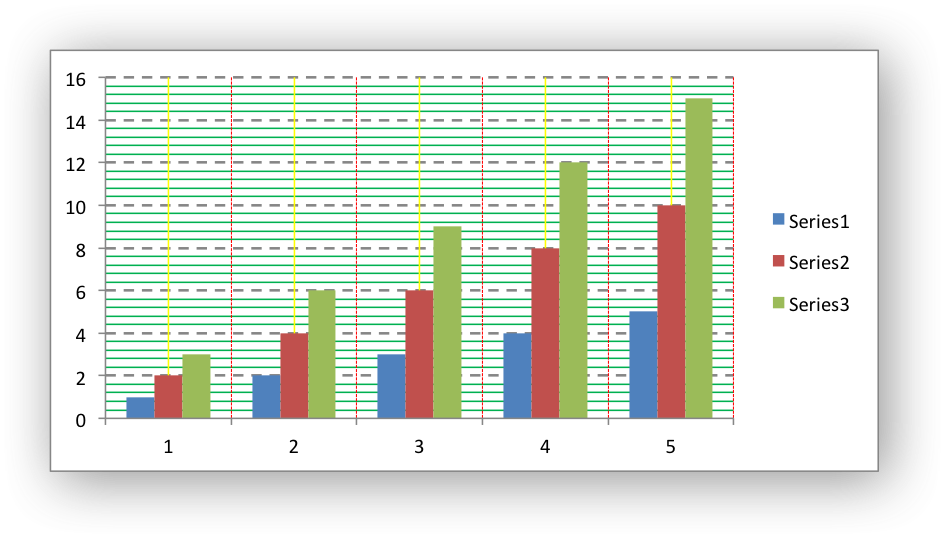Axis types: This function is applicable to to all axes types. See Chart Value and Category Axes.

## ◆ chart_axis_minor_gridlines_set_line()

 void chart_axis_minor_gridlines_set_line ( lxw_chart_axis * axis, lxw_chart_line * line )
Parameters
 axis A pointer to a chart lxw_chart_axis object. line A lxw_chart_line struct.

Format the line properties of the minor gridlines of a chart, see the example above.

Axis types: This function is applicable to to all axes types. See Chart Value and Category Axes.

## ◆ chart_title_set_name()

 void chart_title_set_name ( lxw_chart * chart, const char * name )
Parameters
 chart Pointer to a lxw_chart instance to be configured. name The chart title name.

The `chart_title_set_name()` function sets the name (title) for the chart. The name is displayed above the chart.

chart_title_set_name(chart, "Year End Results");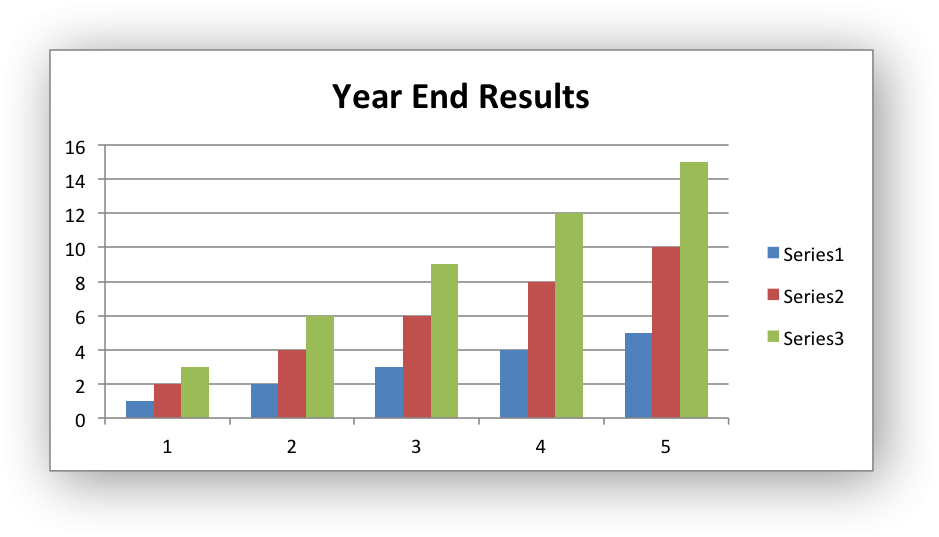The name parameter can also be a formula such as `=Sheet1!\$A\$1` to point to a cell in the workbook that contains the name:

chart_title_set_name(chart, "=Sheet1!\$B\$1");

See also the `chart_title_set_name_range()` function to see how to set the name formula programmatically.

The Excel default is to have no chart title.

Examples
chart.c, chart_area.c, chart_bar.c, chart_column.c, chart_data_labels.c, chart_data_table.c, chart_data_tools.c, chart_doughnut.c, chart_fonts.c, chart_line.c, chart_pattern.c, chart_pie.c, chart_radar.c, chart_scatter.c, chart_styles.c, and chartsheet.c.

## ◆ chart_title_set_name_range()

 void chart_title_set_name_range ( lxw_chart * chart, const char * sheetname, lxw_row_t row, lxw_col_t col )
Parameters
 chart Pointer to a lxw_chart instance to be configured. sheetname The name of the worksheet that contains the cell range. row The zero indexed row number of the range. col The zero indexed column number of the range.

The `chart_title_set_name_range()` function can be used to set a chart title range and is an alternative to using `chart_title_set_name()` and a string formula:

chart_title_set_name_range(chart, "Sheet1", 1, 0);

## ◆ chart_title_set_name_font()

 void chart_title_set_name_font ( lxw_chart * chart, lxw_chart_font * font )
Parameters
 chart Pointer to a lxw_chart instance to be configured. font A pointer to a chart lxw_chart_font font struct.

The `chart_title_set_name_font()` function is used to set the font of a chart title:

chart_title_set_name(chart, "Year End Results");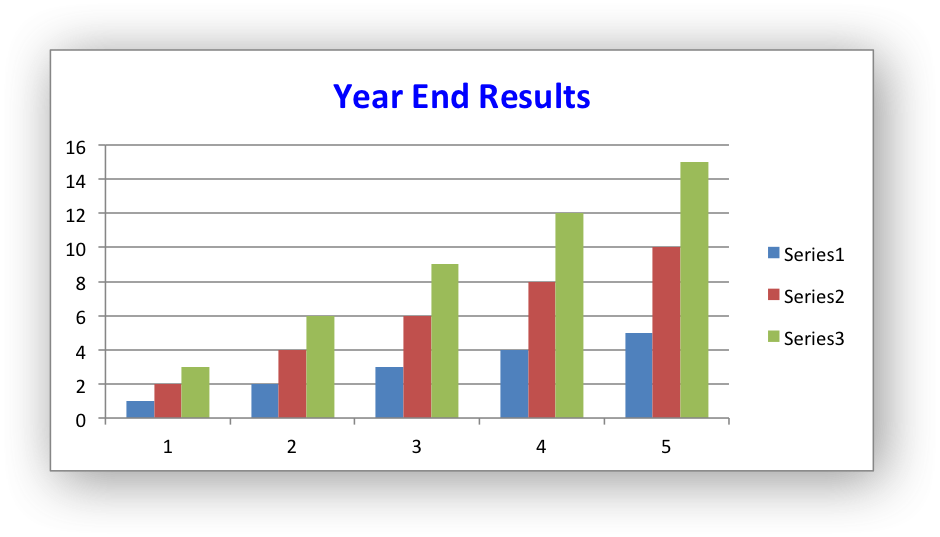In Excel a chart title font is bold by default (as shown in the image above). To turn off bold in the font you cannot use LXW_FALSE (0) since that is indistinguishable from an uninitialized value. Instead you should use LXW_EXPLICIT_FALSE:

chart_title_set_name(chart, "Year End Results");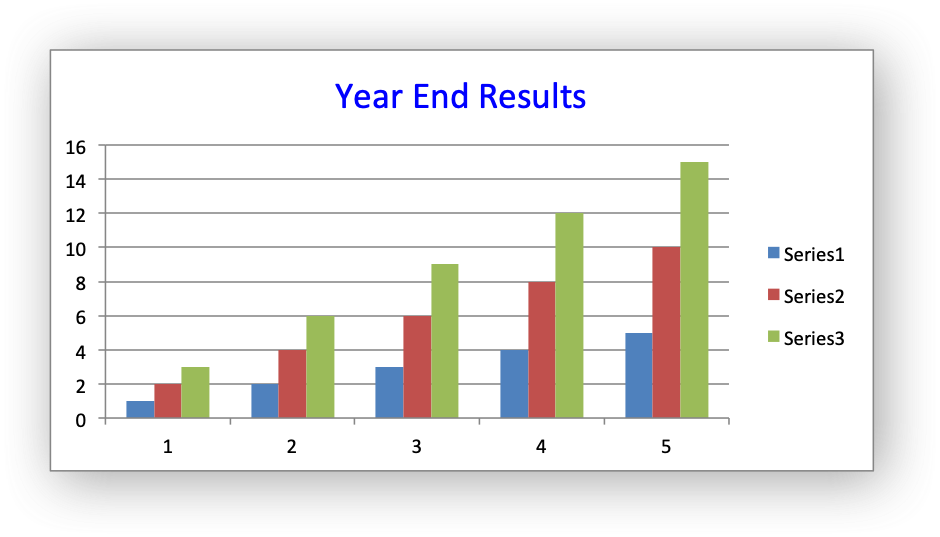Examples
chart.c, and chart_fonts.c.

## ◆ chart_title_off()

 void chart_title_off ( lxw_chart * chart )
Parameters
 chart Pointer to a lxw_chart instance to be configured.

In general in Excel a chart title isn't displayed unless the user explicitly adds one. However, Excel adds an automatic chart title to charts with a single series and a user defined series name. The `chart_title_off()` function allows you to turn off this automatic chart title:

## ◆ chart_legend_set_position()

 void chart_legend_set_position ( lxw_chart * chart, uint8_t position )
Parameters
 chart Pointer to a lxw_chart instance to be configured. position The lxw_chart_legend_position value for the legend.

The `chart_legend_set_position()` function is used to set the chart legend to one of the lxw_chart_legend_position values:

```LXW_CHART_LEGEND_NONE
LXW_CHART_LEGEND_RIGHT
LXW_CHART_LEGEND_LEFT
LXW_CHART_LEGEND_TOP
LXW_CHART_LEGEND_BOTTOM
LXW_CHART_LEGEND_TOP_RIGHT
LXW_CHART_LEGEND_OVERLAY_RIGHT
LXW_CHART_LEGEND_OVERLAY_LEFT
LXW_CHART_LEGEND_OVERLAY_TOP_RIGHT
```

For example: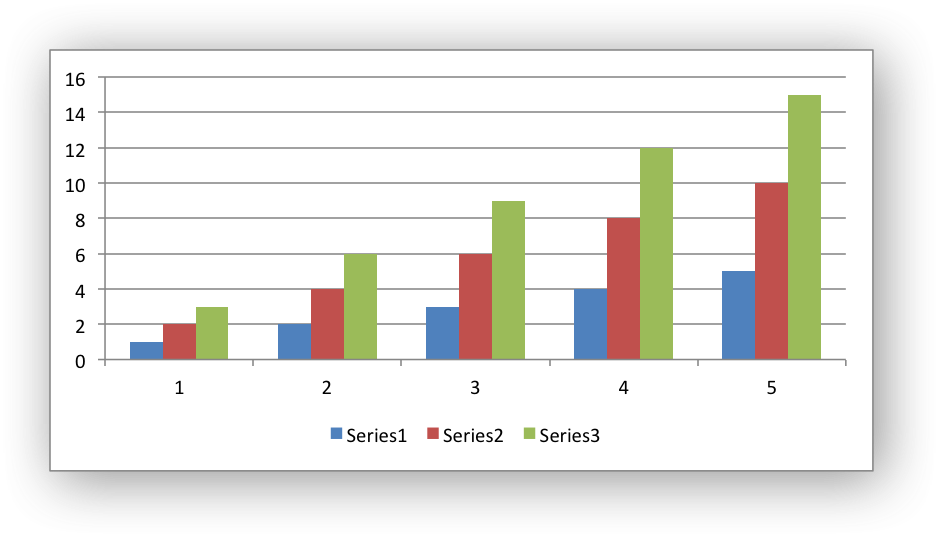This function can also be used to turn off a chart legend: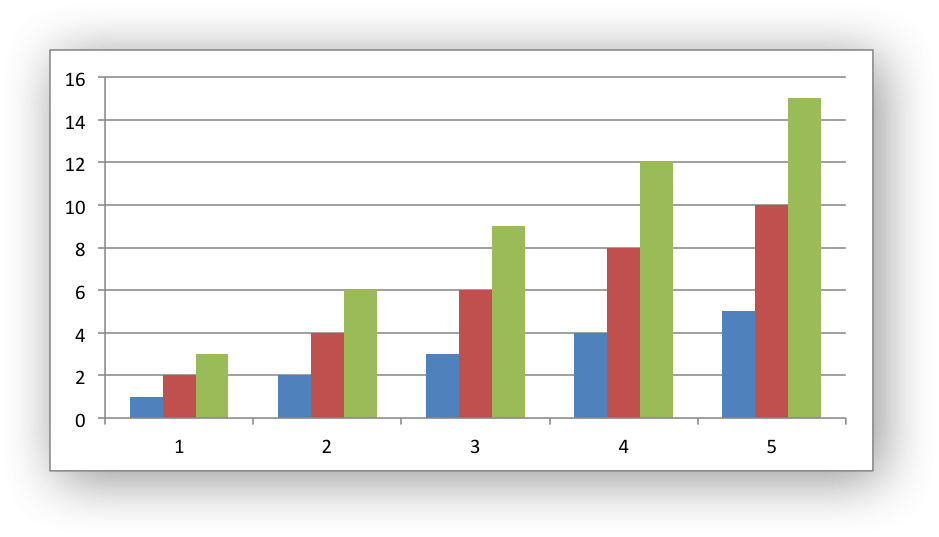Examples
chart_clustered.c, chart_data_labels.c, chart_data_table.c, and chart_fonts.c.

## ◆ chart_legend_set_font()

 void chart_legend_set_font ( lxw_chart * chart, lxw_chart_font * font )
Parameters
 chart Pointer to a lxw_chart instance to be configured. font A pointer to a chart lxw_chart_font font struct.

The `chart_legend_set_font()` function is used to set the font of a chart legend:

chart_legend_set_font(chart, &font);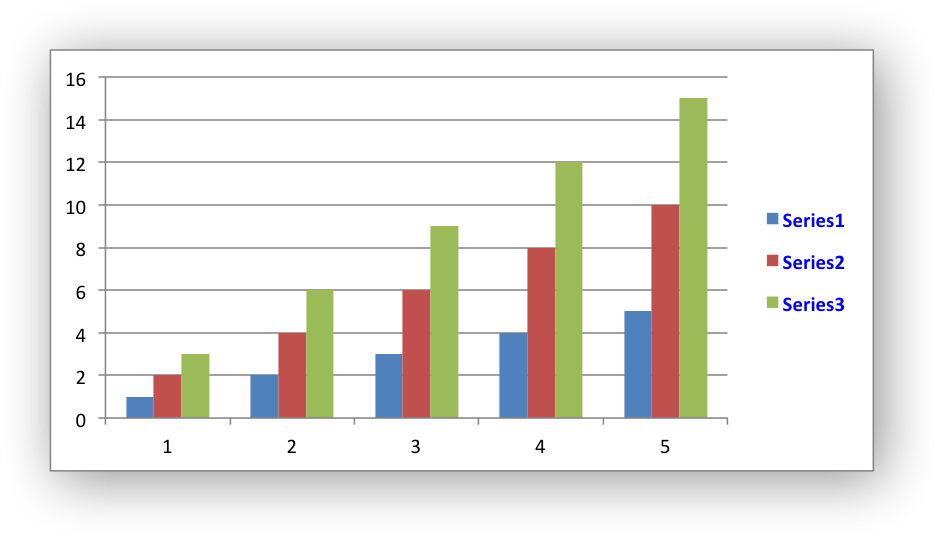Examples
chart_fonts.c.

## ◆ chart_legend_delete_series()

 lxw_error chart_legend_delete_series ( lxw_chart * chart, int16_t delete_series[] )
Parameters
 chart Pointer to a lxw_chart instance to be configured. delete_series An array of zero-indexed values to delete from series.
Returns
A lxw_error.

The `chart_legend_delete_series()` function allows you to remove/hide one or more series in a chart legend (the series will still display on the chart).

This function takes an array of one or more zero indexed series numbers. The array should be terminated with -1.

For example to remove the first and third zero-indexed series from the legend of a chart with 3 series:

int16_t series[] = {0, 2, -1};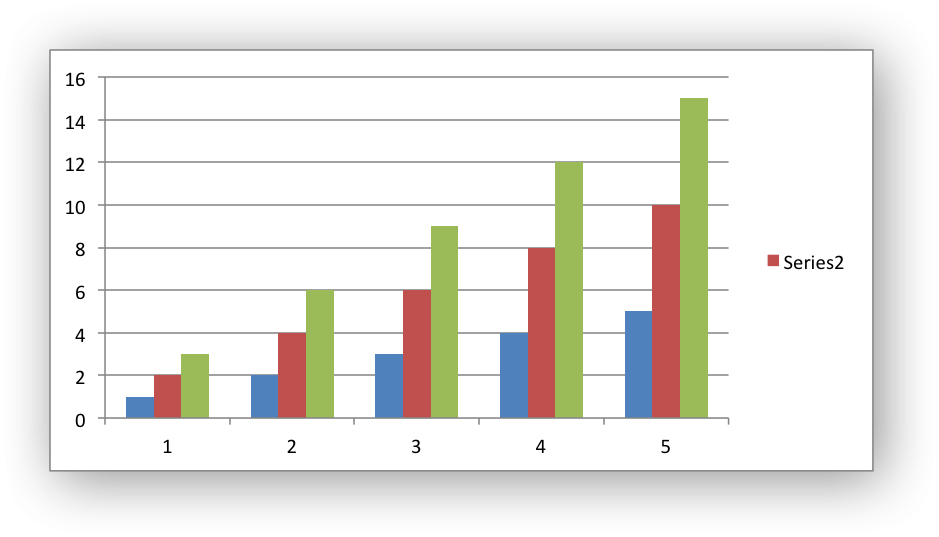## ◆ chart_chartarea_set_line()

 void chart_chartarea_set_line ( lxw_chart * chart, lxw_chart_line * line )
Parameters
 chart Pointer to a lxw_chart instance to be configured. line A lxw_chart_line struct.

Set the line/border properties of a chartarea. In Excel the chartarea is the background area behind the chart: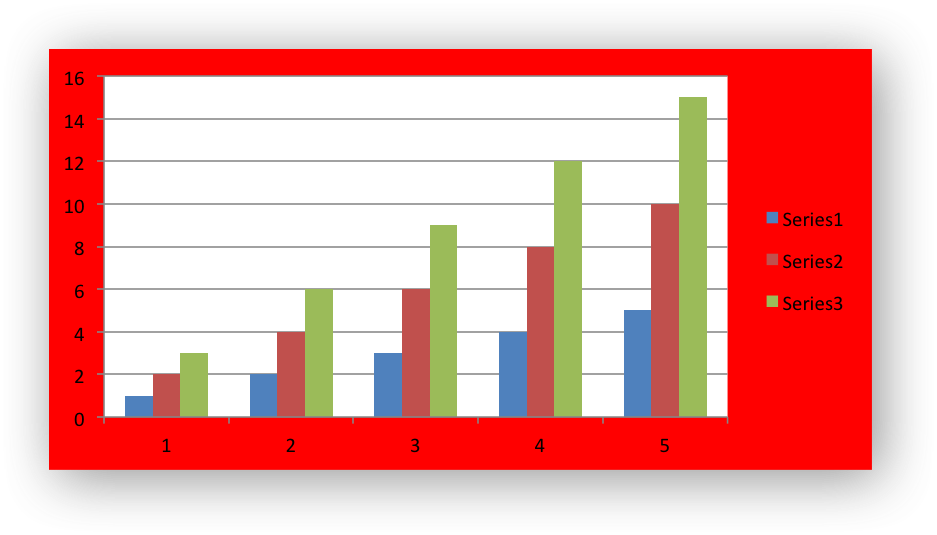## ◆ chart_chartarea_set_fill()

 void chart_chartarea_set_fill ( lxw_chart * chart, lxw_chart_fill * fill )
Parameters
 chart Pointer to a lxw_chart instance to be configured. fill A lxw_chart_fill struct.

Set the fill properties of a chartarea:

See the example and image above.

## ◆ chart_chartarea_set_pattern()

 void chart_chartarea_set_pattern ( lxw_chart * chart, lxw_chart_pattern * pattern )
Parameters
 chart Pointer to a lxw_chart instance to be configured. pattern A lxw_chart_pattern struct.

Set the pattern properties of a chartarea:

chart_chartarea_set_pattern(series1, &pattern);

## ◆ chart_plotarea_set_line()

 void chart_plotarea_set_line ( lxw_chart * chart, lxw_chart_line * line )
Parameters
 chart Pointer to a lxw_chart instance to be configured. line A lxw_chart_line struct.

Set the line/border properties of a plotarea. In Excel the plotarea is the area between the axes on which the chart series are plotted:

.width = 2,
.dash_type = LXW_CHART_LINE_DASH_DASH};
lxw_chart_fill fill = {.color = 0xFFFFC2};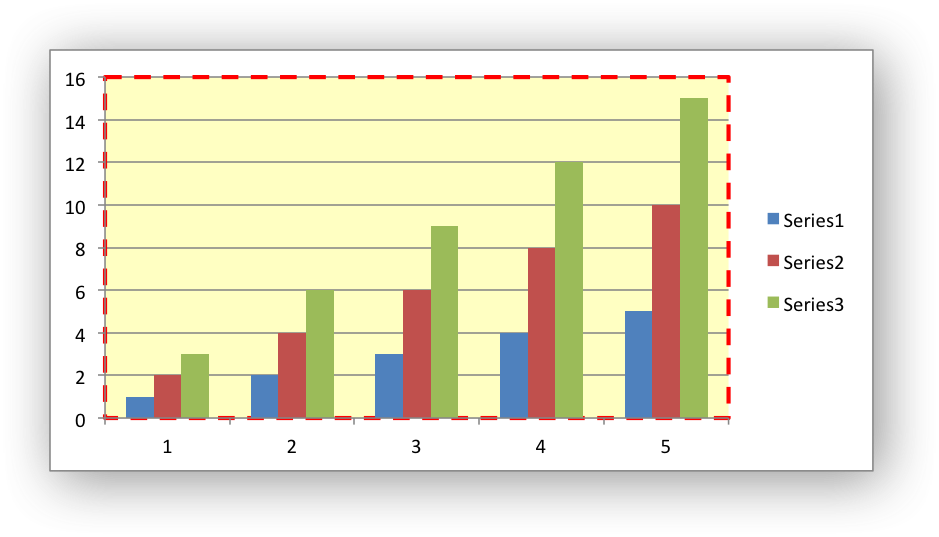## ◆ chart_plotarea_set_fill()

 void chart_plotarea_set_fill ( lxw_chart * chart, lxw_chart_fill * fill )
Parameters
 chart Pointer to a lxw_chart instance to be configured. fill A lxw_chart_fill struct.

Set the fill properties of a plotarea:

See the example and image above.

## ◆ chart_plotarea_set_pattern()

 void chart_plotarea_set_pattern ( lxw_chart * chart, lxw_chart_pattern * pattern )
Parameters
 chart Pointer to a lxw_chart instance to be configured. pattern A lxw_chart_pattern struct.

Set the pattern properties of a plotarea:

chart_plotarea_set_pattern(series1, &pattern);

## ◆ chart_set_style()

 void chart_set_style ( lxw_chart * chart, uint8_t style_id )
Parameters
 chart Pointer to a lxw_chart instance to be configured. style_id An index representing the chart style, 1 - 48.

The `chart_set_style()` function is used to set the style of the chart to one of the 48 built-in styles available on the "Design" tab in Excel 2007:

chart_set_style(chart, 37)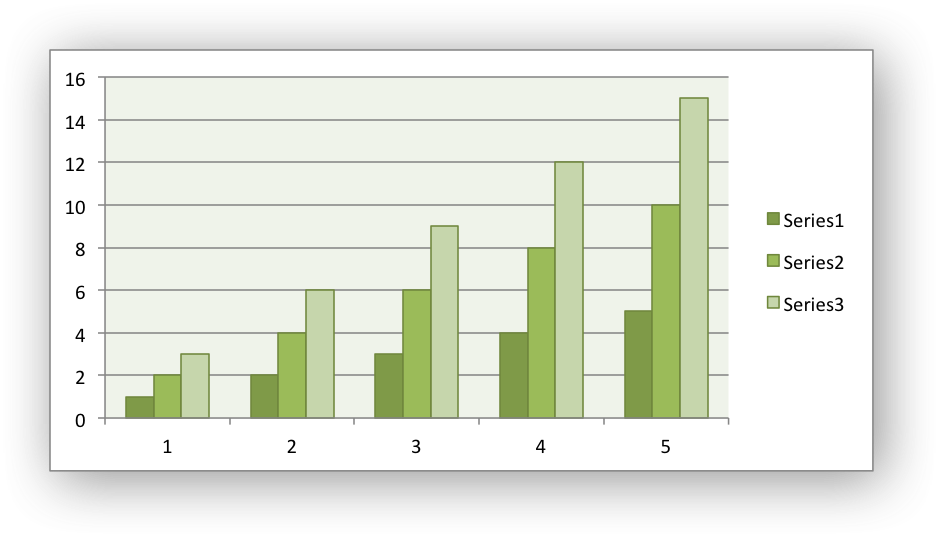The style index number is counted from 1 on the top left in the Excel dialog. The default style is 2.

Note:

In Excel 2013 the Styles section of the "Design" tab in Excel shows what were referred to as "Layouts" in previous versions of Excel. These layouts are not defined in the file format. They are a collection of modifications to the base chart type. They can not be defined by the `chart_set_style()`` function.

Examples
chart_area.c, chart_bar.c, chart_clustered.c, chart_column.c, chart_doughnut.c, chart_line.c, chart_pie.c, chart_radar.c, chart_scatter.c, chart_styles.c, and chartsheet.c.

## ◆ chart_set_table()

 void chart_set_table ( lxw_chart * chart )
Parameters
 chart Pointer to a lxw_chart instance to be configured.

The `chart_set_table()` function adds a data table below the horizontal axis with the data used to plot the chart:

// Turn on the data table with default options.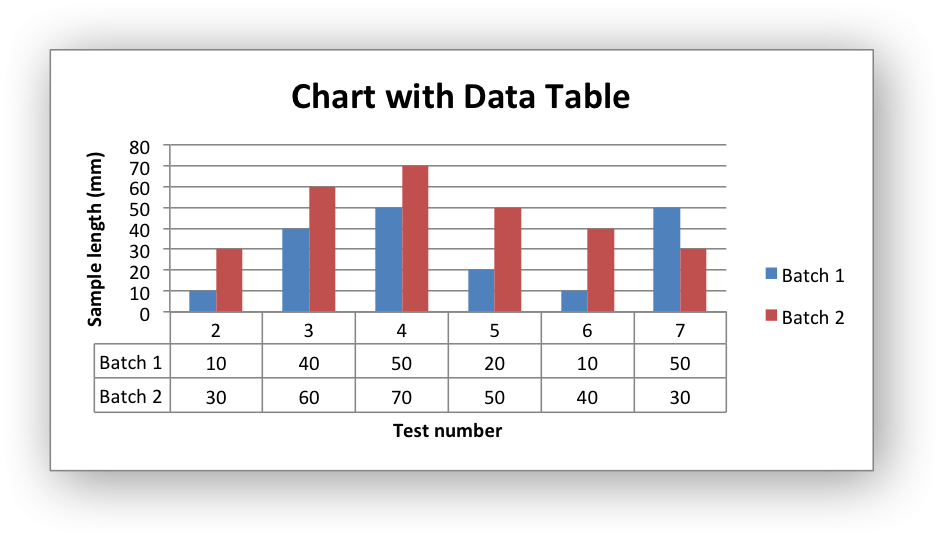The data table can only be shown with Bar, Column, Line and Area charts.

Examples
chart_data_table.c.

## ◆ chart_set_table_grid()

 void chart_set_table_grid ( lxw_chart * chart, uint8_t horizontal, uint8_t vertical, uint8_t outline, uint8_t legend_keys )
Parameters
 chart Pointer to a lxw_chart instance to be configured. horizontal Turn on/off the horizontal grid lines in the table. vertical Turn on/off the vertical grid lines in the table. outline Turn on/off the outline lines in the table. legend_keys Turn on/off the legend keys in the table.

The `chart_set_table_grid()` function turns on/off grid options for a chart data table. The data table grid options in Excel are shown in the dialog below: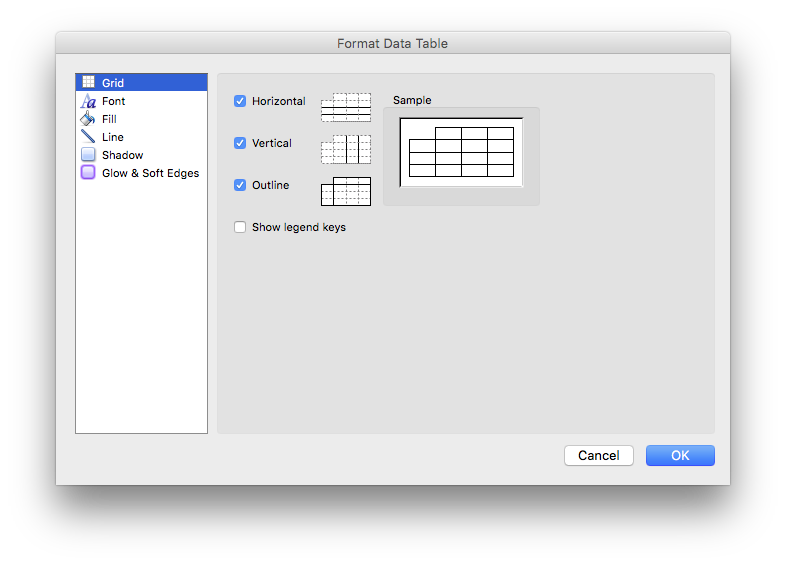These options can be passed to the `chart_set_table_grid()` function. The values for a default chart are:

• `horizontal`: On.
• `vertical`: On.
• `outline`: On.
• `legend_keys`: Off.

Example:

// Turn on the data table with default options.
// Turn on all grid lines and the grid legend.
// Turn off the legend since it is show in the table.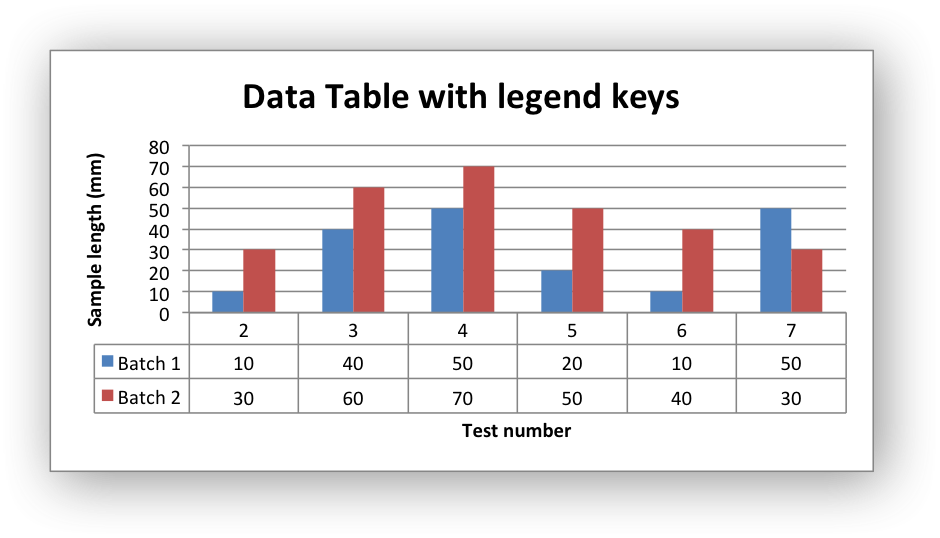The data table can only be shown with Bar, Column, Line and Area charts.

Examples
chart_data_table.c.

## ◆ chart_set_up_down_bars()

 void chart_set_up_down_bars ( lxw_chart * chart )
Parameters
 chart Pointer to a lxw_chart instance to be configured.

The `chart_set_up_down_bars()` function adds Up-Down bars to Line charts to indicate the difference between the first and last data series: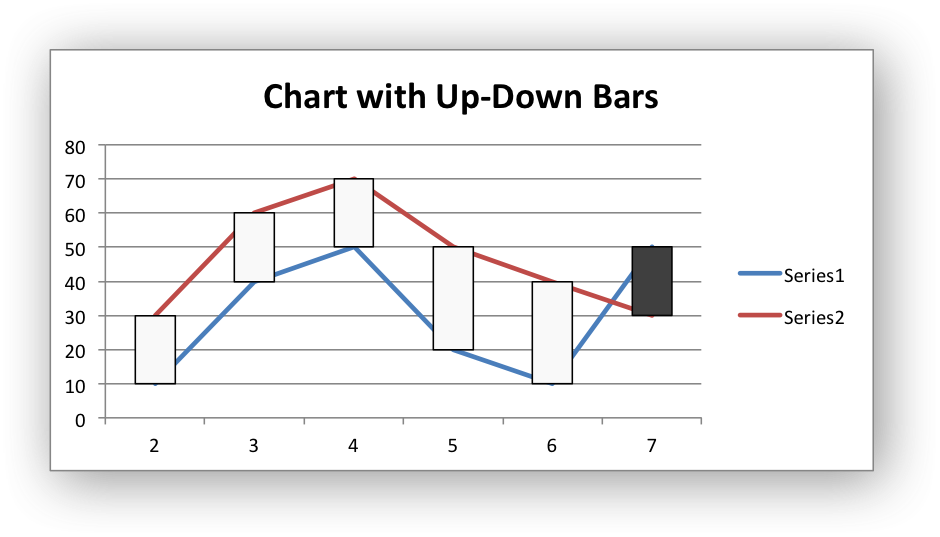Up-Down bars are only available in Line charts. By default Up-Down bars are black and white like in the above example. To format the border or fill of the bars see the `chart_set_up_down_bars_format()` function below.

Examples
chart_data_tools.c.

## ◆ chart_set_up_down_bars_format()

 void chart_set_up_down_bars_format ( lxw_chart * chart, lxw_chart_line * up_bar_line, lxw_chart_fill * up_bar_fill, lxw_chart_line * down_bar_line, lxw_chart_fill * down_bar_fill )
Parameters
 chart Pointer to a lxw_chart instance to be configured. up_bar_line A lxw_chart_line struct for the up-bar border. up_bar_fill A lxw_chart_fill struct for the up-bar fill. down_bar_line A lxw_chart_line struct for the down-bar border. down_bar_fill A lxw_chart_fill struct for the down-bar fill.

The `chart_set_up_down_bars_format()` function adds Up-Down bars to Line charts to indicate the difference between the first and last data series. It also allows the up and down bars to be formatted:

lxw_chart_fill up_fill = {.color = 0x00B050};
chart_set_up_down_bars_format(chart, &line, &up_fill, &line, &down_fill);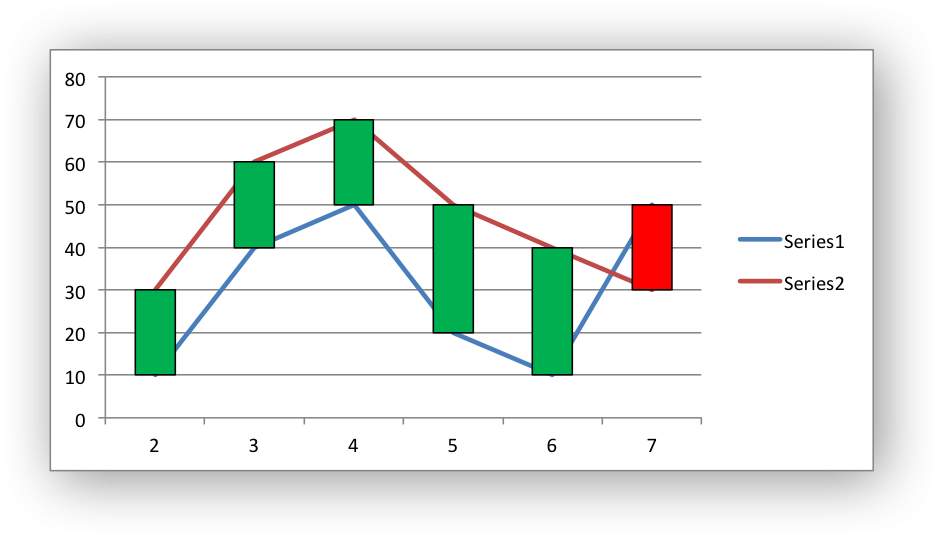Up-Down bars are only available in Line charts. For more format information see Chart formatting: Line and Chart formatting: Fill.

Examples
chart_data_tools.c.

## ◆ chart_set_drop_lines()

 void chart_set_drop_lines ( lxw_chart * chart, lxw_chart_line * line )
Parameters
 chart Pointer to a lxw_chart instance to be configured. line A lxw_chart_line struct.

The `chart_set_drop_lines()` function adds Drop Lines to charts to show the Category value of points in the data:

chart_set_drop_lines(chart, NULL);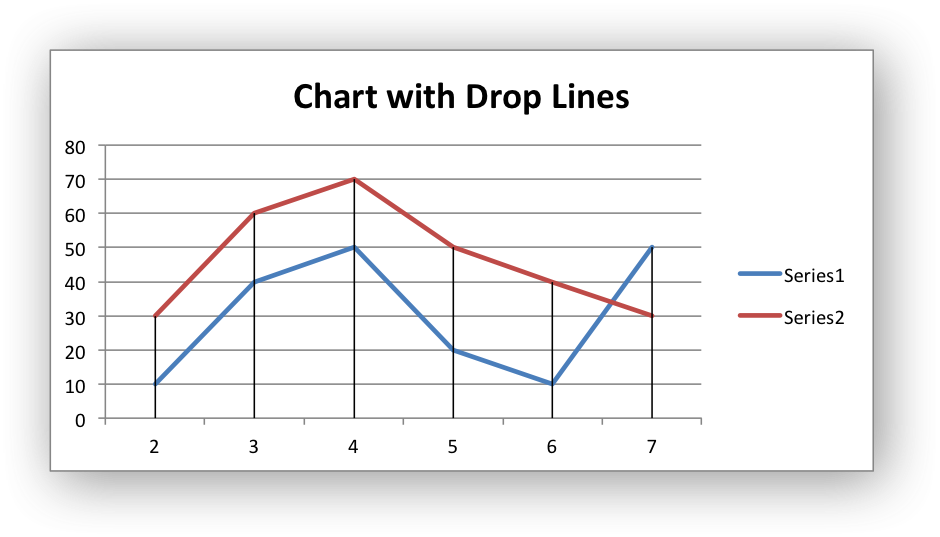It is possible to format the Drop Line line properties if required:

Drop Lines are only available in Line and Area charts. For more format information see Chart formatting: Line.

Examples
chart_data_tools.c.

## ◆ chart_set_high_low_lines()

 void chart_set_high_low_lines ( lxw_chart * chart, lxw_chart_line * line )
Parameters
 chart Pointer to a lxw_chart instance to be configured. line A lxw_chart_line struct.

The `chart_set_high_low_lines()` function adds High-Low Lines to charts to show the Category value of points in the data: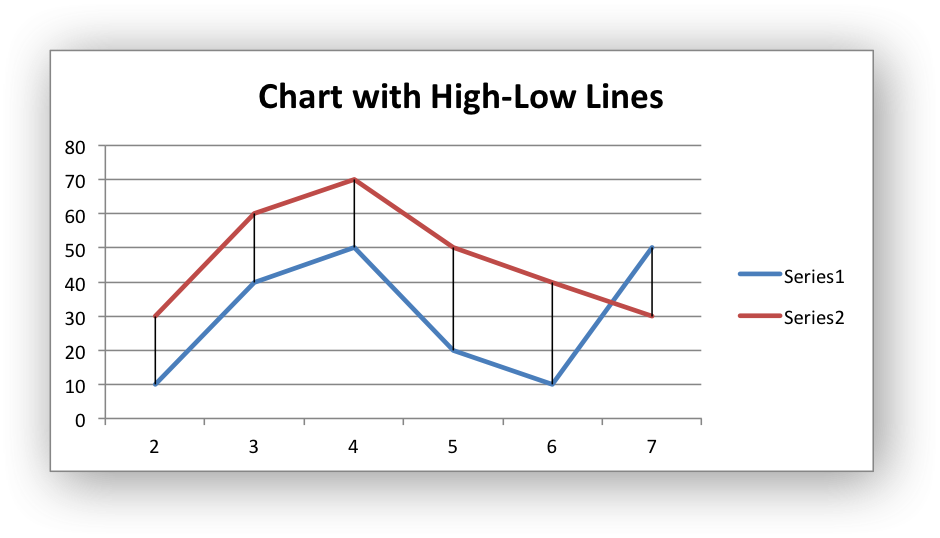It is possible to format the High-Low Line line properties if required:

High-Low Lines are only available in Line charts. For more format information see Chart formatting: Line.

Examples
chart_data_tools.c.

## ◆ chart_set_series_overlap()

 void chart_set_series_overlap ( lxw_chart * chart, int8_t overlap )
Parameters
 chart Pointer to a lxw_chart instance to be configured. overlap The overlap between the series. -100 to 100.

The `chart_set_series_overlap()` function sets the overlap between series in Bar and Column charts.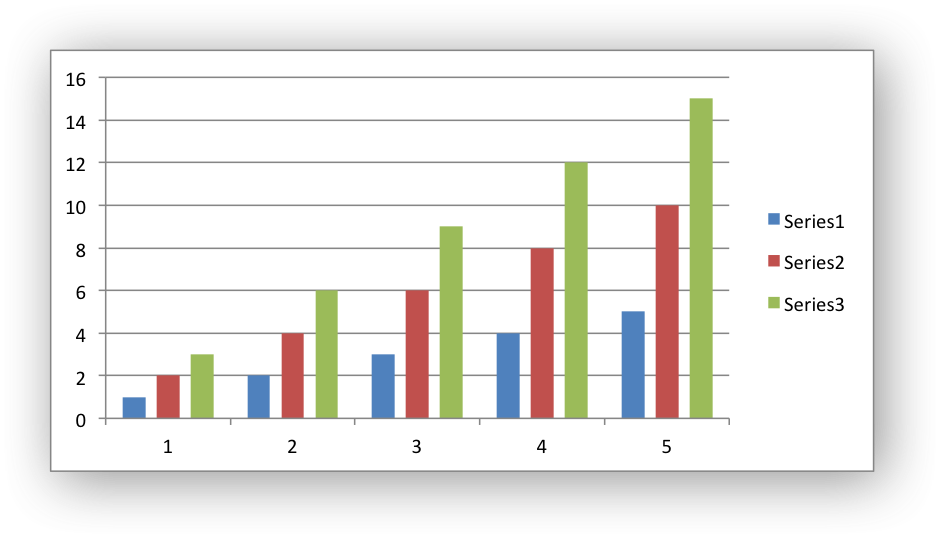The overlap value must be in the range `0 <= overlap <= 500`. The default value is 0.

This option is only available for Bar/Column charts.

## ◆ chart_set_series_gap()

 void chart_set_series_gap ( lxw_chart * chart, uint16_t gap )
Parameters
 chart Pointer to a lxw_chart instance to be configured. gap The gap between the series. 0 to 500.

The `chart_set_series_gap()` function sets the gap between series in Bar and Column charts.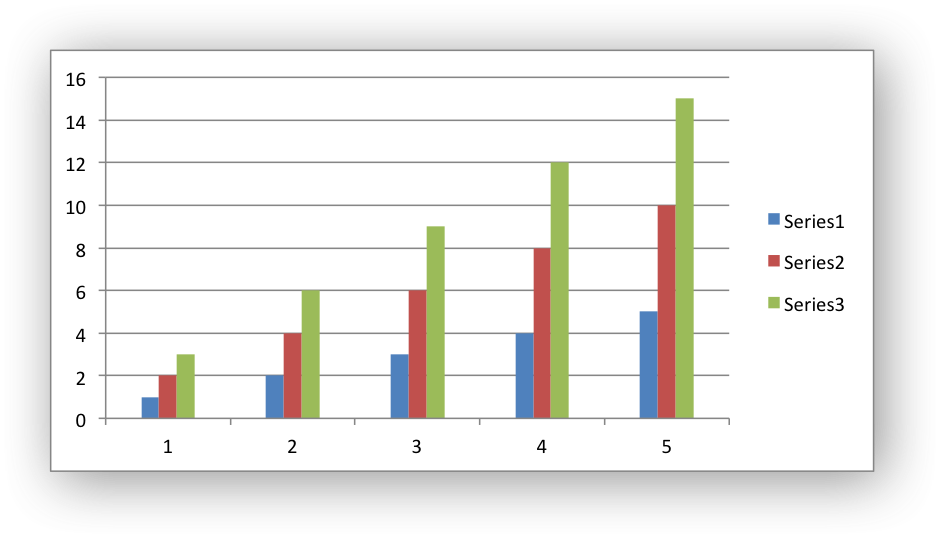The gap value must be in the range `0 <= gap <= 500`. The default value is 150.

This option is only available for Bar/Column charts.

Examples
chart_pattern.c.

## ◆ chart_show_blanks_as()

 void chart_show_blanks_as ( lxw_chart * chart, uint8_t option )
Parameters
 chart Pointer to a lxw_chart instance to be configured. option The display option. A lxw_chart_blank option.

The `chart_show_blanks_as()` function controls how blank data is displayed in a chart:

The `option` parameter can have one of the following values:

## ◆ chart_show_hidden_data()

 void chart_show_hidden_data ( lxw_chart * chart )
Parameters
 chart Pointer to a lxw_chart instance to be configured.

Display data that is in hidden rows or columns on the chart:

## ◆ chart_set_rotation()

 void chart_set_rotation ( lxw_chart * chart, uint16_t rotation )
Parameters
 chart Pointer to a lxw_chart instance to be configured. rotation The angle of rotation.

The `chart_set_rotation()` function is used to set the rotation of the first segment of a Pie/Doughnut chart. This has the effect of rotating the entire chart:

chart_set_rotation(chart, 28);

The angle of rotation must be in the range `0 <= rotation <= 360`.

This option is only available for Pie/Doughnut charts.

Examples
chart_doughnut.c, and chart_pie.c.

## ◆ chart_set_hole_size()

 void chart_set_hole_size ( lxw_chart * chart, uint8_t size )
Parameters
 chart Pointer to a lxw_chart instance to be configured. size The hole size as a percentage.

The `chart_set_hole_size()` function is used to set the hole size of a Doughnut chart:

The hole size must be in the range `10 <= size <= 90`.

This option is only available for Doughnut charts.

Examples
chart_doughnut.c.

## ◆ lxw_chart_line_dash_type

 typedef enum lxw_chart_line_dash_type lxw_chart_line_dash_type

The dash types are shown in the order that they appear in the Excel dialog. See Chart formatting: Line.

## ◆ lxw_chart_line

 typedef struct lxw_chart_line lxw_chart_line

## ◆ lxw_chart_fill

 typedef struct lxw_chart_fill lxw_chart_fill

## ◆ lxw_chart_pattern

 typedef struct lxw_chart_pattern lxw_chart_pattern

## ◆ lxw_chart_font

 typedef struct lxw_chart_font lxw_chart_font

## ◆ lxw_chart_point

 typedef struct lxw_chart_point lxw_chart_point

The lxw_chart_point used to set the line, fill and pattern of one or more points in a chart data series. See Working with Chart Points.

## ◆ lxw_chart_data_label

 typedef struct lxw_chart_data_label lxw_chart_data_label

The lxw_chart_data_label struct is used to represent a data label in a chart series so that custom properties can be set for it.

## ◆ lxw_chart_series

 typedef struct lxw_chart_series lxw_chart_series

The lxw_chart_series is created using the chart_add_series function. It is used in functions that modify a chart series but the members of the struct aren't modified directly.

## ◆ lxw_chart_axis

 typedef struct lxw_chart_axis lxw_chart_axis

The lxw_chart_axis struct is used in functions that modify a chart axis but the members of the struct aren't modified directly.

## ◆ lxw_chart

 typedef struct lxw_chart lxw_chart

The members of the lxw_chart struct aren't modified directly. Instead the chart properties are set by calling the functions shown in chart.h.

## ◆ lxw_chart_type

 enum lxw_chart_type
Enumerator
LXW_CHART_NONE

None.

LXW_CHART_AREA

Area chart.

LXW_CHART_AREA_STACKED

Area chart - stacked.

LXW_CHART_AREA_STACKED_PERCENT

Area chart - percentage stacked.

LXW_CHART_BAR

Bar chart.

LXW_CHART_BAR_STACKED

Bar chart - stacked.

LXW_CHART_BAR_STACKED_PERCENT

Bar chart - percentage stacked.

LXW_CHART_COLUMN

Column chart.

LXW_CHART_COLUMN_STACKED

Column chart - stacked.

LXW_CHART_COLUMN_STACKED_PERCENT

Column chart - percentage stacked.

LXW_CHART_DOUGHNUT

Doughnut chart.

LXW_CHART_LINE

Line chart.

LXW_CHART_LINE_STACKED

Line chart - stacked.

LXW_CHART_LINE_STACKED_PERCENT

Line chart - percentage stacked.

LXW_CHART_PIE

Pie chart.

LXW_CHART_SCATTER

Scatter chart.

LXW_CHART_SCATTER_STRAIGHT

Scatter chart - straight.

LXW_CHART_SCATTER_STRAIGHT_WITH_MARKERS

Scatter chart - straight with markers.

LXW_CHART_SCATTER_SMOOTH

Scatter chart - smooth.

LXW_CHART_SCATTER_SMOOTH_WITH_MARKERS

Scatter chart - smooth with markers.

## ◆ lxw_chart_legend_position

Enumerator
LXW_CHART_LEGEND_NONE

No chart legend.

LXW_CHART_LEGEND_RIGHT

Chart legend positioned at right side.

LXW_CHART_LEGEND_LEFT

Chart legend positioned at left side.

LXW_CHART_LEGEND_TOP

Chart legend positioned at top.

LXW_CHART_LEGEND_BOTTOM

Chart legend positioned at bottom.

LXW_CHART_LEGEND_TOP_RIGHT

Chart legend positioned at top right.

LXW_CHART_LEGEND_OVERLAY_RIGHT

Chart legend overlaid at right side.

LXW_CHART_LEGEND_OVERLAY_LEFT

Chart legend overlaid at left side.

LXW_CHART_LEGEND_OVERLAY_TOP_RIGHT

Chart legend overlaid at top right.

## ◆ lxw_chart_line_dash_type

The dash types are shown in the order that they appear in the Excel dialog. See Chart formatting: Line.

Enumerator
LXW_CHART_LINE_DASH_SOLID

Solid.

LXW_CHART_LINE_DASH_ROUND_DOT

Round Dot.

LXW_CHART_LINE_DASH_SQUARE_DOT

Square Dot.

LXW_CHART_LINE_DASH_DASH

Dash.

LXW_CHART_LINE_DASH_DASH_DOT

Dash Dot.

LXW_CHART_LINE_DASH_LONG_DASH

Long Dash.

LXW_CHART_LINE_DASH_LONG_DASH_DOT

Long Dash Dot.

LXW_CHART_LINE_DASH_LONG_DASH_DOT_DOT

Long Dash Dot Dot.

## ◆ lxw_chart_marker_type

Enumerator
LXW_CHART_MARKER_AUTOMATIC

Automatic, series default, marker type.

LXW_CHART_MARKER_NONE

No marker type.

LXW_CHART_MARKER_SQUARE

Square marker type.

LXW_CHART_MARKER_DIAMOND

Diamond marker type.

LXW_CHART_MARKER_TRIANGLE

Triangle marker type.

LXW_CHART_MARKER_X

X shape marker type.

LXW_CHART_MARKER_STAR

Star marker type.

LXW_CHART_MARKER_SHORT_DASH

Short dash marker type.

LXW_CHART_MARKER_LONG_DASH

Long dash marker type.

LXW_CHART_MARKER_CIRCLE

Circle marker type.

LXW_CHART_MARKER_PLUS

Plus (+) marker type.

## ◆ lxw_chart_pattern_type

Enumerator
LXW_CHART_PATTERN_NONE

None pattern.

LXW_CHART_PATTERN_PERCENT_5

5 Percent pattern.

LXW_CHART_PATTERN_PERCENT_10

10 Percent pattern.

LXW_CHART_PATTERN_PERCENT_20

20 Percent pattern.

LXW_CHART_PATTERN_PERCENT_25

25 Percent pattern.

LXW_CHART_PATTERN_PERCENT_30

30 Percent pattern.

LXW_CHART_PATTERN_PERCENT_40

40 Percent pattern.

LXW_CHART_PATTERN_PERCENT_50

50 Percent pattern.

LXW_CHART_PATTERN_PERCENT_60

60 Percent pattern.

LXW_CHART_PATTERN_PERCENT_70

70 Percent pattern.

LXW_CHART_PATTERN_PERCENT_75

75 Percent pattern.

LXW_CHART_PATTERN_PERCENT_80

80 Percent pattern.

LXW_CHART_PATTERN_PERCENT_90

90 Percent pattern.

LXW_CHART_PATTERN_LIGHT_DOWNWARD_DIAGONAL

Light downward diagonal pattern.

LXW_CHART_PATTERN_LIGHT_UPWARD_DIAGONAL

Light upward diagonal pattern.

LXW_CHART_PATTERN_DARK_DOWNWARD_DIAGONAL

Dark downward diagonal pattern.

LXW_CHART_PATTERN_DARK_UPWARD_DIAGONAL

Dark upward diagonal pattern.

LXW_CHART_PATTERN_WIDE_DOWNWARD_DIAGONAL

Wide downward diagonal pattern.

LXW_CHART_PATTERN_WIDE_UPWARD_DIAGONAL

Wide upward diagonal pattern.

LXW_CHART_PATTERN_LIGHT_VERTICAL

Light vertical pattern.

LXW_CHART_PATTERN_LIGHT_HORIZONTAL

Light horizontal pattern.

LXW_CHART_PATTERN_NARROW_VERTICAL

Narrow vertical pattern.

LXW_CHART_PATTERN_NARROW_HORIZONTAL

Narrow horizontal pattern.

LXW_CHART_PATTERN_DARK_VERTICAL

Dark vertical pattern.

LXW_CHART_PATTERN_DARK_HORIZONTAL

Dark horizontal pattern.

LXW_CHART_PATTERN_DASHED_DOWNWARD_DIAGONAL

Dashed downward diagonal pattern.

LXW_CHART_PATTERN_DASHED_UPWARD_DIAGONAL

Dashed upward diagonal pattern.

LXW_CHART_PATTERN_DASHED_HORIZONTAL

Dashed horizontal pattern.

LXW_CHART_PATTERN_DASHED_VERTICAL

Dashed vertical pattern.

LXW_CHART_PATTERN_SMALL_CONFETTI

Small confetti pattern.

LXW_CHART_PATTERN_LARGE_CONFETTI

Large confetti pattern.

LXW_CHART_PATTERN_ZIGZAG

Zigzag pattern.

LXW_CHART_PATTERN_WAVE

Wave pattern.

LXW_CHART_PATTERN_DIAGONAL_BRICK

Diagonal brick pattern.

LXW_CHART_PATTERN_HORIZONTAL_BRICK

Horizontal brick pattern.

LXW_CHART_PATTERN_WEAVE

Weave pattern.

LXW_CHART_PATTERN_PLAID

Plaid pattern.

LXW_CHART_PATTERN_DIVOT

Divot pattern.

LXW_CHART_PATTERN_DOTTED_GRID

Dotted grid pattern.

LXW_CHART_PATTERN_DOTTED_DIAMOND

Dotted diamond pattern.

LXW_CHART_PATTERN_SHINGLE

Shingle pattern.

LXW_CHART_PATTERN_TRELLIS

Trellis pattern.

LXW_CHART_PATTERN_SPHERE

Sphere pattern.

LXW_CHART_PATTERN_SMALL_GRID

Small grid pattern.

LXW_CHART_PATTERN_LARGE_GRID

Large grid pattern.

LXW_CHART_PATTERN_SMALL_CHECK

Small check pattern.

LXW_CHART_PATTERN_LARGE_CHECK

Large check pattern.

LXW_CHART_PATTERN_OUTLINED_DIAMOND

Outlined diamond pattern.

LXW_CHART_PATTERN_SOLID_DIAMOND

Solid diamond pattern.

## ◆ lxw_chart_label_position

Enumerator
LXW_CHART_LABEL_POSITION_DEFAULT

Series data label position: default position.

LXW_CHART_LABEL_POSITION_CENTER

Series data label position: center.

LXW_CHART_LABEL_POSITION_RIGHT

Series data label position: right.

LXW_CHART_LABEL_POSITION_LEFT

Series data label position: left.

LXW_CHART_LABEL_POSITION_ABOVE

Series data label position: above.

LXW_CHART_LABEL_POSITION_BELOW

Series data label position: below.

LXW_CHART_LABEL_POSITION_INSIDE_BASE

Series data label position: inside base.

LXW_CHART_LABEL_POSITION_INSIDE_END

Series data label position: inside end.

LXW_CHART_LABEL_POSITION_OUTSIDE_END

Series data label position: outside end.

LXW_CHART_LABEL_POSITION_BEST_FIT

Series data label position: best fit.

## ◆ lxw_chart_label_separator

Enumerator
LXW_CHART_LABEL_SEPARATOR_COMMA

Series data label separator: comma (the default).

LXW_CHART_LABEL_SEPARATOR_SEMICOLON

Series data label separator: semicolon.

LXW_CHART_LABEL_SEPARATOR_PERIOD

Series data label separator: period.

LXW_CHART_LABEL_SEPARATOR_NEWLINE

Series data label separator: newline.

LXW_CHART_LABEL_SEPARATOR_SPACE

Series data label separator: space.

## ◆ lxw_chart_axis_type

 enum lxw_chart_axis_type
Enumerator
LXW_CHART_AXIS_TYPE_X

Chart X axis.

LXW_CHART_AXIS_TYPE_Y

Chart Y axis.

## ◆ lxw_chart_axis_tick_position

Enumerator
LXW_CHART_AXIS_POSITION_ON_TICK

Position category axis on tick marks.

LXW_CHART_AXIS_POSITION_BETWEEN

Position category axis between tick marks.

## ◆ lxw_chart_axis_label_position

Enumerator
LXW_CHART_AXIS_LABEL_POSITION_NEXT_TO

Position the axis labels next to the axis. The default.

LXW_CHART_AXIS_LABEL_POSITION_HIGH

Position the axis labels at the top of the chart, for horizontal axes, or to the right for vertical axes.

LXW_CHART_AXIS_LABEL_POSITION_LOW

Position the axis labels at the bottom of the chart, for horizontal axes, or to the left for vertical axes.

LXW_CHART_AXIS_LABEL_POSITION_NONE

Turn off the the axis labels.

## ◆ lxw_chart_axis_label_alignment

Enumerator
LXW_CHART_AXIS_LABEL_ALIGN_CENTER

Chart axis label alignment: center.

LXW_CHART_AXIS_LABEL_ALIGN_LEFT

Chart axis label alignment: left.

LXW_CHART_AXIS_LABEL_ALIGN_RIGHT

Chart axis label alignment: right.

## ◆ lxw_chart_axis_display_unit

Enumerator
LXW_CHART_AXIS_UNITS_NONE

Axis display units: None. The default.

LXW_CHART_AXIS_UNITS_HUNDREDS

Axis display units: Hundreds.

LXW_CHART_AXIS_UNITS_THOUSANDS

Axis display units: Thousands.

LXW_CHART_AXIS_UNITS_TEN_THOUSANDS

Axis display units: Ten thousands.

LXW_CHART_AXIS_UNITS_HUNDRED_THOUSANDS

Axis display units: Hundred thousands.

LXW_CHART_AXIS_UNITS_MILLIONS

Axis display units: Millions.

LXW_CHART_AXIS_UNITS_TEN_MILLIONS

Axis display units: Ten millions.

LXW_CHART_AXIS_UNITS_HUNDRED_MILLIONS

Axis display units: Hundred millions.

LXW_CHART_AXIS_UNITS_BILLIONS

Axis display units: Billions.

LXW_CHART_AXIS_UNITS_TRILLIONS

Axis display units: Trillions.

## ◆ lxw_chart_axis_tick_mark

Enumerator
LXW_CHART_AXIS_TICK_MARK_DEFAULT

Default tick mark for the chart axis. Usually outside.

LXW_CHART_AXIS_TICK_MARK_NONE

No tick mark for the axis.

LXW_CHART_AXIS_TICK_MARK_INSIDE

Tick mark inside the axis only.

LXW_CHART_AXIS_TICK_MARK_OUTSIDE

Tick mark outside the axis only.

LXW_CHART_AXIS_TICK_MARK_CROSSING

Tick mark inside and outside the axis.

## ◆ lxw_chart_blank

 enum lxw_chart_blank
Enumerator
LXW_CHART_BLANKS_AS_GAP

Show empty chart cells as gaps in the data. The default.

LXW_CHART_BLANKS_AS_ZERO

Show empty chart cells as zeros.

LXW_CHART_BLANKS_AS_CONNECTED

Show empty chart cells as connected. Only for charts with lines.

## ◆ lxw_chart_error_bar_type

Enumerator
LXW_CHART_ERROR_BAR_TYPE_STD_ERROR

Error bar type: Standard error.

LXW_CHART_ERROR_BAR_TYPE_FIXED

Error bar type: Fixed value.

LXW_CHART_ERROR_BAR_TYPE_PERCENTAGE

Error bar type: Percentage.

LXW_CHART_ERROR_BAR_TYPE_STD_DEV

Error bar type: Standard deviation(s).

## ◆ lxw_chart_error_bar_direction

Enumerator
LXW_CHART_ERROR_BAR_DIR_BOTH

Error bar extends in both directions. The default.

LXW_CHART_ERROR_BAR_DIR_PLUS

Error bar extends in positive direction.

LXW_CHART_ERROR_BAR_DIR_MINUS

Error bar extends in negative direction.

## ◆ lxw_chart_error_bar_axis

Enumerator
LXW_CHART_ERROR_BAR_AXIS_X

X axis error bar.

LXW_CHART_ERROR_BAR_AXIS_Y

Y axis error bar.

## ◆ lxw_chart_error_bar_cap

Enumerator
LXW_CHART_ERROR_BAR_END_CAP

Flat end cap. The default.

LXW_CHART_ERROR_BAR_NO_CAP

No end cap.

## ◆ lxw_chart_trendline_type

Enumerator
LXW_CHART_TRENDLINE_TYPE_LINEAR

Trendline type: Linear.

LXW_CHART_TRENDLINE_TYPE_LOG

Trendline type: Logarithm.

LXW_CHART_TRENDLINE_TYPE_POLY

Trendline type: Polynomial.

LXW_CHART_TRENDLINE_TYPE_POWER

Trendline type: Power.

LXW_CHART_TRENDLINE_TYPE_EXP

Trendline type: Exponential.

LXW_CHART_TRENDLINE_TYPE_AVERAGE

Trendline type: Moving Average.

## Data Structures

struct  lxw_chart_line
Struct to represent a chart line. More...

struct  lxw_chart_fill
Struct to represent a chart fill. More...

struct  lxw_chart_pattern
Struct to represent a chart pattern. More...

struct  lxw_chart_font
Struct to represent a chart font. More...

struct  lxw_chart_point
Struct to represent an Excel chart data point. More...

struct  lxw_chart_data_label
Struct to represent an Excel chart data label. More...

struct  lxw_chart_series
Struct to represent an Excel chart data series. More...

struct  lxw_chart_axis
Struct to represent an Excel chart axis. More...

struct  lxw_chart
Struct to represent an Excel chart. More...

## Typedefs

typedef enum lxw_chart_type lxw_chart_type
Available chart types.

typedef enum lxw_chart_legend_position lxw_chart_legend_position
Chart legend positions.

typedef enum lxw_chart_line_dash_type lxw_chart_line_dash_type
Chart line dash types. More...

typedef enum lxw_chart_marker_type lxw_chart_marker_type
Chart marker types.

typedef enum lxw_chart_pattern_type lxw_chart_pattern_type
Chart pattern types.

typedef enum lxw_chart_label_position lxw_chart_label_position
Chart data label positions.

typedef enum lxw_chart_label_separator lxw_chart_label_separator
Chart data label separator.

typedef enum lxw_chart_axis_type lxw_chart_axis_type
Chart axis types.

typedef enum lxw_chart_axis_tick_position lxw_chart_axis_tick_position
Axis positions for category axes.

typedef enum lxw_chart_axis_label_position lxw_chart_axis_label_position
Axis label positions.

typedef enum lxw_chart_axis_label_alignment lxw_chart_axis_label_alignment
Axis label alignments.

typedef enum lxw_chart_axis_display_unit lxw_chart_axis_display_unit
Display units for chart value axis.

typedef enum lxw_chart_axis_tick_mark lxw_chart_tick_mark
Tick mark types for an axis.

typedef struct lxw_chart_line lxw_chart_line
Struct to represent a chart line. More...

typedef struct lxw_chart_fill lxw_chart_fill
Struct to represent a chart fill. More...

typedef struct lxw_chart_pattern lxw_chart_pattern
Struct to represent a chart pattern. More...

typedef struct lxw_chart_font lxw_chart_font
Struct to represent a chart font. More...

typedef struct lxw_chart_point lxw_chart_point
Struct to represent an Excel chart data point. More...

typedef struct lxw_chart_data_label lxw_chart_data_label
Struct to represent an Excel chart data label. More...

typedef enum lxw_chart_blank lxw_chart_blank
Define how blank values are displayed in a chart.

typedef enum lxw_chart_error_bar_type lxw_chart_error_bar_type
Type/amount of data series error bar.

typedef enum lxw_chart_error_bar_direction lxw_chart_error_bar_direction
Direction for a data series error bar.

typedef enum lxw_chart_error_bar_axis lxw_chart_error_bar_axis
Direction for a data series error bar.

typedef enum lxw_chart_error_bar_cap lxw_chart_error_bar_cap
End cap styles for a data series error bar.

typedef enum lxw_chart_trendline_type lxw_chart_trendline_type
Series trendline/regression types.

typedef struct lxw_chart_series lxw_chart_series
Struct to represent an Excel chart data series. More...

typedef struct lxw_chart_axis lxw_chart_axis
Struct to represent an Excel chart axis. More...

typedef struct lxw_chart lxw_chart
Struct to represent an Excel chart. More...

## Enumerations

enum  lxw_chart_type {
LXW_CHART_NONE = 0, LXW_CHART_AREA, LXW_CHART_AREA_STACKED, LXW_CHART_AREA_STACKED_PERCENT,
LXW_CHART_BAR, LXW_CHART_BAR_STACKED, LXW_CHART_BAR_STACKED_PERCENT, LXW_CHART_COLUMN,
LXW_CHART_COLUMN_STACKED, LXW_CHART_COLUMN_STACKED_PERCENT, LXW_CHART_DOUGHNUT, LXW_CHART_LINE,
LXW_CHART_LINE_STACKED, LXW_CHART_LINE_STACKED_PERCENT, LXW_CHART_PIE, LXW_CHART_SCATTER,
LXW_CHART_SCATTER_STRAIGHT, LXW_CHART_SCATTER_STRAIGHT_WITH_MARKERS, LXW_CHART_SCATTER_SMOOTH, LXW_CHART_SCATTER_SMOOTH_WITH_MARKERS,
}
Available chart types. More...

enum  lxw_chart_legend_position {
LXW_CHART_LEGEND_NONE = 0, LXW_CHART_LEGEND_RIGHT, LXW_CHART_LEGEND_LEFT, LXW_CHART_LEGEND_TOP,
LXW_CHART_LEGEND_BOTTOM, LXW_CHART_LEGEND_TOP_RIGHT, LXW_CHART_LEGEND_OVERLAY_RIGHT, LXW_CHART_LEGEND_OVERLAY_LEFT,
LXW_CHART_LEGEND_OVERLAY_TOP_RIGHT
}
Chart legend positions. More...

enum  lxw_chart_line_dash_type {
LXW_CHART_LINE_DASH_SOLID = 0, LXW_CHART_LINE_DASH_ROUND_DOT, LXW_CHART_LINE_DASH_SQUARE_DOT, LXW_CHART_LINE_DASH_DASH,
LXW_CHART_LINE_DASH_DASH_DOT, LXW_CHART_LINE_DASH_LONG_DASH, LXW_CHART_LINE_DASH_LONG_DASH_DOT, LXW_CHART_LINE_DASH_LONG_DASH_DOT_DOT
}
Chart line dash types. More...

enum  lxw_chart_marker_type {
LXW_CHART_MARKER_AUTOMATIC, LXW_CHART_MARKER_NONE, LXW_CHART_MARKER_SQUARE, LXW_CHART_MARKER_DIAMOND,
LXW_CHART_MARKER_TRIANGLE, LXW_CHART_MARKER_X, LXW_CHART_MARKER_STAR, LXW_CHART_MARKER_SHORT_DASH,
LXW_CHART_MARKER_LONG_DASH, LXW_CHART_MARKER_CIRCLE, LXW_CHART_MARKER_PLUS
}
Chart marker types. More...

enum  lxw_chart_pattern_type {
LXW_CHART_PATTERN_NONE, LXW_CHART_PATTERN_PERCENT_5, LXW_CHART_PATTERN_PERCENT_10, LXW_CHART_PATTERN_PERCENT_20,
LXW_CHART_PATTERN_PERCENT_25, LXW_CHART_PATTERN_PERCENT_30, LXW_CHART_PATTERN_PERCENT_40, LXW_CHART_PATTERN_PERCENT_50,
LXW_CHART_PATTERN_PERCENT_60, LXW_CHART_PATTERN_PERCENT_70, LXW_CHART_PATTERN_PERCENT_75, LXW_CHART_PATTERN_PERCENT_80,
LXW_CHART_PATTERN_PERCENT_90, LXW_CHART_PATTERN_LIGHT_DOWNWARD_DIAGONAL, LXW_CHART_PATTERN_LIGHT_UPWARD_DIAGONAL, LXW_CHART_PATTERN_DARK_DOWNWARD_DIAGONAL,
LXW_CHART_PATTERN_DARK_UPWARD_DIAGONAL, LXW_CHART_PATTERN_WIDE_DOWNWARD_DIAGONAL, LXW_CHART_PATTERN_WIDE_UPWARD_DIAGONAL, LXW_CHART_PATTERN_LIGHT_VERTICAL,
LXW_CHART_PATTERN_LIGHT_HORIZONTAL, LXW_CHART_PATTERN_NARROW_VERTICAL, LXW_CHART_PATTERN_NARROW_HORIZONTAL, LXW_CHART_PATTERN_DARK_VERTICAL,
LXW_CHART_PATTERN_DARK_HORIZONTAL, LXW_CHART_PATTERN_DASHED_DOWNWARD_DIAGONAL, LXW_CHART_PATTERN_DASHED_UPWARD_DIAGONAL, LXW_CHART_PATTERN_DASHED_HORIZONTAL,
LXW_CHART_PATTERN_DASHED_VERTICAL, LXW_CHART_PATTERN_SMALL_CONFETTI, LXW_CHART_PATTERN_LARGE_CONFETTI, LXW_CHART_PATTERN_ZIGZAG,
LXW_CHART_PATTERN_WAVE, LXW_CHART_PATTERN_DIAGONAL_BRICK, LXW_CHART_PATTERN_HORIZONTAL_BRICK, LXW_CHART_PATTERN_WEAVE,
LXW_CHART_PATTERN_PLAID, LXW_CHART_PATTERN_DIVOT, LXW_CHART_PATTERN_DOTTED_GRID, LXW_CHART_PATTERN_DOTTED_DIAMOND,
LXW_CHART_PATTERN_SHINGLE, LXW_CHART_PATTERN_TRELLIS, LXW_CHART_PATTERN_SPHERE, LXW_CHART_PATTERN_SMALL_GRID,
LXW_CHART_PATTERN_LARGE_GRID, LXW_CHART_PATTERN_SMALL_CHECK, LXW_CHART_PATTERN_LARGE_CHECK, LXW_CHART_PATTERN_OUTLINED_DIAMOND,
LXW_CHART_PATTERN_SOLID_DIAMOND
}
Chart pattern types. More...

enum  lxw_chart_label_position {
LXW_CHART_LABEL_POSITION_DEFAULT, LXW_CHART_LABEL_POSITION_CENTER, LXW_CHART_LABEL_POSITION_RIGHT, LXW_CHART_LABEL_POSITION_LEFT,
LXW_CHART_LABEL_POSITION_ABOVE, LXW_CHART_LABEL_POSITION_BELOW, LXW_CHART_LABEL_POSITION_INSIDE_BASE, LXW_CHART_LABEL_POSITION_INSIDE_END,
LXW_CHART_LABEL_POSITION_OUTSIDE_END, LXW_CHART_LABEL_POSITION_BEST_FIT
}
Chart data label positions. More...

enum  lxw_chart_label_separator {
LXW_CHART_LABEL_SEPARATOR_COMMA, LXW_CHART_LABEL_SEPARATOR_SEMICOLON, LXW_CHART_LABEL_SEPARATOR_PERIOD, LXW_CHART_LABEL_SEPARATOR_NEWLINE,
LXW_CHART_LABEL_SEPARATOR_SPACE
}
Chart data label separator. More...

enum  lxw_chart_axis_type { LXW_CHART_AXIS_TYPE_X, LXW_CHART_AXIS_TYPE_Y }
Chart axis types. More...

enum  lxw_chart_axis_tick_position { , LXW_CHART_AXIS_POSITION_ON_TICK, LXW_CHART_AXIS_POSITION_BETWEEN }
Axis positions for category axes. More...

enum  lxw_chart_axis_label_position { LXW_CHART_AXIS_LABEL_POSITION_NEXT_TO, LXW_CHART_AXIS_LABEL_POSITION_HIGH, LXW_CHART_AXIS_LABEL_POSITION_LOW, LXW_CHART_AXIS_LABEL_POSITION_NONE }
Axis label positions. More...

enum  lxw_chart_axis_label_alignment { LXW_CHART_AXIS_LABEL_ALIGN_CENTER, LXW_CHART_AXIS_LABEL_ALIGN_LEFT, LXW_CHART_AXIS_LABEL_ALIGN_RIGHT }
Axis label alignments. More...

enum  lxw_chart_axis_display_unit {
LXW_CHART_AXIS_UNITS_NONE, LXW_CHART_AXIS_UNITS_HUNDREDS, LXW_CHART_AXIS_UNITS_THOUSANDS, LXW_CHART_AXIS_UNITS_TEN_THOUSANDS,
LXW_CHART_AXIS_UNITS_HUNDRED_THOUSANDS, LXW_CHART_AXIS_UNITS_MILLIONS, LXW_CHART_AXIS_UNITS_TEN_MILLIONS, LXW_CHART_AXIS_UNITS_HUNDRED_MILLIONS,
LXW_CHART_AXIS_UNITS_BILLIONS, LXW_CHART_AXIS_UNITS_TRILLIONS
}
Display units for chart value axis. More...

enum  lxw_chart_axis_tick_mark {
LXW_CHART_AXIS_TICK_MARK_DEFAULT, LXW_CHART_AXIS_TICK_MARK_NONE, LXW_CHART_AXIS_TICK_MARK_INSIDE, LXW_CHART_AXIS_TICK_MARK_OUTSIDE,
LXW_CHART_AXIS_TICK_MARK_CROSSING
}
Tick mark types for an axis. More...

enum  lxw_chart_blank { LXW_CHART_BLANKS_AS_GAP, LXW_CHART_BLANKS_AS_ZERO, LXW_CHART_BLANKS_AS_CONNECTED }
Define how blank values are displayed in a chart. More...

enum  lxw_chart_error_bar_type { LXW_CHART_ERROR_BAR_TYPE_STD_ERROR, LXW_CHART_ERROR_BAR_TYPE_FIXED, LXW_CHART_ERROR_BAR_TYPE_PERCENTAGE, LXW_CHART_ERROR_BAR_TYPE_STD_DEV }
Type/amount of data series error bar. More...

enum  lxw_chart_error_bar_direction { LXW_CHART_ERROR_BAR_DIR_BOTH, LXW_CHART_ERROR_BAR_DIR_PLUS, LXW_CHART_ERROR_BAR_DIR_MINUS }
Direction for a data series error bar. More...

enum  lxw_chart_error_bar_axis { LXW_CHART_ERROR_BAR_AXIS_X, LXW_CHART_ERROR_BAR_AXIS_Y }
Direction for a data series error bar. More...

enum  lxw_chart_error_bar_cap { LXW_CHART_ERROR_BAR_END_CAP, LXW_CHART_ERROR_BAR_NO_CAP }
End cap styles for a data series error bar. More...

enum  lxw_chart_trendline_type {
LXW_CHART_TRENDLINE_TYPE_LINEAR, LXW_CHART_TRENDLINE_TYPE_LOG, LXW_CHART_TRENDLINE_TYPE_POLY, LXW_CHART_TRENDLINE_TYPE_POWER,
LXW_CHART_TRENDLINE_TYPE_EXP, LXW_CHART_TRENDLINE_TYPE_AVERAGE
}
Series trendline/regression types. More...

chart_series_set_marker_type
void chart_series_set_marker_type(lxw_chart_series *series, uint8_t type)
Set the data marker type for a series.
chart_set_style
void chart_set_style(lxw_chart *chart, uint8_t style_id)
Set the chart style type.
LXW_CHART_LINE_DASH_LONG_DASH
@ LXW_CHART_LINE_DASH_LONG_DASH
Definition: chart.h:218
lxw_chart_pattern
Struct to represent a chart pattern.
Definition: chart.h:681
chart_series_set_trendline_equation
void chart_series_set_trendline_equation(lxw_chart_series *series)
Display the equation of a trendline for a chart data series.
chart_series_set_marker_fill
void chart_series_set_marker_fill(lxw_chart_series *series, lxw_chart_fill *fill)
Set the fill properties for a chart series marker.
workbook_close
lxw_error workbook_close(lxw_workbook *workbook)
Close the Workbook object and write the XLSX file.
chart_series_set_trendline
void chart_series_set_trendline(lxw_chart_series *series, uint8_t type, uint8_t value)
Turn on a trendline for a chart data series.
chart_series_set_points
lxw_error chart_series_set_points(lxw_chart_series *series, lxw_chart_point *points[])
Set the formatting for points in the series.
chart_axis_set_minor_tick_mark
void chart_axis_set_minor_tick_mark(lxw_chart_axis *axis, uint8_t type)
Set the minor axis tick mark type.
chart_series_set_labels_custom
lxw_error chart_series_set_labels_custom(lxw_chart_series *series, lxw_chart_data_label *data_labels[])
Set the properties for data labels in a series.
chart_series_set_pattern
void chart_series_set_pattern(lxw_chart_series *series, lxw_chart_pattern *pattern)
Set the pattern properties for a chart series.
LXW_CHART_LABEL_SEPARATOR_NEWLINE
@ LXW_CHART_LABEL_SEPARATOR_NEWLINE
Definition: chart.h:473
chart_axis_set_display_units
void chart_axis_set_display_units(lxw_chart_axis *axis, uint8_t units)
Set the display units for a value axis.
chart_series_set_trendline_line
void chart_series_set_trendline_line(lxw_chart_series *series, lxw_chart_line *line)
Set the trendline line properties for a chart data series.
chart_series_get_error_bars
lxw_series_error_bars * chart_series_get_error_bars(lxw_chart_series *series, lxw_chart_error_bar_axis axis_type)
Get a pointer to X or Y error bars from a chart series.
chart_series_set_trendline_forecast
void chart_series_set_trendline_forecast(lxw_chart_series *series, double forward, double backward)
Set the trendline forecast for a chart data series.
LXW_CHART_ERROR_BAR_NO_CAP
@ LXW_CHART_ERROR_BAR_NO_CAP
Definition: chart.h:914
chart_series_set_invert_if_negative
void chart_series_set_invert_if_negative(lxw_chart_series *series)
Invert the fill color for negative series values.
chart_series_set_labels_legend
void chart_series_set_labels_legend(lxw_chart_series *series)
Set the legend key for a data label in a chart series.
chart_series_set_labels_pattern
void chart_series_set_labels_pattern(lxw_chart_series *series, lxw_chart_pattern *pattern)
Set the pattern properties for the data labels in a chart series.
LXW_CHART_ERROR_BAR_TYPE_STD_DEV
@ LXW_CHART_ERROR_BAR_TYPE_STD_DEV
Definition: chart.h:877
LXW_CHART_MARKER_CIRCLE
@ LXW_CHART_MARKER_CIRCLE
Definition: chart.h:265
lxw_chart_line::width
float width
Definition: chart.h:648
chart_series_set_labels_font
void chart_series_set_labels_font(lxw_chart_series *series, lxw_chart_font *font)
Set the font properties for chart data labels in a series.
chart_series_set_name
void chart_series_set_name(lxw_chart_series *series, const char *name)
Set the name of a chart series range.
chart_legend_set_font
void chart_legend_set_font(lxw_chart *chart, lxw_chart_font *font)
Set the font properties for a chart legend.
LXW_FALSE
@ LXW_FALSE
Definition: common.h:51
chart_axis_set_name
void chart_axis_set_name(lxw_chart_axis *axis, const char *name)
Set the name caption of the an axis.
chart_axis_set_line
void chart_axis_set_line(lxw_chart_axis *axis, lxw_chart_line *line)
Set the line properties for a chart axis.
LXW_CHART_TRENDLINE_TYPE_AVERAGE
@ LXW_CHART_TRENDLINE_TYPE_AVERAGE
Definition: chart.h:950
lxw_chart_data_label::value
char * value
Definition: chart.h:803
chart_set_hole_size
void chart_set_hole_size(lxw_chart *chart, uint8_t size)
Set the Doughnut chart hole size.
lxw_chart_series * chart_add_series(lxw_chart *chart, const char *categories, const char *values)
Add a data series to a chart.
LXW_CHART_PATTERN_HORIZONTAL_BRICK
@ LXW_CHART_PATTERN_HORIZONTAL_BRICK
Definition: chart.h:379
chart_axis_minor_gridlines_set_visible
void chart_axis_minor_gridlines_set_visible(lxw_chart_axis *axis, uint8_t visible)
Turn on/off the minor gridlines for an axis.
LXW_CHART_LINE_DASH_SQUARE_DOT
@ LXW_CHART_LINE_DASH_SQUARE_DOT
Definition: chart.h:209
lxw_chart_axis
Struct to represent an Excel chart axis.
Definition: chart.h:1029
lxw_chart_font::color
lxw_color_t color
Definition: chart.h:726
LXW_CHART_ERROR_BAR_TYPE_STD_ERROR
@ LXW_CHART_ERROR_BAR_TYPE_STD_ERROR
Definition: chart.h:868
chart_axis_set_crossing_min
void chart_axis_set_crossing_min(lxw_chart_axis *axis)
Set the opposite axis crossing position as the axis minimum.
LXW_CHART_AXIS_TICK_MARK_INSIDE
@ LXW_CHART_AXIS_TICK_MARK_INSIDE
Definition: chart.h:600
chart_series_set_labels_percentage
void chart_series_set_labels_percentage(lxw_chart_series *series)
Set the percentage for a Pie/Doughnut data point.
chart_title_set_name_font
void chart_title_set_name_font(lxw_chart *chart, lxw_chart_font *font)
Set the font properties for a chart title.
chart_series_set_categories
void chart_series_set_categories(lxw_chart_series *series, const char *sheetname, lxw_row_t first_row, lxw_col_t first_col, lxw_row_t last_row, lxw_col_t last_col)
Set a series "categories" range using row and column values.
chart_chartarea_set_pattern
void chart_chartarea_set_pattern(lxw_chart *chart, lxw_chart_pattern *pattern)
Set the pattern properties for a chartarea.
LXW_CHART_ERROR_BAR_AXIS_Y
@ LXW_CHART_ERROR_BAR_AXIS_Y
Definition: chart.h:903
chart_series_set_error_bars
void chart_series_set_error_bars(lxw_series_error_bars *error_bars, uint8_t type, double value)
LXW_CHART_ERROR_BAR_TYPE_PERCENTAGE
@ LXW_CHART_ERROR_BAR_TYPE_PERCENTAGE
Definition: chart.h:874
lxw_chart_line
Struct to represent a chart line.
Definition: chart.h:639
chart_set_high_low_lines
void chart_set_high_low_lines(lxw_chart *chart, lxw_chart_line *line)
Turn on and format high-low Lines for a chart.
chart_series_set_trendline_intercept
void chart_series_set_trendline_intercept(lxw_chart_series *series, double intercept)
Set the trendline Y-axis intercept for a chart data series.
chart_set_up_down_bars
void chart_set_up_down_bars(lxw_chart *chart)
Turn on up-down bars for the chart.
chart_series_set_labels_line
void chart_series_set_labels_line(lxw_chart_series *series, lxw_chart_line *line)
Set the line properties for the data labels in a chart series.
LXW_COLOR_YELLOW
@ LXW_COLOR_YELLOW
Definition: format.h:226
chart_chartarea_set_line
void chart_chartarea_set_line(lxw_chart *chart, lxw_chart_line *line)
Set the line properties for a chartarea.
chart_plotarea_set_line
void chart_plotarea_set_line(lxw_chart *chart, lxw_chart_line *line)
Set the line properties for a plotarea.
chart_axis_set_label_align
void chart_axis_set_label_align(lxw_chart_axis *axis, uint8_t align)
Set the alignment of the axis labels.
chart_axis_set_min
void chart_axis_set_min(lxw_chart_axis *axis, double min)
Set the minimum value for a chart axis.
chart_axis_set_display_units_visible
void chart_axis_set_display_units_visible(lxw_chart_axis *axis, uint8_t visible)
Turn on/off the display units for a value axis.
lxw_chart_font
Struct to represent a chart font.
Definition: chart.h:699
LXW_CHART_AXIS_LABEL_POSITION_HIGH
@ LXW_CHART_AXIS_LABEL_POSITION_HIGH
Definition: chart.h:528
LXW_CHART_LINE
@ LXW_CHART_LINE
Definition: chart.h:125
LXW_CHART_AXIS_UNITS_THOUSANDS
@ LXW_CHART_AXIS_UNITS_THOUSANDS
Definition: chart.h:564
chart_axis_set_interval_unit
void chart_axis_set_interval_unit(lxw_chart_axis *axis, uint16_t unit)
Set the interval between category values.
lxw_worksheet
Struct to represent an Excel worksheet.
Definition: worksheet.h:2107
chart_series_set_marker_line
void chart_series_set_marker_line(lxw_chart_series *series, lxw_chart_line *line)
Set the line properties for a chart series marker.
LXW_COLOR_BLACK
@ LXW_COLOR_BLACK
Definition: format.h:181
lxw_chart_data_label
Struct to represent an Excel chart data label.
Definition: chart.h:799
chart_plotarea_set_pattern
void chart_plotarea_set_pattern(lxw_chart *chart, lxw_chart_pattern *pattern)
Set the pattern properties for a plotarea.
chart_series_set_error_bars_line
void chart_series_set_error_bars_line(lxw_series_error_bars *error_bars, lxw_chart_line *line)
Set the line properties for a chart series error bars.
chart_series_set_trendline_name
void chart_series_set_trendline_name(lxw_chart_series *series, const char *name)
Set the trendline name for a chart data series.
LXW_CHART_ERROR_BAR_AXIS_X
@ LXW_CHART_ERROR_BAR_AXIS_X
Definition: chart.h:900
LXW_CHART_AXIS_POSITION_BETWEEN
@ LXW_CHART_AXIS_POSITION_BETWEEN
Definition: chart.h:515
chart_axis_set_name_font
void chart_axis_set_name_font(lxw_chart_axis *axis, lxw_chart_font *font)
Set the font properties for a chart axis name.
chart_show_hidden_data
void chart_show_hidden_data(lxw_chart *chart)
Display data on charts from hidden rows or columns.
lxw_chart_point::fill
lxw_chart_fill * fill
Definition: chart.h:786
LXW_CHART_LINE_DASH_DASH
@ LXW_CHART_LINE_DASH_DASH
Definition: chart.h:212
LXW_TRUE
@ LXW_TRUE
Definition: common.h:53
chart_axis_set_reverse
void chart_axis_set_reverse(lxw_chart_axis *axis)
Reverse the order of the axis categories or values.
chart_set_table
void chart_set_table(lxw_chart *chart)
Turn on a data table below the horizontal axis.
LXW_CHART_TRENDLINE_TYPE_LINEAR
@ LXW_CHART_TRENDLINE_TYPE_LINEAR
Definition: chart.h:935
lxw_chart
Struct to represent an Excel chart.
Definition: chart.h:1090
chart_axis_major_gridlines_set_line
void chart_axis_major_gridlines_set_line(lxw_chart_axis *axis, lxw_chart_line *line)
Set the line properties for the chart axis major gridlines.
worksheet_insert_chart
lxw_error worksheet_insert_chart(lxw_worksheet *worksheet, lxw_row_t row, lxw_col_t col, lxw_chart *chart)
Insert a chart object into a worksheet.
chart_axis_set_fill
void chart_axis_set_fill(lxw_chart_axis *axis, lxw_chart_fill *fill)
Set the fill properties for a chart axis.
lxw_chart_series
Struct to represent an Excel chart data series.
Definition: chart.h:960
chart_title_set_name_range
void chart_title_set_name_range(lxw_chart *chart, const char *sheetname, lxw_row_t row, lxw_col_t col)
Set a chart title formula using row and column values.
chart_set_rotation
void chart_set_rotation(lxw_chart *chart, uint16_t rotation)
Set the Pie/Doughnut chart rotation.
LXW_EXPLICIT_FALSE
@ LXW_EXPLICIT_FALSE
Definition: common.h:56
lxw_chart_fill::color
lxw_color_t color
Definition: chart.h:666
chart_axis_set_max
void chart_axis_set_max(lxw_chart_axis *axis, double max)
Set the maximum value for a chart axis.
chart_axis_set_major_tick_mark
void chart_axis_set_major_tick_mark(lxw_chart_axis *axis, uint8_t type)
Set the major axis tick mark type.
LXW_CHART_LABEL_POSITION_ABOVE
@ LXW_CHART_LABEL_POSITION_ABOVE
Definition: chart.h:441
chart_set_series_gap
void chart_set_series_gap(lxw_chart *chart, uint16_t gap)
Set the gap between series in a Bar/Column chart.
chart_series_set_error_bars_endcap
void chart_series_set_error_bars_endcap(lxw_series_error_bars *error_bars, uint8_t endcap)
Set the end cap type for the error bars of a chart series.
chart_axis_major_gridlines_set_visible
void chart_axis_major_gridlines_set_visible(lxw_chart_axis *axis, uint8_t visible)
Turn on/off the major gridlines for an axis.
chart_chartarea_set_fill
void chart_chartarea_set_fill(lxw_chart *chart, lxw_chart_fill *fill)
Set the fill properties for a chartarea.
chart_series_set_marker_size
void chart_series_set_marker_size(lxw_chart_series *series, uint8_t size)
Set the size of a data marker for a series.
chart_set_up_down_bars_format
void chart_set_up_down_bars_format(lxw_chart *chart, lxw_chart_line *up_bar_line, lxw_chart_fill *up_bar_fill, lxw_chart_line *down_bar_line, lxw_chart_fill *down_bar_fill)
Turn on up-down bars for the chart, with formatting.
chart_axis_off
void chart_axis_off(lxw_chart_axis *axis)
Turn off/hide an axis.
lxw_chart_line::none
uint8_t none
Definition: chart.h:645
lxw_chart::y_axis
lxw_chart_axis * y_axis
Definition: chart.h:1111
LXW_CHART_AXIS_TYPE_X
@ LXW_CHART_AXIS_TYPE_X
Definition: chart.h:484
LXW_CHART_MARKER_DIAMOND
@ LXW_CHART_MARKER_DIAMOND
Definition: chart.h:247
chart_series_set_error_bars_direction
void chart_series_set_error_bars_direction(lxw_series_error_bars *error_bars, uint8_t direction)
Set the direction (up, down or both) of the error bars for a chart series.
chart_series_set_labels_position
void chart_series_set_labels_position(lxw_chart_series *series, uint8_t position)
Set the data label position for a series.
chart_legend_delete_series
lxw_error chart_legend_delete_series(lxw_chart *chart, int16_t delete_series[])
Remove one or more series from the the legend.
LXW_COLOR_GREEN
@ LXW_COLOR_GREEN
Definition: format.h:196
chart_series_set_labels
void chart_series_set_labels(lxw_chart_series *series)
Add data labels to a chart series.
lxw_chart_fill
Struct to represent a chart fill.
Definition: chart.h:663
chart_series_set_trendline_r_squared
void chart_series_set_trendline_r_squared(lxw_chart_series *series)
Display the R squared value of a trendline for a chart data series.
LXW_CHART_AXIS_TYPE_Y
@ LXW_CHART_AXIS_TYPE_Y
Definition: chart.h:487
chart_series_set_labels_separator
void chart_series_set_labels_separator(lxw_chart_series *series, uint8_t separator)
Set the separator for the data label captions.
chart_axis_set_major_unit
void chart_axis_set_major_unit(lxw_chart_axis *axis, double unit)
Set the increment of the major units in the axis.
chart_axis_minor_gridlines_set_line
void chart_axis_minor_gridlines_set_line(lxw_chart_axis *axis, lxw_chart_line *line)
Set the line properties for the chart axis minor gridlines.
lxw_chart_font::name
char * name
Definition: chart.h:702
lxw_chart_line::color
lxw_color_t color
Definition: chart.h:642
lxw_workbook
Struct to represent an Excel workbook.
Definition: workbook.h:280
lxw_chart::x_axis
lxw_chart_axis * x_axis
Definition: chart.h:1105
chart_axis_set_minor_unit
void chart_axis_set_minor_unit(lxw_chart_axis *axis, double unit)
Set the increment of the minor units in the axis.
chart_series_set_fill
void chart_series_set_fill(lxw_chart_series *series, lxw_chart_fill *fill)
Set the fill properties for a chart series.
chart_set_table_grid
void chart_set_table_grid(lxw_chart *chart, uint8_t horizontal, uint8_t vertical, uint8_t outline, uint8_t legend_keys)
Turn on/off grid options for a chart data table.
chart_series_set_smooth
void chart_series_set_smooth(lxw_chart_series *series, uint8_t smooth)
Smooth a line or scatter chart series.
chart_legend_set_position
void chart_legend_set_position(lxw_chart *chart, uint8_t position)
Set the position of the chart legend.
LXW_CHART_ERROR_BAR_TYPE_FIXED
@ LXW_CHART_ERROR_BAR_TYPE_FIXED
Definition: chart.h:871
LXW_CHART_AXIS_UNITS_MILLIONS
@ LXW_CHART_AXIS_UNITS_MILLIONS
Definition: chart.h:573
LXW_CHART_PATTERN_SHINGLE
@ LXW_CHART_PATTERN_SHINGLE
Definition: chart.h:397
chart_axis_set_num_format
void chart_axis_set_num_format(lxw_chart_axis *axis, const char *num_format)
Set the number format for a chart axis.
LXW_CHART_AXIS_TICK_MARK_CROSSING
@ LXW_CHART_AXIS_TICK_MARK_CROSSING
Definition: chart.h:606
chart_series_set_labels_options
void chart_series_set_labels_options(lxw_chart_series *series, uint8_t show_name, uint8_t show_category, uint8_t show_value)
Set the display options for the labels of a data series.
chart_title_set_name
void chart_title_set_name(lxw_chart *chart, const char *name)
Set the title of the chart.
chart_series_set_line
void chart_series_set_line(lxw_chart_series *series, lxw_chart_line *line)
Set the line properties for a chart series.
chart_axis_set_name_range
void chart_axis_set_name_range(lxw_chart_axis *axis, const char *sheetname, lxw_row_t row, lxw_col_t col)
Set a chart axis name formula using row and column values.
chart_axis_set_position
void chart_axis_set_position(lxw_chart_axis *axis, uint8_t position)
Position a category axis on or between the axis tick marks.
chart_series_set_marker_pattern
void chart_series_set_marker_pattern(lxw_chart_series *series, lxw_chart_pattern *pattern)
Set the pattern properties for a chart series marker.
LXW_COLOR_BLUE
@ LXW_COLOR_BLUE
Definition: format.h:184
lxw_chart_font::bold
uint8_t bold
Definition: chart.h:708
chart_axis_set_interval_tick
void chart_axis_set_interval_tick(lxw_chart_axis *axis, uint16_t unit)
Set the interval between category tick marks.
LXW_COLOR_RED
@ LXW_COLOR_RED
Definition: format.h:217
LXW_CHART_MARKER_NONE
@ LXW_CHART_MARKER_NONE
Definition: chart.h:241
LXW_CHART_LINE_DASH_ROUND_DOT
@ LXW_CHART_LINE_DASH_ROUND_DOT
Definition: chart.h:206
LXW_CHART_ERROR_BAR_DIR_PLUS
@ LXW_CHART_ERROR_BAR_DIR_PLUS
Definition: chart.h:889
lxw_chart_pattern::type
uint8_t type
Definition: chart.h:690
LXW_CHART_LEGEND_BOTTOM
@ LXW_CHART_LEGEND_BOTTOM
Definition: chart.h:179
chart_series_set_labels_num_format
void chart_series_set_labels_num_format(lxw_chart_series *series, const char *num_format)
Set the number format for chart data labels in a series.
lxw_chart_point
Struct to represent an Excel chart data point.
Definition: chart.h:780
chart_axis_set_pattern
void chart_axis_set_pattern(lxw_chart_axis *axis, lxw_chart_pattern *pattern)
Set the pattern properties for a chart axis.
chart_axis_set_crossing_max
void chart_axis_set_crossing_max(lxw_chart_axis *axis)
Set the opposite axis crossing position as the axis maximum.
Set leader lines for Pie and Doughnut charts.
LXW_CHART_AXIS_LABEL_ALIGN_RIGHT
@ LXW_CHART_AXIS_LABEL_ALIGN_RIGHT
Definition: chart.h:549
chart_axis_set_label_position
void chart_axis_set_label_position(lxw_chart_axis *axis, uint8_t position)
Position the axis labels.
chart_axis_set_log_base
void chart_axis_set_log_base(lxw_chart_axis *axis, uint16_t log_base)
Set the log base of the axis range.
chart_show_blanks_as
void chart_show_blanks_as(lxw_chart *chart, uint8_t option)
Set the option for displaying blank data in a chart.
CELL
#define CELL(cell)
Convert an Excel A1 cell string into a (row, col) pair.
Definition: utility.h:45
LXW_CHART_MARKER_SQUARE
@ LXW_CHART_MARKER_SQUARE
Definition: chart.h:244
lxw_worksheet * workbook_add_worksheet(lxw_workbook *workbook, const char *sheetname)
Add a new worksheet to a workbook.
chart_series_set_values
void chart_series_set_values(lxw_chart_series *series, const char *sheetname, lxw_row_t first_row, lxw_col_t first_col, lxw_row_t last_row, lxw_col_t last_col)
Set a series "values" range using row and column values.
LXW_CHART_BLANKS_AS_CONNECTED
@ LXW_CHART_BLANKS_AS_CONNECTED
Definition: chart.h:853
lxw_chart * workbook_add_chart(lxw_workbook *workbook, uint8_t chart_type)
Create a new chart to be added to a worksheet:
chart_axis_set_num_font
void chart_axis_set_num_font(lxw_chart_axis *axis, lxw_chart_font *font)
Set the font properties for the numbers of a chart axis.
chart_title_off
void chart_title_off(lxw_chart *chart)
Turn off an automatic chart title.
chart_set_series_overlap
void chart_set_series_overlap(lxw_chart *chart, int8_t overlap)
Set the overlap between series in a Bar/Column chart.
chart_axis_get
lxw_chart_axis * chart_axis_get(lxw_chart *chart, lxw_chart_axis_type axis_type)
Get an axis pointer from a chart.
chart_set_drop_lines
void chart_set_drop_lines(lxw_chart *chart, lxw_chart_line *line)
Turn on and format Drop Lines for a chart.
chart_axis_set_crossing
void chart_axis_set_crossing(lxw_chart_axis *axis, double value)
Set the position that the axis will cross the opposite axis.
LXW_CHART_LEGEND_NONE
@ LXW_CHART_LEGEND_NONE
Definition: chart.h:167
chart_series_set_labels_fill
void chart_series_set_labels_fill(lxw_chart_series *series, lxw_chart_fill *fill)
Set the fill properties for the data labels in a chart series.
chart_series_set_name_range
void chart_series_set_name_range(lxw_chart_series *series, const char *sheetname, lxw_row_t row, lxw_col_t col)
Set a series name formula using row and column values.
LXW_CHART_AXIS_TICK_MARK_OUTSIDE
@ LXW_CHART_AXIS_TICK_MARK_OUTSIDE
Definition: chart.h:603
chart_plotarea_set_fill
void chart_plotarea_set_fill(lxw_chart *chart, lxw_chart_fill *fill)
Set the fill properties for a plotarea.
LXW_CHART_COLUMN
@ LXW_CHART_COLUMN
Definition: chart.h:113